artists

axis

# axes¶

## `matplotlib.axes`¶

class `matplotlib.axes.``Axes`(fig, rect, axisbg=None, frameon=True, sharex=None, sharey=None, label=u'', xscale=None, yscale=None, **kwargs)

The `Axes` contains most of the figure elements: `Axis`, `Tick`, `Line2D`, `Text`, `Polygon`, etc., and sets the coordinate system.

The `Axes` instance supports callbacks through a callbacks attribute which is a `CallbackRegistry` instance. The events you can connect to are ‘xlim_changed’ and ‘ylim_changed’ and the callback will be called with func(ax) where ax is the `Axes` instance.

`acorr`(x, **kwargs)

Plot the autocorrelation of `x`.

Parameters: Returns: x : sequence of scalar hold : boolean, optional, default: True detrend : callable, optional, default: `mlab.detrend_none` x is detrended by the `detrend` callable. Default is no normalization. normed : boolean, optional, default: True if True, normalize the data by the autocorrelation at the 0-th lag. usevlines : boolean, optional, default: True if True, Axes.vlines is used to plot the vertical lines from the origin to the acorr. Otherwise, Axes.plot is used. maxlags : integer, optional, default: 10 number of lags to show. If None, will return all 2 * len(x) - 1 lags. (lags, c, line, b) : where: `lags` are a length 2`maxlags+1 lag vector. `c` is the 2`maxlags+1 auto correlation vectorI `line` is a `Line2D` instance returned by `plot`. `b` is the x-axis. linestyle : `Line2D` prop, optional, default: None Only used if usevlines is False. marker : string, optional, default: ‘o’

Notes

The cross correlation is performed with `numpy.correlate()` with `mode` = 2.

Examples

`xcorr` is top graph, and `acorr` is bottom graph.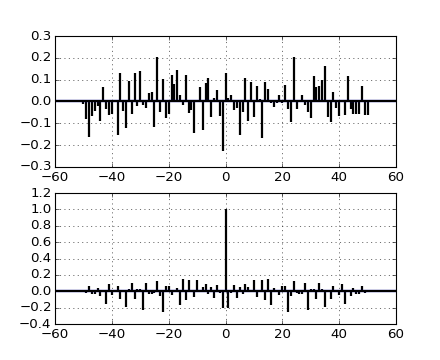`add_artist`(a)

Add any `Artist` to the axes.

Use `add_artist` only for artists for which there is no dedicated “add” method; and if necessary, use a method such as `update_datalim` or `update_datalim_numerix` to manually update the dataLim if the artist is to be included in autoscaling.

Returns the artist.

`add_callback`(func)

Adds a callback function that will be called whenever one of the `Artist`‘s properties changes.

Returns an id that is useful for removing the callback with `remove_callback()` later.

`add_collection`(collection, autolim=True)

Add a `Collection` instance to the axes.

Returns the collection.

`add_container`(container)

Add a `Container` instance to the axes.

Returns the collection.

`add_image`(image)

Add a `AxesImage` to the axes.

Returns the image.

`add_line`(line)

Add a `Line2D` to the list of plot lines

Returns the line.

`add_patch`(p)

Add a `Patch` p to the list of axes patches; the clipbox will be set to the Axes clipping box. If the transform is not set, it will be set to `transData`.

Returns the patch.

`add_table`(tab)

Add a `Table` instance to the list of axes tables

Returns the table.

`aname` = u'Artist'
`angle_spectrum`(x, Fs=None, Fc=None, window=None, pad_to=None, sides=None, **kwargs)

Plot the angle spectrum.

Call signature:

```angle_spectrum(x, Fs=2, Fc=0,  window=mlab.window_hanning,
```

Compute the angle spectrum (wrapped phase spectrum) of x. Data is padded to a length of pad_to and the windowing function window is applied to the signal.

x: 1-D array or sequence
Array or sequence containing the data

Keyword arguments:

Fs: scalar
The sampling frequency (samples per time unit). It is used to calculate the Fourier frequencies, freqs, in cycles per time unit. The default value is 2.
window: callable or ndarray
A function or a vector of length NFFT. To create window vectors see `window_hanning()`, `window_none()`, `numpy.blackman()`, `numpy.hamming()`, `numpy.bartlett()`, `scipy.signal()`, `scipy.signal.get_window()`, etc. The default is `window_hanning()`. If a function is passed as the argument, it must take a data segment as an argument and return the windowed version of the segment.
sides: [ ‘default’ | ‘onesided’ | ‘twosided’ ]
Specifies which sides of the spectrum to return. Default gives the default behavior, which returns one-sided for real data and both for complex data. ‘onesided’ forces the return of a one-sided spectrum, while ‘twosided’ forces two-sided.
The number of points to which the data segment is padded when performing the FFT. While not increasing the actual resolution of the spectrum (the minimum distance between resolvable peaks), this can give more points in the plot, allowing for more detail. This corresponds to the n parameter in the call to fft(). The default is None, which sets pad_to equal to the length of the input signal (i.e. no padding).
Fc: integer
The center frequency of x (defaults to 0), which offsets the x extents of the plot to reflect the frequency range used when a signal is acquired and then filtered and downsampled to baseband.

Returns the tuple (spectrum, freqs, line):

spectrum: 1-D array
The values for the angle spectrum in radians (real valued)
freqs: 1-D array
The frequencies corresponding to the elements in spectrum
line: a `Line2D` instance
The line created by this function

kwargs control the `Line2D` properties:

Property Description
`agg_filter` unknown
`alpha` float (0.0 transparent through 1.0 opaque)
`animated` [True | False]
`antialiased` or aa [True | False]
`axes` an `Axes` instance
`clip_box` a `matplotlib.transforms.Bbox` instance
`clip_on` [True | False]
`clip_path` [ (`Path`, `Transform`) | `Patch` | None ]
`color` or c any matplotlib color
`contains` a callable function
`dash_capstyle` [‘butt’ | ‘round’ | ‘projecting’]
`dash_joinstyle` [‘miter’ | ‘round’ | ‘bevel’]
`dashes` sequence of on/off ink in points
`drawstyle` [‘default’ | ‘steps’ | ‘steps-pre’ | ‘steps-mid’ | ‘steps-post’]
`figure` a `matplotlib.figure.Figure` instance
`fillstyle` [‘full’ | ‘left’ | ‘right’ | ‘bottom’ | ‘top’ | ‘none’]
`gid` an id string
`label` string or anything printable with ‘%s’ conversion.
`linestyle` or ls [`'-'` | `'--'` | `'-.'` | `':'` | `'None'` | `' '` | `''`]
`linewidth` or lw float value in points
`lod` [True | False]
`marker` unknown
`markeredgecolor` or mec any matplotlib color
`markeredgewidth` or mew float value in points
`markerfacecolor` or mfc any matplotlib color
`markerfacecoloralt` or mfcalt any matplotlib color
`markersize` or ms float
`markevery` unknown
`path_effects` unknown
`picker` float distance in points or callable pick function `fn(artist, event)`
`pickradius` float distance in points
`rasterized` [True | False | None]
`sketch_params` unknown
`snap` unknown
`solid_capstyle` [‘butt’ | ‘round’ | ‘projecting’]
`solid_joinstyle` [‘miter’ | ‘round’ | ‘bevel’]
`transform` a `matplotlib.transforms.Transform` instance
`url` a url string
`visible` [True | False]
`xdata` 1D array
`ydata` 1D array
`zorder` any number

Example: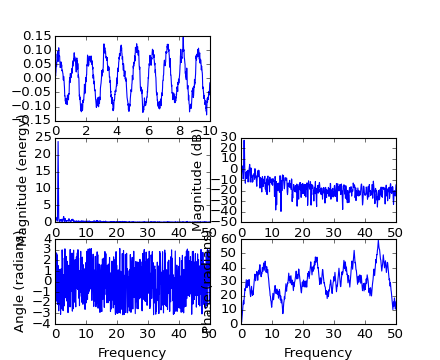`magnitude_spectrum()`
`angle_spectrum()` plots the magnitudes of the corresponding frequencies.
`phase_spectrum()`
`phase_spectrum()` plots the unwrapped version of this function.
`specgram()`
`specgram()` can plot the angle spectrum of segments within the signal in a colormap.
`annotate`(*args, **kwargs)

Create an annotation: a piece of text referring to a data point.

Parameters: s : string label xy : (x, y) position of element to annotate xytext : (x, y) , optional, default: None position of the label `s` xycoords : string, optional, default: “data” string that indicates what type of coordinates `xy` is. Examples: “figure points”, “figure pixels”, “figure fraction”, “axes points”, .... See `matplotlib.text.Annotation` for more details. textcoords : string, optional string that indicates what type of coordinates `text` is. Examples: “figure points”, “figure pixels”, “figure fraction”, “axes points”, .... See `matplotlib.text.Annotation` for more details. Default is None. arrowprops : `matplotlib.lines.Line2D` properties, optional Dictionary of line properties for the arrow that connects the annotation to the point. If the dictionnary has a key `arrowstyle`, a `FancyArrowPatch` instance is created and drawn. See `matplotlib.text.Annotation` for more details on valid options. Default is None.

Notes

arrowprops, if not None, is a dictionary of line properties (see `matplotlib.lines.Line2D`) for the arrow that connects annotation to the point.

If the dictionary has a key arrowstyle, a `FancyArrowPatch` instance is created with the given dictionary and is drawn. Otherwise, a `YAArrow` patch instance is created and drawn. Valid keys for `YAArrow` are:

Key Description
width the width of the arrow in points
frac the fraction of the arrow length occupied by the head
headwidth the width of the base of the arrow head in points
shrink oftentimes it is convenient to have the arrowtip and base a bit away from the text and point being annotated. If d is the distance between the text and annotated point, shrink will shorten the arrow so the tip and base are shink percent of the distance d away from the endpoints. ie, `shrink=0.05 is 5%`
? any key for `matplotlib.patches.polygon`

Valid keys for `FancyArrowPatch` are:

Key Description
arrowstyle the arrow style
connectionstyle the connection style
relpos default is (0.5, 0.5)
patchA default is bounding box of the text
patchB default is None
shrinkA default is 2 points
shrinkB default is 2 points
mutation_scale default is text size (in points)
mutation_aspect default is 1.
? any key for `matplotlib.patches.PathPatch`

xycoords and textcoords are strings that indicate the coordinates of xy and xytext, and may be one of the following values:

Property Description
‘figure points’ points from the lower left corner of the figure
‘figure pixels’ pixels from the lower left corner of the figure
‘figure fraction’ 0,0 is lower left of figure and 1,1 is upper right
‘axes points’ points from lower left corner of axes
‘axes pixels’ pixels from lower left corner of axes
‘axes fraction’ 0,0 is lower left of axes and 1,1 is upper right
‘data’ use the coordinate system of the object being annotated (default)
‘offset points’ Specify an offset (in points) from the xy value
‘polar’ you can specify theta, r for the annotation, even in cartesian plots. Note that if you are using a polar axes, you do not need to specify polar for the coordinate system since that is the native “data” coordinate system.

If a ‘points’ or ‘pixels’ option is specified, values will be added to the bottom-left and if negative, values will be subtracted from the top-right. e.g.:

```# 10 points to the right of the left border of the axes and
# 5 points below the top border
xy=(10,-5), xycoords='axes points'
```

You may use an instance of `Transform` or `Artist`. See Annotating Axes for more details.

The annotation_clip attribute controls the visibility of the annotation when it goes outside the axes area. If `True`, the annotation will only be drawn when the xy is inside the axes. If `False`, the annotation will always be drawn regardless of its position. The default is `None`, which behave as `True` only if xycoords is “data”.

Additional kwargs are `Text` properties:

Property Description
`agg_filter` unknown
`alpha` float (0.0 transparent through 1.0 opaque)
`animated` [True | False]
`axes` an `Axes` instance
`backgroundcolor` any matplotlib color
`bbox` rectangle prop dict
`clip_box` a `matplotlib.transforms.Bbox` instance
`clip_on` [True | False]
`clip_path` [ (`Path`, `Transform`) | `Patch` | None ]
`color` any matplotlib color
`contains` a callable function
`family` or fontfamily or fontname or name [FONTNAME | ‘serif’ | ‘sans-serif’ | ‘cursive’ | ‘fantasy’ | ‘monospace’ ]
`figure` a `matplotlib.figure.Figure` instance
`fontproperties` or font_properties a `matplotlib.font_manager.FontProperties` instance
`gid` an id string
`horizontalalignment` or ha [ ‘center’ | ‘right’ | ‘left’ ]
`label` string or anything printable with ‘%s’ conversion.
`linespacing` float (multiple of font size)
`lod` [True | False]
`multialignment` [‘left’ | ‘right’ | ‘center’ ]
`path_effects` unknown
`picker` [None|float|boolean|callable]
`position` (x,y)
`rasterized` [True | False | None]
`rotation` [ angle in degrees | ‘vertical’ | ‘horizontal’ ]
`rotation_mode` unknown
`size` or fontsize [size in points | ‘xx-small’ | ‘x-small’ | ‘small’ | ‘medium’ | ‘large’ | ‘x-large’ | ‘xx-large’ ]
`sketch_params` unknown
`snap` unknown
`stretch` or fontstretch [a numeric value in range 0-1000 | ‘ultra-condensed’ | ‘extra-condensed’ | ‘condensed’ | ‘semi-condensed’ | ‘normal’ | ‘semi-expanded’ | ‘expanded’ | ‘extra-expanded’ | ‘ultra-expanded’ ]
`style` or fontstyle [ ‘normal’ | ‘italic’ | ‘oblique’]
`text` string or anything printable with ‘%s’ conversion.
`transform` `Transform` instance
`url` a url string
`variant` or fontvariant [ ‘normal’ | ‘small-caps’ ]
`verticalalignment` or va or ma [ ‘center’ | ‘top’ | ‘bottom’ | ‘baseline’ ]
`visible` [True | False]
`weight` or fontweight [a numeric value in range 0-1000 | ‘ultralight’ | ‘light’ | ‘normal’ | ‘regular’ | ‘book’ | ‘medium’ | ‘roman’ | ‘semibold’ | ‘demibold’ | ‘demi’ | ‘bold’ | ‘heavy’ | ‘extra bold’ | ‘black’ ]
`x` float
`y` float
`zorder` any number

Examples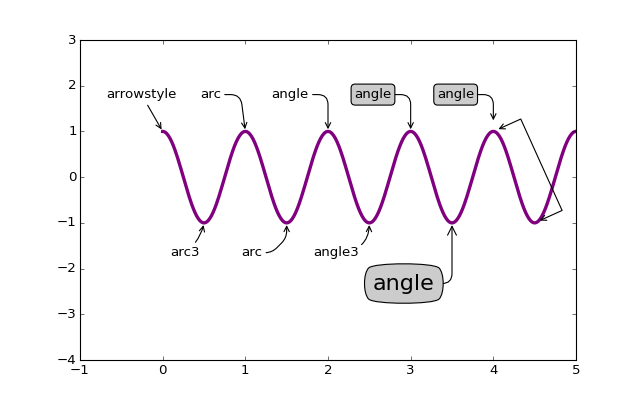(png, hires.png, pdf)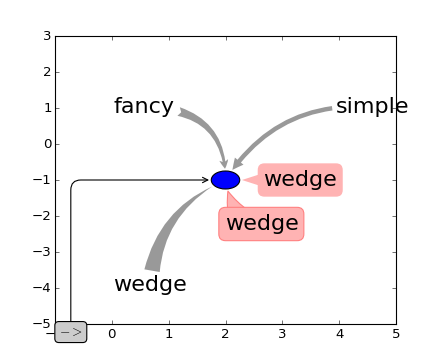(png, hires.png, pdf)

`apply_aspect`(position=None)

Use `_aspect()` and `_adjustable()` to modify the axes box or the view limits.

`arrow`(x, y, dx, dy, **kwargs)

Add an arrow to the axes.

Call signature:

```arrow(x, y, dx, dy, **kwargs)
```

Draws arrow on specified axis from (x, y) to (x + dx, y + dy). Uses FancyArrow patch to construct the arrow.

The resulting arrow is affected by the axes aspect ratio and limits. This may produce an arrow whose head is not square with its stem. To create an arrow whose head is square with its stem, use `annotate()` for example:

```ax.annotate("", xy=(0.5, 0.5), xytext=(0, 0),
arrowprops=dict(arrowstyle="->"))
```

Optional kwargs control the arrow construction and properties:

Constructor arguments
width: float (default: 0.001)
width of full arrow tail
length_includes_head: [True | False] (default: False)
True if head is to be counted in calculating the length.
head_width: float or None (default: 3*width)
total width of the full arrow head
shape: [‘full’, ‘left’, ‘right’] (default: ‘full’)
draw the left-half, right-half, or full arrow
overhang: float (default: 0)
fraction that the arrow is swept back (0 overhang means triangular shape). Can be negative or greater than one.
head_starts_at_zero: [True | False] (default: False)
if True, the head starts being drawn at coordinate 0 instead of ending at coordinate 0.

Other valid kwargs (inherited from `Patch`) are:

Property Description
`agg_filter` unknown
`alpha` float or None
`animated` [True | False]
`antialiased` or aa [True | False] or None for default
`axes` an `Axes` instance
`capstyle` [‘butt’ | ‘round’ | ‘projecting’]
`clip_box` a `matplotlib.transforms.Bbox` instance
`clip_on` [True | False]
`clip_path` [ (`Path`, `Transform`) | `Patch` | None ]
`color` matplotlib color spec
`contains` a callable function
`edgecolor` or ec mpl color spec, or None for default, or ‘none’ for no color
`facecolor` or fc mpl color spec, or None for default, or ‘none’ for no color
`figure` a `matplotlib.figure.Figure` instance
`fill` [True | False]
`gid` an id string
`hatch` [‘/’ | ‘\’ | ‘|’ | ‘-‘ | ‘+’ | ‘x’ | ‘o’ | ‘O’ | ‘.’ | ‘*’]
`joinstyle` [‘miter’ | ‘round’ | ‘bevel’]
`label` string or anything printable with ‘%s’ conversion.
`linestyle` or ls [‘solid’ | ‘dashed’ | ‘dashdot’ | ‘dotted’]
`linewidth` or lw float or None for default
`lod` [True | False]
`path_effects` unknown
`picker` [None|float|boolean|callable]
`rasterized` [True | False | None]
`sketch_params` unknown
`snap` unknown
`transform` `Transform` instance
`url` a url string
`visible` [True | False]
`zorder` any number

Example: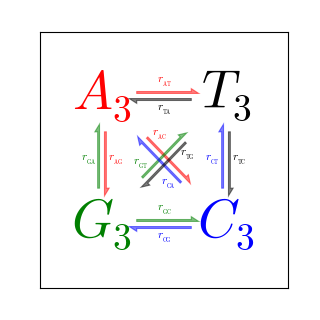`autoscale`(enable=True, axis=u'both', tight=None)

Autoscale the axis view to the data (toggle).

Convenience method for simple axis view autoscaling. It turns autoscaling on or off, and then, if autoscaling for either axis is on, it performs the autoscaling on the specified axis or axes.

enable: [True | False | None]
True (default) turns autoscaling on, False turns it off. None leaves the autoscaling state unchanged.
axis: [‘x’ | ‘y’ | ‘both’]
which axis to operate on; default is ‘both’
tight: [True | False | None]
If True, set view limits to data limits; if False, let the locator and margins expand the view limits; if None, use tight scaling if the only artist is an image, otherwise treat tight as False. The tight setting is retained for future autoscaling until it is explicitly changed.

Returns None.

`autoscale_view`(tight=None, scalex=True, scaley=True)

Autoscale the view limits using the data limits. You can selectively autoscale only a single axis, eg, the xaxis by setting scaley to False. The autoscaling preserves any axis direction reversal that has already been done.

The data limits are not updated automatically when artist data are changed after the artist has been added to an Axes instance. In that case, use `matplotlib.axes.Axes.relim()` prior to calling autoscale_view.

`axhline`(y=0, xmin=0, xmax=1, **kwargs)

Add a horizontal line across the axis.

Parameters: y : scalar, optional, default: 0 y position in data coordinates of the horizontal line. xmin : scalar, optional, default: 0 Should be between 0 and 1, 0 being the far left of the plot, 1 the far right of the plot. xmax : scalar, optional, default: 1 Should be between 0 and 1, 0 being the far left of the plot, 1 the far right of the plot. `~matplotlib.lines.Line2D` :

`axhspan`
for example plot and source code

Notes

kwargs are the same as kwargs to plot, and can be used to control the line properties. e.g.,

Examples

• draw a thick red hline at ‘y’ = 0 that spans the xrange:

```>>> axhline(linewidth=4, color='r')
```
• draw a default hline at ‘y’ = 1 that spans the xrange:

```>>> axhline(y=1)
```
• draw a default hline at ‘y’ = .5 that spans the the middle half of the xrange:

```>>> axhline(y=.5, xmin=0.25, xmax=0.75)
```

Valid kwargs are `Line2D` properties, with the exception of ‘transform’:

Property Description
`agg_filter` unknown
`alpha` float (0.0 transparent through 1.0 opaque)
`animated` [True | False]
`antialiased` or aa [True | False]
`axes` an `Axes` instance
`clip_box` a `matplotlib.transforms.Bbox` instance
`clip_on` [True | False]
`clip_path` [ (`Path`, `Transform`) | `Patch` | None ]
`color` or c any matplotlib color
`contains` a callable function
`dash_capstyle` [‘butt’ | ‘round’ | ‘projecting’]
`dash_joinstyle` [‘miter’ | ‘round’ | ‘bevel’]
`dashes` sequence of on/off ink in points
`drawstyle` [‘default’ | ‘steps’ | ‘steps-pre’ | ‘steps-mid’ | ‘steps-post’]
`figure` a `matplotlib.figure.Figure` instance
`fillstyle` [‘full’ | ‘left’ | ‘right’ | ‘bottom’ | ‘top’ | ‘none’]
`gid` an id string
`label` string or anything printable with ‘%s’ conversion.
`linestyle` or ls [`'-'` | `'--'` | `'-.'` | `':'` | `'None'` | `' '` | `''`]
`linewidth` or lw float value in points
`lod` [True | False]
`marker` unknown
`markeredgecolor` or mec any matplotlib color
`markeredgewidth` or mew float value in points
`markerfacecolor` or mfc any matplotlib color
`markerfacecoloralt` or mfcalt any matplotlib color
`markersize` or ms float
`markevery` unknown
`path_effects` unknown
`picker` float distance in points or callable pick function `fn(artist, event)`
`pickradius` float distance in points
`rasterized` [True | False | None]
`sketch_params` unknown
`snap` unknown
`solid_capstyle` [‘butt’ | ‘round’ | ‘projecting’]
`solid_joinstyle` [‘miter’ | ‘round’ | ‘bevel’]
`transform` a `matplotlib.transforms.Transform` instance
`url` a url string
`visible` [True | False]
`xdata` 1D array
`ydata` 1D array
`zorder` any number
`axhspan`(ymin, ymax, xmin=0, xmax=1, **kwargs)

Add a horizontal span (rectangle) across the axis.

Call signature:

```axhspan(ymin, ymax, xmin=0, xmax=1, **kwargs)
```

y coords are in data units and x coords are in axes (relative 0-1) units.

Draw a horizontal span (rectangle) from ymin to ymax. With the default values of xmin = 0 and xmax = 1, this always spans the xrange, regardless of the xlim settings, even if you change them, e.g., with the `set_xlim()` command. That is, the horizontal extent is in axes coords: 0=left, 0.5=middle, 1.0=right but the y location is in data coordinates.

Return value is a `matplotlib.patches.Polygon` instance.

Examples:

• draw a gray rectangle from y = 0.25-0.75 that spans the horizontal extent of the axes:

```>>> axhspan(0.25, 0.75, facecolor='0.5', alpha=0.5)
```

Valid kwargs are `Polygon` properties:

Property Description
`agg_filter` unknown
`alpha` float or None
`animated` [True | False]
`antialiased` or aa [True | False] or None for default
`axes` an `Axes` instance
`capstyle` [‘butt’ | ‘round’ | ‘projecting’]
`clip_box` a `matplotlib.transforms.Bbox` instance
`clip_on` [True | False]
`clip_path` [ (`Path`, `Transform`) | `Patch` | None ]
`color` matplotlib color spec
`contains` a callable function
`edgecolor` or ec mpl color spec, or None for default, or ‘none’ for no color
`facecolor` or fc mpl color spec, or None for default, or ‘none’ for no color
`figure` a `matplotlib.figure.Figure` instance
`fill` [True | False]
`gid` an id string
`hatch` [‘/’ | ‘\’ | ‘|’ | ‘-‘ | ‘+’ | ‘x’ | ‘o’ | ‘O’ | ‘.’ | ‘*’]
`joinstyle` [‘miter’ | ‘round’ | ‘bevel’]
`label` string or anything printable with ‘%s’ conversion.
`linestyle` or ls [‘solid’ | ‘dashed’ | ‘dashdot’ | ‘dotted’]
`linewidth` or lw float or None for default
`lod` [True | False]
`path_effects` unknown
`picker` [None|float|boolean|callable]
`rasterized` [True | False | None]
`sketch_params` unknown
`snap` unknown
`transform` `Transform` instance
`url` a url string
`visible` [True | False]
`zorder` any number

Example: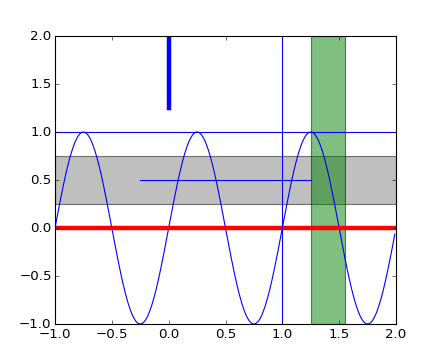`axis`(*v, **kwargs)

Convenience method for manipulating the x and y view limits and the aspect ratio of the plot. For details, see `axis()`.

kwargs are passed on to `set_xlim()` and `set_ylim()`

`axvline`(x=0, ymin=0, ymax=1, **kwargs)

Add a vertical line across the axes.

Parameters: x : scalar, optional, default: 0 x position in data coordinates of the vertical line. ymin : scalar, optional, default: 0 Should be between 0 and 1, 0 being the far left of the plot, 1 the far right of the plot. ymax : scalar, optional, default: 1 Should be between 0 and 1, 0 being the far left of the plot, 1 the far right of the plot. `~matplotlib.lines.Line2D` :

`axhspan`
for example plot and source code

Examples

• draw a thick red vline at x = 0 that spans the yrange:

```>>> axvline(linewidth=4, color='r')
```
• draw a default vline at x = 1 that spans the yrange:

```>>> axvline(x=1)
```
• draw a default vline at x = .5 that spans the the middle half of the yrange:

```>>> axvline(x=.5, ymin=0.25, ymax=0.75)
```

Valid kwargs are `Line2D` properties, with the exception of ‘transform’:

Property Description
`agg_filter` unknown
`alpha` float (0.0 transparent through 1.0 opaque)
`animated` [True | False]
`antialiased` or aa [True | False]
`axes` an `Axes` instance
`clip_box` a `matplotlib.transforms.Bbox` instance
`clip_on` [True | False]
`clip_path` [ (`Path`, `Transform`) | `Patch` | None ]
`color` or c any matplotlib color
`contains` a callable function
`dash_capstyle` [‘butt’ | ‘round’ | ‘projecting’]
`dash_joinstyle` [‘miter’ | ‘round’ | ‘bevel’]
`dashes` sequence of on/off ink in points
`drawstyle` [‘default’ | ‘steps’ | ‘steps-pre’ | ‘steps-mid’ | ‘steps-post’]
`figure` a `matplotlib.figure.Figure` instance
`fillstyle` [‘full’ | ‘left’ | ‘right’ | ‘bottom’ | ‘top’ | ‘none’]
`gid` an id string
`label` string or anything printable with ‘%s’ conversion.
`linestyle` or ls [`'-'` | `'--'` | `'-.'` | `':'` | `'None'` | `' '` | `''`]
`linewidth` or lw float value in points
`lod` [True | False]
`marker` unknown
`markeredgecolor` or mec any matplotlib color
`markeredgewidth` or mew float value in points
`markerfacecolor` or mfc any matplotlib color
`markerfacecoloralt` or mfcalt any matplotlib color
`markersize` or ms float
`markevery` unknown
`path_effects` unknown
`picker` float distance in points or callable pick function `fn(artist, event)`
`pickradius` float distance in points
`rasterized` [True | False | None]
`sketch_params` unknown
`snap` unknown
`solid_capstyle` [‘butt’ | ‘round’ | ‘projecting’]
`solid_joinstyle` [‘miter’ | ‘round’ | ‘bevel’]
`transform` a `matplotlib.transforms.Transform` instance
`url` a url string
`visible` [True | False]
`xdata` 1D array
`ydata` 1D array
`zorder` any number
`axvspan`(xmin, xmax, ymin=0, ymax=1, **kwargs)

Add a vertical span (rectangle) across the axes.

Call signature:

```axvspan(xmin, xmax, ymin=0, ymax=1, **kwargs)
```

x coords are in data units and y coords are in axes (relative 0-1) units.

Draw a vertical span (rectangle) from xmin to xmax. With the default values of ymin = 0 and ymax = 1, this always spans the yrange, regardless of the ylim settings, even if you change them, e.g., with the `set_ylim()` command. That is, the vertical extent is in axes coords: 0=bottom, 0.5=middle, 1.0=top but the y location is in data coordinates.

Return value is the `matplotlib.patches.Polygon` instance.

Examples:

• draw a vertical green translucent rectangle from x=1.25 to 1.55 that spans the yrange of the axes:

```>>> axvspan(1.25, 1.55, facecolor='g', alpha=0.5)
```

Valid kwargs are `Polygon` properties:

Property Description
`agg_filter` unknown
`alpha` float or None
`animated` [True | False]
`antialiased` or aa [True | False] or None for default
`axes` an `Axes` instance
`capstyle` [‘butt’ | ‘round’ | ‘projecting’]
`clip_box` a `matplotlib.transforms.Bbox` instance
`clip_on` [True | False]
`clip_path` [ (`Path`, `Transform`) | `Patch` | None ]
`color` matplotlib color spec
`contains` a callable function
`edgecolor` or ec mpl color spec, or None for default, or ‘none’ for no color
`facecolor` or fc mpl color spec, or None for default, or ‘none’ for no color
`figure` a `matplotlib.figure.Figure` instance
`fill` [True | False]
`gid` an id string
`hatch` [‘/’ | ‘\’ | ‘|’ | ‘-‘ | ‘+’ | ‘x’ | ‘o’ | ‘O’ | ‘.’ | ‘*’]
`joinstyle` [‘miter’ | ‘round’ | ‘bevel’]
`label` string or anything printable with ‘%s’ conversion.
`linestyle` or ls [‘solid’ | ‘dashed’ | ‘dashdot’ | ‘dotted’]
`linewidth` or lw float or None for default
`lod` [True | False]
`path_effects` unknown
`picker` [None|float|boolean|callable]
`rasterized` [True | False | None]
`sketch_params` unknown
`snap` unknown
`transform` `Transform` instance
`url` a url string
`visible` [True | False]
`zorder` any number

`axhspan()`
for example plot and source code
`bar`(left, height, width=0.8, bottom=None, **kwargs)

Make a bar plot.

Make a bar plot with rectangles bounded by:

`left`, `left` + `width`, `bottom`, `bottom` + `height`
(left, right, bottom and top edges)
Parameters: left : sequence of scalars the x coordinates of the left sides of the bars height : sequence of scalars the heights of the bars width : scalar or array-like, optional, default: 0.8 the width(s) of the bars bottom : scalar or array-like, optional, default: None the y coordinate(s) of the bars color : scalar or array-like, optional the colors of the bar faces edgecolor : scalar or array-like, optional the colors of the bar edges linewidth : scalar or array-like, optional, default: None width of bar edge(s). If None, use default linewidth; If 0, don’t draw edges. xerr : scalar or array-like, optional, default: None if not None, will be used to generate errorbar(s) on the bar chart yerr : scalar or array-like, optional, default: None if not None, will be used to generate errorbar(s) on the bar chart ecolor : scalar or array-like, optional, default: None specifies the color of errorbar(s) capsize : integer, optional, default: 3 determines the length in points of the error bar caps error_kw : : dictionary of kwargs to be passed to errorbar method. ecolor and capsize may be specified here rather than as independent kwargs. align : [‘edge’ | ‘center’], optional, default: ‘edge’ If `edge`, aligns bars by their left edges (for vertical bars) and by their bottom edges (for horizontal bars). If `center`, interpret the `left` argument as the coordinates of the centers of the bars. orientation : ‘vertical’ | ‘horizontal’, optional, default: ‘vertical’ The orientation of the bars. log : boolean, optional, default: False If true, sets the axis to be log scale `matplotlib.patches.Rectangle` instances. :

`barh`
Plot a horizontal bar plot.

Notes

The optional arguments `color`, `edgecolor`, `linewidth`, `xerr`, and `yerr` can be either scalars or sequences of length equal to the number of bars. This enables you to use bar as the basis for stacked bar charts, or candlestick plots. Detail: `xerr` and `yerr` are passed directly to `errorbar()`, so they can also have shape 2xN for independent specification of lower and upper errors.

Other optional kwargs:

Property Description
`agg_filter` unknown
`alpha` float or None
`animated` [True | False]
`antialiased` or aa [True | False] or None for default
`axes` an `Axes` instance
`capstyle` [‘butt’ | ‘round’ | ‘projecting’]
`clip_box` a `matplotlib.transforms.Bbox` instance
`clip_on` [True | False]
`clip_path` [ (`Path`, `Transform`) | `Patch` | None ]
`color` matplotlib color spec
`contains` a callable function
`edgecolor` or ec mpl color spec, or None for default, or ‘none’ for no color
`facecolor` or fc mpl color spec, or None for default, or ‘none’ for no color
`figure` a `matplotlib.figure.Figure` instance
`fill` [True | False]
`gid` an id string
`hatch` [‘/’ | ‘\’ | ‘|’ | ‘-‘ | ‘+’ | ‘x’ | ‘o’ | ‘O’ | ‘.’ | ‘*’]
`joinstyle` [‘miter’ | ‘round’ | ‘bevel’]
`label` string or anything printable with ‘%s’ conversion.
`linestyle` or ls [‘solid’ | ‘dashed’ | ‘dashdot’ | ‘dotted’]
`linewidth` or lw float or None for default
`lod` [True | False]
`path_effects` unknown
`picker` [None|float|boolean|callable]
`rasterized` [True | False | None]
`sketch_params` unknown
`snap` unknown
`transform` `Transform` instance
`url` a url string
`visible` [True | False]
`zorder` any number

Examples

Example: A stacked bar chart.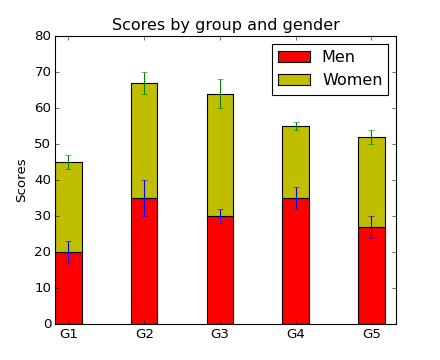`barbs`(*args, **kw)

Plot a 2-D field of barbs.

Call signatures:

```barb(U, V, **kw)
barb(U, V, C, **kw)
barb(X, Y, U, V, **kw)
barb(X, Y, U, V, C, **kw)
```

Arguments:

X, Y:
The x and y coordinates of the barb locations (default is head of barb; see pivot kwarg)
U, V:
Give the x and y components of the barb shaft
C:
An optional array used to map colors to the barbs

All arguments may be 1-D or 2-D arrays or sequences. If X and Y are absent, they will be generated as a uniform grid. If U and V are 2-D arrays but X and Y are 1-D, and if `len(X)` and `len(Y)` match the column and row dimensions of U, then X and Y will be expanded with `numpy.meshgrid()`.

U, V, C may be masked arrays, but masked X, Y are not supported at present.

Keyword arguments:

length:
Length of the barb in points; the other parts of the barb are scaled against this. Default is 9
pivot: [ ‘tip’ | ‘middle’ ]
The part of the arrow that is at the grid point; the arrow rotates about this point, hence the name pivot. Default is ‘tip’
barbcolor: [ color | color sequence ]
Specifies the color all parts of the barb except any flags. This parameter is analagous to the edgecolor parameter for polygons, which can be used instead. However this parameter will override facecolor.
flagcolor: [ color | color sequence ]
Specifies the color of any flags on the barb. This parameter is analagous to the facecolor parameter for polygons, which can be used instead. However this parameter will override facecolor. If this is not set (and C has not either) then flagcolor will be set to match barbcolor so that the barb has a uniform color. If C has been set, flagcolor has no effect.
sizes:

A dictionary of coefficients specifying the ratio of a given feature to the length of the barb. Only those values one wishes to override need to be included. These features include:

• ‘spacing’ - space between features (flags, full/half barbs)
• ‘height’ - height (distance from shaft to top) of a flag or full barb
• ‘width’ - width of a flag, twice the width of a full barb
• ‘emptybarb’ - radius of the circle used for low magnitudes
fill_empty:
A flag on whether the empty barbs (circles) that are drawn should be filled with the flag color. If they are not filled, they will be drawn such that no color is applied to the center. Default is False
rounding:
A flag to indicate whether the vector magnitude should be rounded when allocating barb components. If True, the magnitude is rounded to the nearest multiple of the half-barb increment. If False, the magnitude is simply truncated to the next lowest multiple. Default is True
barb_increments:

A dictionary of increments specifying values to associate with different parts of the barb. Only those values one wishes to override need to be included.

• ‘half’ - half barbs (Default is 5)
• ‘full’ - full barbs (Default is 10)
• ‘flag’ - flags (default is 50)
flip_barb:
Either a single boolean flag or an array of booleans. Single boolean indicates whether the lines and flags should point opposite to normal for all barbs. An array (which should be the same size as the other data arrays) indicates whether to flip for each individual barb. Normal behavior is for the barbs and lines to point right (comes from wind barbs having these features point towards low pressure in the Northern Hemisphere.) Default is False

Barbs are traditionally used in meteorology as a way to plot the speed and direction of wind observations, but can technically be used to plot any two dimensional vector quantity. As opposed to arrows, which give vector magnitude by the length of the arrow, the barbs give more quantitative information about the vector magnitude by putting slanted lines or a triangle for various increments in magnitude, as show schematically below:

```:     /\    \
:    /  \    \
:   /    \    \    \
:  /      \    \    \
: ------------------------------
```

The largest increment is given by a triangle (or “flag”). After those come full lines (barbs). The smallest increment is a half line. There is only, of course, ever at most 1 half line. If the magnitude is small and only needs a single half-line and no full lines or triangles, the half-line is offset from the end of the barb so that it can be easily distinguished from barbs with a single full line. The magnitude for the barb shown above would nominally be 65, using the standard increments of 50, 10, and 5.

linewidths and edgecolors can be used to customize the barb. Additional `PolyCollection` keyword arguments:

Property Description
`agg_filter` unknown
`alpha` float or None
`animated` [True | False]
`antialiased` or antialiaseds Boolean or sequence of booleans
`array` unknown
`axes` an `Axes` instance
`clim` a length 2 sequence of floats
`clip_box` a `matplotlib.transforms.Bbox` instance
`clip_on` [True | False]
`clip_path` [ (`Path`, `Transform`) | `Patch` | None ]
`cmap` a colormap or registered colormap name
`color` matplotlib color arg or sequence of rgba tuples
`contains` a callable function
`edgecolor` or edgecolors matplotlib color arg or sequence of rgba tuples
`facecolor` or facecolors matplotlib color arg or sequence of rgba tuples
`figure` a `matplotlib.figure.Figure` instance
`gid` an id string
`hatch` [ ‘/’ | ‘\’ | ‘|’ | ‘-‘ | ‘+’ | ‘x’ | ‘o’ | ‘O’ | ‘.’ | ‘*’ ]
`label` string or anything printable with ‘%s’ conversion.
`linestyle` or linestyles or dashes [‘solid’ | ‘dashed’, ‘dashdot’, ‘dotted’ | (offset, on-off-dash-seq) ]
`linewidth` or lw or linewidths float or sequence of floats
`lod` [True | False]
`norm` unknown
`offset_position` unknown
`offsets` float or sequence of floats
`path_effects` unknown
`picker` [None|float|boolean|callable]
`pickradius` unknown
`rasterized` [True | False | None]
`sketch_params` unknown
`snap` unknown
`transform` `Transform` instance
`url` a url string
`urls` unknown
`visible` [True | False]
`zorder` any number

Example: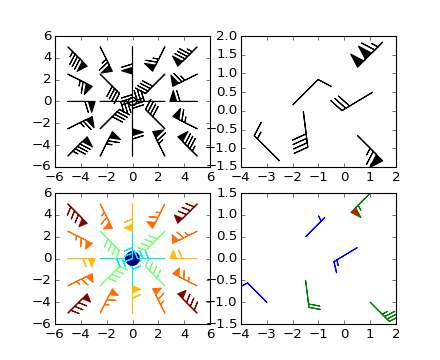(png, hires.png, pdf)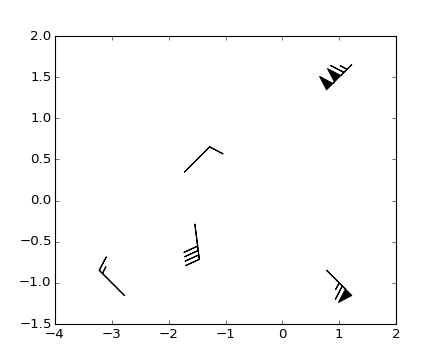(png, hires.png, pdf)

`barh`(bottom, width, height=0.8, left=None, **kwargs)

Make a horizontal bar plot.

Make a horizontal bar plot with rectangles bounded by:

`left`, `left` + `width`, `bottom`, `bottom` + `height`
(left, right, bottom and top edges)

`bottom`, `width`, `height`, and `left` can be either scalars or sequences

Parameters: Returns: bottom : scalar or array-like the y coordinate(s) of the bars width : scalar or array-like the width(s) of the bars height : sequence of scalars, optional, default: 0.8 the heights of the bars left : sequence of scalars the x coordinates of the left sides of the bars `matplotlib.patches.Rectangle` instances. : color : scalar or array-like, optional the colors of the bars edgecolor : scalar or array-like, optional the colors of the bar edges linewidth : scalar or array-like, optional, default: None width of bar edge(s). If None, use default linewidth; If 0, don’t draw edges. xerr : scalar or array-like, optional, default: None if not None, will be used to generate errorbar(s) on the bar chart yerr : scalar or array-like, optional, default: None if not None, will be used to generate errorbar(s) on the bar chart ecolor : scalar or array-like, optional, default: None specifies the color of errorbar(s) capsize : integer, optional, default: 3 determines the length in points of the error bar caps error_kw : : dictionary of kwargs to be passed to errorbar method. `ecolor` and `capsize` may be specified here rather than as independent kwargs. align : [‘edge’ | ‘center’], optional, default: ‘edge’ If `edge`, aligns bars by their left edges (for vertical bars) and by their bottom edges (for horizontal bars). If `center`, interpret the `left` argument as the coordinates of the centers of the bars. orientation : ‘vertical’ | ‘horizontal’, optional, default: ‘vertical’ The orientation of the bars. log : boolean, optional, default: False If true, sets the axis to be log scale

`bar`
Plot a vertical bar plot.

Notes

The optional arguments `color`, `edgecolor`, `linewidth`, `xerr`, and `yerr` can be either scalars or sequences of length equal to the number of bars. This enables you to use bar as the basis for stacked bar charts, or candlestick plots. Detail: `xerr` and `yerr` are passed directly to `errorbar()`, so they can also have shape 2xN for independent specification of lower and upper errors.

Other optional kwargs:

Property Description
`agg_filter` unknown
`alpha` float or None
`animated` [True | False]
`antialiased` or aa [True | False] or None for default
`axes` an `Axes` instance
`capstyle` [‘butt’ | ‘round’ | ‘projecting’]
`clip_box` a `matplotlib.transforms.Bbox` instance
`clip_on` [True | False]
`clip_path` [ (`Path`, `Transform`) | `Patch` | None ]
`color` matplotlib color spec
`contains` a callable function
`edgecolor` or ec mpl color spec, or None for default, or ‘none’ for no color
`facecolor` or fc mpl color spec, or None for default, or ‘none’ for no color
`figure` a `matplotlib.figure.Figure` instance
`fill` [True | False]
`gid` an id string
`hatch` [‘/’ | ‘\’ | ‘|’ | ‘-‘ | ‘+’ | ‘x’ | ‘o’ | ‘O’ | ‘.’ | ‘*’]
`joinstyle` [‘miter’ | ‘round’ | ‘bevel’]
`label` string or anything printable with ‘%s’ conversion.
`linestyle` or ls [‘solid’ | ‘dashed’ | ‘dashdot’ | ‘dotted’]
`linewidth` or lw float or None for default
`lod` [True | False]
`path_effects` unknown
`picker` [None|float|boolean|callable]
`rasterized` [True | False | None]
`sketch_params` unknown
`snap` unknown
`transform` `Transform` instance
`url` a url string
`visible` [True | False]
`zorder` any number
`boxplot`(x, notch=False, sym=None, vert=True, whis=1.5, positions=None, widths=None, patch_artist=False, bootstrap=None, usermedians=None, conf_intervals=None, meanline=False, showmeans=False, showcaps=True, showbox=True, showfliers=True, boxprops=None, labels=None, flierprops=None, medianprops=None, meanprops=None, capprops=None, whiskerprops=None, manage_xticks=True)

Make a box and whisker plot.

Call signature:

```boxplot(self, x, notch=False, sym='b+', vert=True, whis=1.5,
positions=None, widths=None, patch_artist=False,
bootstrap=None, usermedians=None, conf_intervals=None,
meanline=False, showmeans=False, showcaps=True,
showbox=True, showfliers=True, boxprops=None, labels=None,
flierprops=None, medianprops=None, meanprops=None,
capprops=None, whiskerprops=None, manage_xticks=True):
```

Make a box and whisker plot for each column of x or each vector in sequence x. The box extends from the lower to upper quartile values of the data, with a line at the median. The whiskers extend from the box to show the range of the data. Flier points are those past the end of the whiskers.

Parameters: x : Array or a sequence of vectors. The input data. notch : bool, default = False If False, produces a rectangular box plot. If True, will produce a notched box plot sym : str or None, default = None The default symbol for flier points. Enter an empty string (‘’) if you don’t want to show fliers. If `None`, then the fliers default to ‘b+’ If you want more control use the fliersprop kwarg. vert : bool, default = True If True (default), makes the boxes vertical. If False, makes horizontal boxes. whis : float, sequence (default = 1.5) or string As a float, determines the reach of the whiskers past the first and third quartiles (e.g., Q3 + whis*IQR, IQR = interquartile range, Q3-Q1). Beyond the whiskers, data are considered outliers and are plotted as individual points. Set this to an unreasonably high value to force the whiskers to show the min and max values. Alternatively, set this to an ascending sequence of percentile (e.g., [5, 95]) to set the whiskers at specific percentiles of the data. Finally, whis can be the string ‘range’ to force the whiskers to the min and max of the data. In the edge case that the 25th and 75th percentiles are equivalent, whis will be automatically set to ‘range’. bootstrap : None (default) or integer Specifies whether to bootstrap the confidence intervals around the median for notched boxplots. If bootstrap==None, no bootstrapping is performed, and notches are calculated using a Gaussian-based asymptotic approximation (see McGill, R., Tukey, J.W., and Larsen, W.A., 1978, and Kendall and Stuart, 1967). Otherwise, bootstrap specifies the number of times to bootstrap the median to determine it’s 95% confidence intervals. Values between 1000 and 10000 are recommended. usermedians : array-like or None (default) An array or sequence whose first dimension (or length) is compatible with x. This overrides the medians computed by matplotlib for each element of usermedians that is not None. When an element of usermedians == None, the median will be computed by matplotlib as normal. conf_intervals : array-like or None (default) Array or sequence whose first dimension (or length) is compatible with x and whose second dimension is 2. When the current element of conf_intervals is not None, the notch locations computed by matplotlib are overridden (assuming notch is True). When an element of conf_intervals is None, boxplot compute notches the method specified by the other kwargs (e.g., bootstrap). positions : array-like, default = [1, 2, ..., n] Sets the positions of the boxes. The ticks and limits are automatically set to match the positions. widths : array-like, default = 0.5 Either a scalar or a vector and sets the width of each box. The default is 0.5, or `0.15*(distance between extreme positions)` if that is smaller. labels : sequence or None (default) Labels for each dataset. Length must be compatible with dimensions of x patch_artist : bool, default = False If False produces boxes with the Line2D artist If True produces boxes with the Patch artist showmeans : bool, default = False If True, will toggle one the rendering of the means showcaps : bool, default = True If True, will toggle one the rendering of the caps showbox : bool, default = True If True, will toggle one the rendering of box showfliers : bool, default = True If True, will toggle one the rendering of the fliers boxprops : dict or None (default) If provided, will set the plotting style of the boxes whiskerprops : dict or None (default) If provided, will set the plotting style of the whiskers capprops : dict or None (default) If provided, will set the plotting style of the caps flierprops : dict or None (default) If provided, will set the plotting style of the fliers medianprops : dict or None (default) If provided, will set the plotting style of the medians meanprops : dict or None (default) If provided, will set the plotting style of the means meanline : bool, default = False If True (and showmeans is True), will try to render the mean as a line spanning the full width of the box according to meanprops. Not recommended if shownotches is also True. Otherwise, means will be shown as points. result : dict A dictionary mapping each component of the boxplot to a list of the `matplotlib.lines.Line2D` instances created. That dictionary has the following keys (assuming vertical boxplots): boxes: the main body of the boxplot showing the quartiles and the median’s confidence intervals if enabled. medians: horizonal lines at the median of each box. whiskers: the vertical lines extending to the most extreme, n-outlier data points. caps: the horizontal lines at the ends of the whiskers. fliers: points representing data that extend beyond the whiskers (outliers). means: points or lines representing the means.

Examples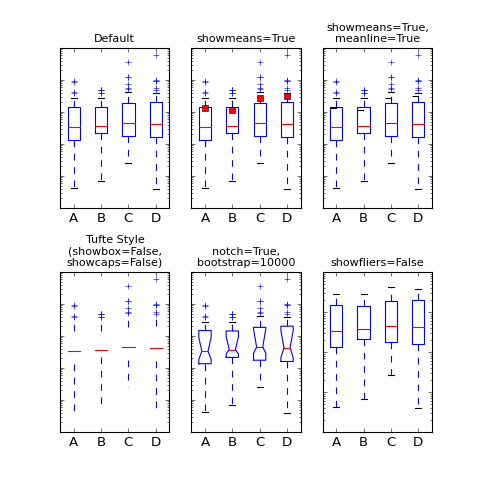(png, hires.png, pdf)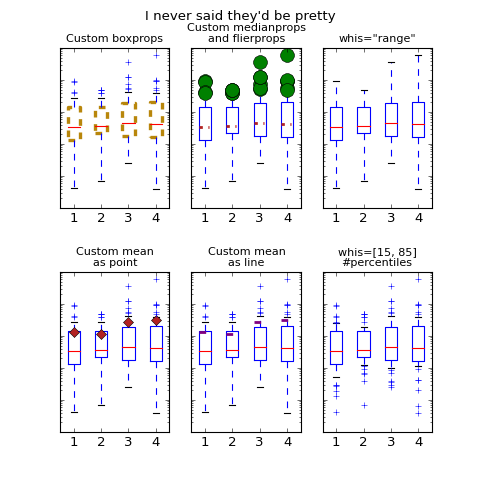`broken_barh`(xranges, yrange, **kwargs)

Plot horizontal bars.

Call signature:

```broken_barh(self, xranges, yrange, **kwargs)
```

A collection of horizontal bars spanning yrange with a sequence of xranges.

Required arguments:

Argument Description
xranges sequence of (xmin, xwidth)
yrange sequence of (ymin, ywidth)

kwargs are `matplotlib.collections.BrokenBarHCollection` properties:

Property Description
`agg_filter` unknown
`alpha` float or None
`animated` [True | False]
`antialiased` or antialiaseds Boolean or sequence of booleans
`array` unknown
`axes` an `Axes` instance
`clim` a length 2 sequence of floats
`clip_box` a `matplotlib.transforms.Bbox` instance
`clip_on` [True | False]
`clip_path` [ (`Path`, `Transform`) | `Patch` | None ]
`cmap` a colormap or registered colormap name
`color` matplotlib color arg or sequence of rgba tuples
`contains` a callable function
`edgecolor` or edgecolors matplotlib color arg or sequence of rgba tuples
`facecolor` or facecolors matplotlib color arg or sequence of rgba tuples
`figure` a `matplotlib.figure.Figure` instance
`gid` an id string
`hatch` [ ‘/’ | ‘\’ | ‘|’ | ‘-‘ | ‘+’ | ‘x’ | ‘o’ | ‘O’ | ‘.’ | ‘*’ ]
`label` string or anything printable with ‘%s’ conversion.
`linestyle` or linestyles or dashes [‘solid’ | ‘dashed’, ‘dashdot’, ‘dotted’ | (offset, on-off-dash-seq) ]
`linewidth` or lw or linewidths float or sequence of floats
`lod` [True | False]
`norm` unknown
`offset_position` unknown
`offsets` float or sequence of floats
`path_effects` unknown
`picker` [None|float|boolean|callable]
`pickradius` unknown
`rasterized` [True | False | None]
`sketch_params` unknown
`snap` unknown
`transform` `Transform` instance
`url` a url string
`urls` unknown
`visible` [True | False]
`zorder` any number

these can either be a single argument, ie:

```facecolors = 'black'
```

or a sequence of arguments for the various bars, ie:

```facecolors = ('black', 'red', 'green')
```

Example: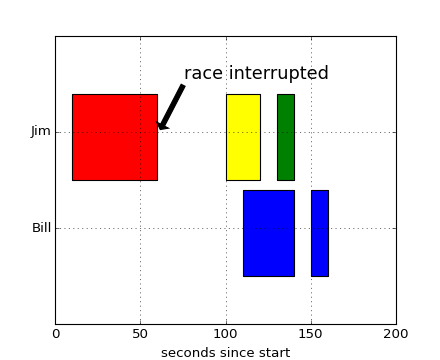`bxp`(bxpstats, positions=None, widths=None, vert=True, patch_artist=False, shownotches=False, showmeans=False, showcaps=True, showbox=True, showfliers=True, boxprops=None, whiskerprops=None, flierprops=None, medianprops=None, capprops=None, meanprops=None, meanline=False, manage_xticks=True)

Drawing function for box and whisker plots.

Call signature:

```bxp(self, bxpstats, positions=None, widths=None, vert=True,
patch_artist=False, shownotches=False, showmeans=False,
showcaps=True, showbox=True, showfliers=True,
boxprops=None, whiskerprops=None, flierprops=None,
medianprops=None, capprops=None, meanprops=None,
meanline=False, manage_xticks=True):
```

Make a box and whisker plot for each column of x or each vector in sequence x. The box extends from the lower to upper quartile values of the data, with a line at the median. The whiskers extend from the box to show the range of the data. Flier points are those past the end of the whiskers.

Parameters: bxpstats : list of dicts A list of dictionaries containing stats for each boxplot. Required keys are: `med`: The median (scalar float). `q1`: The first quartile (25th percentile) (scalar float). `q3`: The first quartile (50th percentile) (scalar float). `whislo`: Lower bound of the lower whisker (scalar float). `whishi`: Upper bound of the upper whisker (scalar float). Optional keys are: `mean`: The mean (scalar float). Needed if `showmeans=True`. `fliers`: Data beyond the whiskers (sequence of floats). Needed if `showfliers=True`. `cilo` & `ciho`: Lower and upper confidence intervals about the median. Needed if `shownotches=True`. `label`: Name of the dataset (string). If available, this will be used a tick label for the boxplot positions : array-like, default = [1, 2, ..., n] Sets the positions of the boxes. The ticks and limits are automatically set to match the positions. widths : array-like, default = 0.5 Either a scalar or a vector and sets the width of each box. The default is 0.5, or ```0.15*(distance between extreme positions)``` if that is smaller. vert : bool, default = False If `True` (default), makes the boxes vertical. If `False`, makes horizontal boxes. patch_artist : bool, default = False If `False` produces boxes with the `Line2D` artist. If `True` produces boxes with the `Patch` artist. shownotches : bool, default = False If `False` (default), produces a rectangular box plot. If `True`, will produce a notched box plot showmeans : bool, default = False If `True`, will toggle one the rendering of the means showcaps : bool, default = True If `True`, will toggle one the rendering of the caps showbox : bool, default = True If `True`, will toggle one the rendering of box showfliers : bool, default = True If `True`, will toggle one the rendering of the fliers boxprops : dict or None (default) If provided, will set the plotting style of the boxes whiskerprops : dict or None (default) If provided, will set the plotting style of the whiskers capprops : dict or None (default) If provided, will set the plotting style of the caps flierprops : dict or None (default) If provided will set the plotting style of the fliers medianprops : dict or None (default) If provided, will set the plotting style of the medians meanprops : dict or None (default) If provided, will set the plotting style of the means meanline : bool, default = False If `True` (and showmeans is `True`), will try to render the mean as a line spanning the full width of the box according to meanprops. Not recommended if shownotches is also True. Otherwise, means will be shown as points. manage_xticks : bool, default = True If the function should adjust the xlim and xtick locations. result : dict A dictionary mapping each component of the boxplot to a list of the `matplotlib.lines.Line2D` instances created. That dictionary has the following keys (assuming vertical boxplots): `boxes`: the main body of the boxplot showing the quartiles and the median’s confidence intervals if enabled. `medians`: horizonal lines at the median of each box. `whiskers`: the vertical lines extending to the most extreme, n-outlier data points. `caps`: the horizontal lines at the ends of the whiskers. `fliers`: points representing data that extend beyond the whiskers (fliers). `means`: points or lines representing the means.

Examples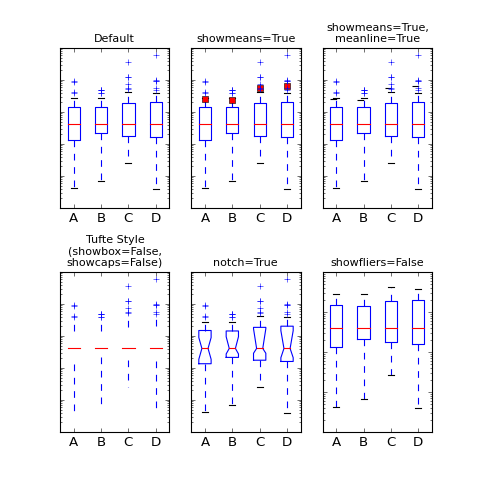(png, hires.png, pdf)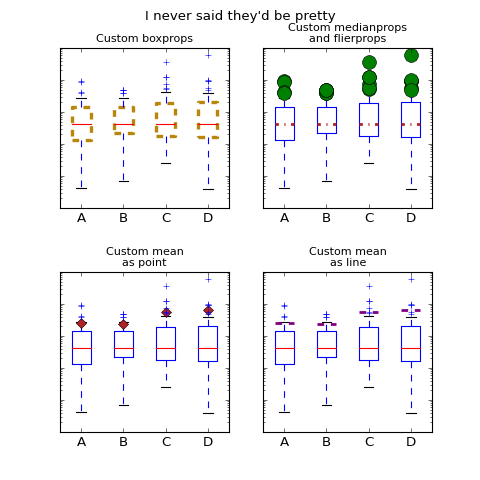`can_pan`()

Return True if this axes supports any pan/zoom button functionality.

`can_zoom`()

Return True if this axes supports the zoom box button functionality.

`cla`()

Clear the current axes.

`clabel`(CS, *args, **kwargs)

Label a contour plot.

Call signature:

```clabel(cs, **kwargs)
```

Adds labels to line contours in cs, where cs is a `ContourSet` object returned by contour.

```clabel(cs, v, **kwargs)
```

only labels contours listed in v.

Optional keyword arguments:

fontsize:
size in points or relative size eg ‘smaller’, ‘x-large’
colors:
• if None, the color of each label matches the color of the corresponding contour
• if one string color, e.g., colors = ‘r’ or colors = ‘red’, all labels will be plotted in this color
• if a tuple of matplotlib color args (string, float, rgb, etc), different labels will be plotted in different colors in the order specified
inline:
controls whether the underlying contour is removed or not. Default is True.
inline_spacing:
space in pixels to leave on each side of label when placing inline. Defaults to 5. This spacing will be exact for labels at locations where the contour is straight, less so for labels on curved contours.
fmt:
a format string for the label. Default is ‘%1.3f’ Alternatively, this can be a dictionary matching contour levels with arbitrary strings to use for each contour level (i.e., fmt[level]=string), or it can be any callable, such as a `Formatter` instance, that returns a string when called with a numeric contour level.
manual:

if True, contour labels will be placed manually using mouse clicks. Click the first button near a contour to add a label, click the second button (or potentially both mouse buttons at once) to finish adding labels. The third button can be used to remove the last label added, but only if labels are not inline. Alternatively, the keyboard can be used to select label locations (enter to end label placement, delete or backspace act like the third mouse button, and any other key will select a label location).

manual can be an iterable object of x,y tuples. Contour labels will be created as if mouse is clicked at each x,y positions.

rightside_up:
if True (default), label rotations will always be plus or minus 90 degrees from level.
use_clabeltext:
if True (default is False), ClabelText class (instead of matplotlib.Text) is used to create labels. ClabelText recalculates rotation angles of texts during the drawing time, therefore this can be used if aspect of the axes changes.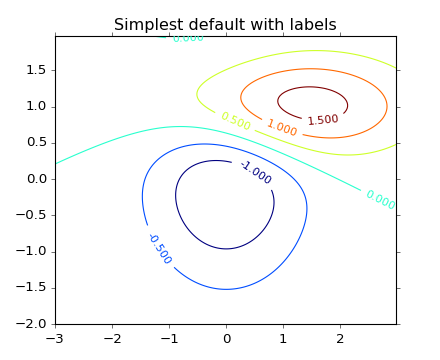(png, hires.png, pdf)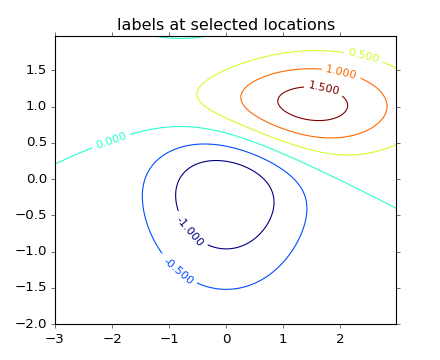(png, hires.png, pdf)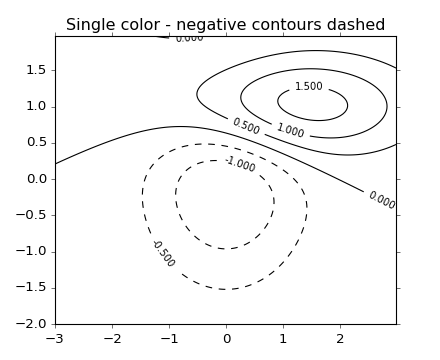(png, hires.png, pdf)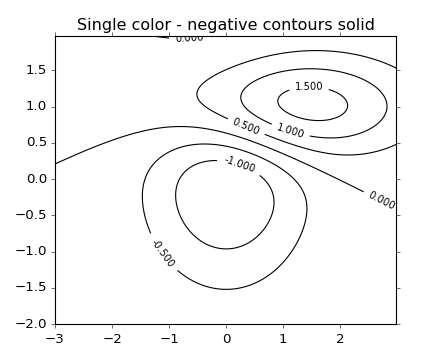(png, hires.png, pdf)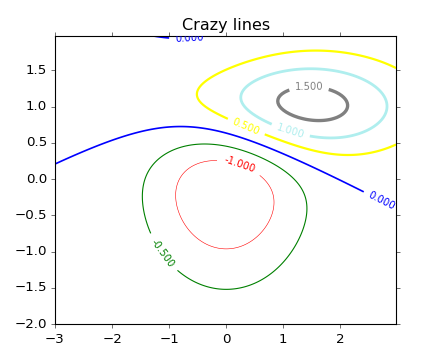(png, hires.png, pdf)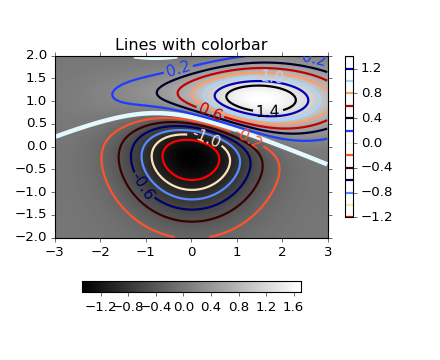(png, hires.png, pdf)

`clear`()

clear the axes

`cohere`(x, y, NFFT=256, Fs=2, Fc=0, detrend=<function detrend_none at 0x7fd543b15c08>, window=<function window_hanning at 0x7fd543b15938>, noverlap=0, pad_to=None, sides=u'default', scale_by_freq=None, **kwargs)

Plot the coherence between x and y.

Call signature:

```cohere(x, y, NFFT=256, Fs=2, Fc=0, detrend = mlab.detrend_none,
sides='default', scale_by_freq=None, **kwargs)
```

Plot the coherence between x and y. Coherence is the normalized cross spectral density: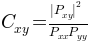Keyword arguments:

Fs: scalar
The sampling frequency (samples per time unit). It is used to calculate the Fourier frequencies, freqs, in cycles per time unit. The default value is 2.
window: callable or ndarray
A function or a vector of length NFFT. To create window vectors see `window_hanning()`, `window_none()`, `numpy.blackman()`, `numpy.hamming()`, `numpy.bartlett()`, `scipy.signal()`, `scipy.signal.get_window()`, etc. The default is `window_hanning()`. If a function is passed as the argument, it must take a data segment as an argument and return the windowed version of the segment.
sides: [ ‘default’ | ‘onesided’ | ‘twosided’ ]
Specifies which sides of the spectrum to return. Default gives the default behavior, which returns one-sided for real data and both for complex data. ‘onesided’ forces the return of a one-sided spectrum, while ‘twosided’ forces two-sided.
The number of points to which the data segment is padded when performing the FFT. This can be different from NFFT, which specifies the number of data points used. While not increasing the actual resolution of the spectrum (the minimum distance between resolvable peaks), this can give more points in the plot, allowing for more detail. This corresponds to the n parameter in the call to fft(). The default is None, which sets pad_to equal to NFFT
NFFT: integer
The number of data points used in each block for the FFT. A power 2 is most efficient. The default value is 256. This should NOT be used to get zero padding, or the scaling of the result will be incorrect. Use pad_to for this instead.
detrend: [ ‘default’ | ‘constant’ | ‘mean’ | ‘linear’ | ‘none’] or
callable

The function applied to each segment before fft-ing, designed to remove the mean or linear trend. Unlike in MATLAB, where the detrend parameter is a vector, in matplotlib is it a function. The `pylab` module defines `detrend_none()`, `detrend_mean()`, and `detrend_linear()`, but you can use a custom function as well. You can also use a string to choose one of the functions. ‘default’, ‘constant’, and ‘mean’ call `detrend_mean()`. ‘linear’ calls `detrend_linear()`. ‘none’ calls `detrend_none()`.

scale_by_freq: boolean
Specifies whether the resulting density values should be scaled by the scaling frequency, which gives density in units of Hz^-1. This allows for integration over the returned frequency values. The default is True for MATLAB compatibility.
noverlap: integer
The number of points of overlap between blocks. The default value is 0 (no overlap).
Fc: integer
The center frequency of x (defaults to 0), which offsets the x extents of the plot to reflect the frequency range used when a signal is acquired and then filtered and downsampled to baseband.

The return value is a tuple (Cxy, f), where f are the frequencies of the coherence vector.

kwargs are applied to the lines.

References:

• Bendat & Piersol – Random Data: Analysis and Measurement Procedures, John Wiley & Sons (1986)

kwargs control the `Line2D` properties of the coherence plot:

Property Description
`agg_filter` unknown
`alpha` float (0.0 transparent through 1.0 opaque)
`animated` [True | False]
`antialiased` or aa [True | False]
`axes` an `Axes` instance
`clip_box` a `matplotlib.transforms.Bbox` instance
`clip_on` [True | False]
`clip_path` [ (`Path`, `Transform`) | `Patch` | None ]
`color` or c any matplotlib color
`contains` a callable function
`dash_capstyle` [‘butt’ | ‘round’ | ‘projecting’]
`dash_joinstyle` [‘miter’ | ‘round’ | ‘bevel’]
`dashes` sequence of on/off ink in points
`drawstyle` [‘default’ | ‘steps’ | ‘steps-pre’ | ‘steps-mid’ | ‘steps-post’]
`figure` a `matplotlib.figure.Figure` instance
`fillstyle` [‘full’ | ‘left’ | ‘right’ | ‘bottom’ | ‘top’ | ‘none’]
`gid` an id string
`label` string or anything printable with ‘%s’ conversion.
`linestyle` or ls [`'-'` | `'--'` | `'-.'` | `':'` | `'None'` | `' '` | `''`]
`linewidth` or lw float value in points
`lod` [True | False]
`marker` unknown
`markeredgecolor` or mec any matplotlib color
`markeredgewidth` or mew float value in points
`markerfacecolor` or mfc any matplotlib color
`markerfacecoloralt` or mfcalt any matplotlib color
`markersize` or ms float
`markevery` unknown
`path_effects` unknown
`picker` float distance in points or callable pick function `fn(artist, event)`
`pickradius` float distance in points
`rasterized` [True | False | None]
`sketch_params` unknown
`snap` unknown
`solid_capstyle` [‘butt’ | ‘round’ | ‘projecting’]
`solid_joinstyle` [‘miter’ | ‘round’ | ‘bevel’]
`transform` a `matplotlib.transforms.Transform` instance
`url` a url string
`visible` [True | False]
`xdata` 1D array
`ydata` 1D array
`zorder` any number

Example: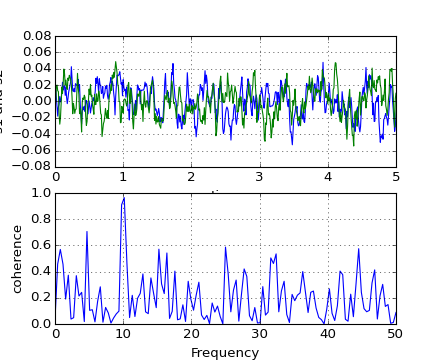`contains`(mouseevent)

Test whether the mouse event occured in the axes.

Returns True / False, {}

`contains_point`(point)

Returns True if the point (tuple of x,y) is inside the axes (the area defined by the its patch). A pixel coordinate is required.

`contour`(*args, **kwargs)

Plot contours.

`contour()` and `contourf()` draw contour lines and filled contours, respectively. Except as noted, function signatures and return values are the same for both versions.

`contourf()` differs from the MATLAB version in that it does not draw the polygon edges. To draw edges, add line contours with calls to `contour()`.

Call signatures:

```contour(Z)
```

make a contour plot of an array Z. The level values are chosen automatically.

```contour(X,Y,Z)
```

X, Y specify the (x, y) coordinates of the surface

```contour(Z,N)
contour(X,Y,Z,N)
```

contour N automatically-chosen levels.

```contour(Z,V)
contour(X,Y,Z,V)
```

draw contour lines at the values specified in sequence V

```contourf(..., V)
```

fill the `len(V)-1` regions between the values in V

```contour(Z, **kwargs)
```

Use keyword args to control colors, linewidth, origin, cmap ... see below for more details.

X and Y must both be 2-D with the same shape as Z, or they must both be 1-D such that `len(X)` is the number of columns in Z and `len(Y)` is the number of rows in Z.

`C = contour(...)` returns a `QuadContourSet` object.

Optional keyword arguments:

colors: [ None | string | (mpl_colors) ]

If None, the colormap specified by cmap will be used.

If a string, like ‘r’ or ‘red’, all levels will be plotted in this color.

If a tuple of matplotlib color args (string, float, rgb, etc), different levels will be plotted in different colors in the order specified.

alpha: float
The alpha blending value
cmap: [ None | Colormap ]
A cm `Colormap` instance or None. If cmap is None and colors is None, a default Colormap is used.
norm: [ None | Normalize ]
A `matplotlib.colors.Normalize` instance for scaling data values to colors. If norm is None and colors is None, the default linear scaling is used.
vmin, vmax: [ None | scalar ]
If not None, either or both of these values will be supplied to the `matplotlib.colors.Normalize` instance, overriding the default color scaling based on levels.
levels: [level0, level1, ..., leveln]
A list of floating point numbers indicating the level curves to draw; eg to draw just the zero contour pass `levels=`
origin: [ None | ‘upper’ | ‘lower’ | ‘image’ ]

If None, the first value of Z will correspond to the lower left corner, location (0,0). If ‘image’, the rc value for `image.origin` will be used.

This keyword is not active if X and Y are specified in the call to contour.

extent: [ None | (x0,x1,y0,y1) ]

If origin is not None, then extent is interpreted as in `matplotlib.pyplot.imshow()`: it gives the outer pixel boundaries. In this case, the position of Z[0,0] is the center of the pixel, not a corner. If origin is None, then (x0, y0) is the position of Z[0,0], and (x1, y1) is the position of Z[-1,-1].

This keyword is not active if X and Y are specified in the call to contour.

locator: [ None | ticker.Locator subclass ]
If locator is None, the default `MaxNLocator` is used. The locator is used to determine the contour levels if they are not given explicitly via the V argument.
extend: [ ‘neither’ | ‘both’ | ‘min’ | ‘max’ ]
Unless this is ‘neither’, contour levels are automatically added to one or both ends of the range so that all data are included. These added ranges are then mapped to the special colormap values which default to the ends of the colormap range, but can be set via `matplotlib.colors.Colormap.set_under()` and `matplotlib.colors.Colormap.set_over()` methods.
xunits, yunits: [ None | registered units ]
Override axis units by specifying an instance of a `matplotlib.units.ConversionInterface`.
antialiased: [ True | False ]
enable antialiasing, overriding the defaults. For filled contours, the default is True. For line contours, it is taken from rcParams[‘lines.antialiased’].

contour-only keyword arguments:

linewidths: [ None | number | tuple of numbers ]

If linewidths is None, the default width in `lines.linewidth` in `matplotlibrc` is used.

If a number, all levels will be plotted with this linewidth.

If a tuple, different levels will be plotted with different linewidths in the order specified.

linestyles: [ None | ‘solid’ | ‘dashed’ | ‘dashdot’ | ‘dotted’ ]

If linestyles is None, the default is ‘solid’ unless the lines are monochrome. In that case, negative contours will take their linestyle from the `matplotlibrc` `contour.negative_linestyle` setting.

linestyles can also be an iterable of the above strings specifying a set of linestyles to be used. If this iterable is shorter than the number of contour levels it will be repeated as necessary.

contourf-only keyword arguments:

nchunk: [ 0 | integer ]
If 0, no subdivision of the domain. Specify a positive integer to divide the domain into subdomains of roughly nchunk by nchunk points. This may never actually be advantageous, so this option may be removed. Chunking introduces artifacts at the chunk boundaries unless antialiased is False.
hatches:
A list of cross hatch patterns to use on the filled areas. If None, no hatching will be added to the contour. Hatching is supported in the PostScript, PDF, SVG and Agg backends only.

Note: contourf fills intervals that are closed at the top; that is, for boundaries z1 and z2, the filled region is:

```z1 < z <= z2
```

There is one exception: if the lowest boundary coincides with the minimum value of the z array, then that minimum value will be included in the lowest interval.

Examples:(png, hires.png, pdf)(png, hires.png, pdf)(png, hires.png, pdf)(png, hires.png, pdf)(png, hires.png, pdf)(png, hires.png, pdf)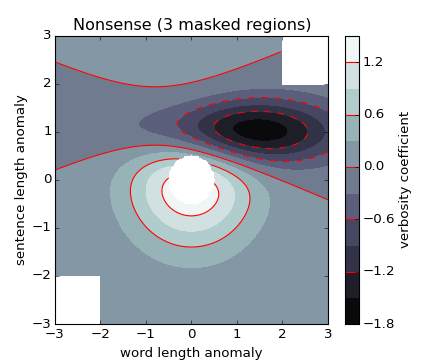(png, hires.png, pdf)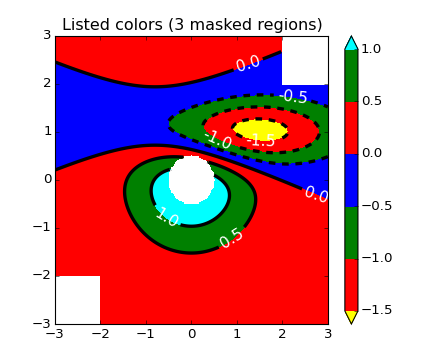(png, hires.png, pdf)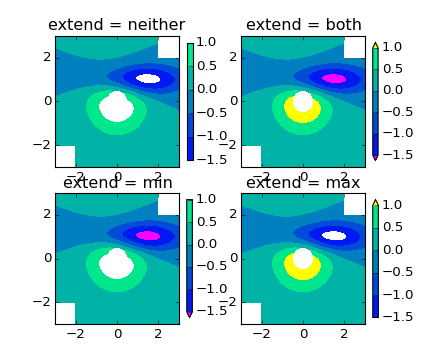(png, hires.png, pdf)

`contourf`(*args, **kwargs)

Plot contours.

`contour()` and `contourf()` draw contour lines and filled contours, respectively. Except as noted, function signatures and return values are the same for both versions.

`contourf()` differs from the MATLAB version in that it does not draw the polygon edges. To draw edges, add line contours with calls to `contour()`.

Call signatures:

```contour(Z)
```

make a contour plot of an array Z. The level values are chosen automatically.

```contour(X,Y,Z)
```

X, Y specify the (x, y) coordinates of the surface

```contour(Z,N)
contour(X,Y,Z,N)
```

contour N automatically-chosen levels.

```contour(Z,V)
contour(X,Y,Z,V)
```

draw contour lines at the values specified in sequence V

```contourf(..., V)
```

fill the `len(V)-1` regions between the values in V

```contour(Z, **kwargs)
```

Use keyword args to control colors, linewidth, origin, cmap ... see below for more details.

X and Y must both be 2-D with the same shape as Z, or they must both be 1-D such that `len(X)` is the number of columns in Z and `len(Y)` is the number of rows in Z.

`C = contour(...)` returns a `QuadContourSet` object.

Optional keyword arguments:

colors: [ None | string | (mpl_colors) ]

If None, the colormap specified by cmap will be used.

If a string, like ‘r’ or ‘red’, all levels will be plotted in this color.

If a tuple of matplotlib color args (string, float, rgb, etc), different levels will be plotted in different colors in the order specified.

alpha: float
The alpha blending value
cmap: [ None | Colormap ]
A cm `Colormap` instance or None. If cmap is None and colors is None, a default Colormap is used.
norm: [ None | Normalize ]
A `matplotlib.colors.Normalize` instance for scaling data values to colors. If norm is None and colors is None, the default linear scaling is used.
vmin, vmax: [ None | scalar ]
If not None, either or both of these values will be supplied to the `matplotlib.colors.Normalize` instance, overriding the default color scaling based on levels.
levels: [level0, level1, ..., leveln]
A list of floating point numbers indicating the level curves to draw; eg to draw just the zero contour pass `levels=`
origin: [ None | ‘upper’ | ‘lower’ | ‘image’ ]

If None, the first value of Z will correspond to the lower left corner, location (0,0). If ‘image’, the rc value for `image.origin` will be used.

This keyword is not active if X and Y are specified in the call to contour.

extent: [ None | (x0,x1,y0,y1) ]

If origin is not None, then extent is interpreted as in `matplotlib.pyplot.imshow()`: it gives the outer pixel boundaries. In this case, the position of Z[0,0] is the center of the pixel, not a corner. If origin is None, then (x0, y0) is the position of Z[0,0], and (x1, y1) is the position of Z[-1,-1].

This keyword is not active if X and Y are specified in the call to contour.

locator: [ None | ticker.Locator subclass ]
If locator is None, the default `MaxNLocator` is used. The locator is used to determine the contour levels if they are not given explicitly via the V argument.
extend: [ ‘neither’ | ‘both’ | ‘min’ | ‘max’ ]
Unless this is ‘neither’, contour levels are automatically added to one or both ends of the range so that all data are included. These added ranges are then mapped to the special colormap values which default to the ends of the colormap range, but can be set via `matplotlib.colors.Colormap.set_under()` and `matplotlib.colors.Colormap.set_over()` methods.
xunits, yunits: [ None | registered units ]
Override axis units by specifying an instance of a `matplotlib.units.ConversionInterface`.
antialiased: [ True | False ]
enable antialiasing, overriding the defaults. For filled contours, the default is True. For line contours, it is taken from rcParams[‘lines.antialiased’].

contour-only keyword arguments:

linewidths: [ None | number | tuple of numbers ]

If linewidths is None, the default width in `lines.linewidth` in `matplotlibrc` is used.

If a number, all levels will be plotted with this linewidth.

If a tuple, different levels will be plotted with different linewidths in the order specified.

linestyles: [ None | ‘solid’ | ‘dashed’ | ‘dashdot’ | ‘dotted’ ]

If linestyles is None, the default is ‘solid’ unless the lines are monochrome. In that case, negative contours will take their linestyle from the `matplotlibrc` `contour.negative_linestyle` setting.

linestyles can also be an iterable of the above strings specifying a set of linestyles to be used. If this iterable is shorter than the number of contour levels it will be repeated as necessary.

contourf-only keyword arguments:

nchunk: [ 0 | integer ]
If 0, no subdivision of the domain. Specify a positive integer to divide the domain into subdomains of roughly nchunk by nchunk points. This may never actually be advantageous, so this option may be removed. Chunking introduces artifacts at the chunk boundaries unless antialiased is False.
hatches:
A list of cross hatch patterns to use on the filled areas. If None, no hatching will be added to the contour. Hatching is supported in the PostScript, PDF, SVG and Agg backends only.

Note: contourf fills intervals that are closed at the top; that is, for boundaries z1 and z2, the filled region is:

```z1 < z <= z2
```

There is one exception: if the lowest boundary coincides with the minimum value of the z array, then that minimum value will be included in the lowest interval.

Examples:(png, hires.png, pdf)(png, hires.png, pdf)(png, hires.png, pdf)(png, hires.png, pdf)(png, hires.png, pdf)(png, hires.png, pdf)(png, hires.png, pdf)(png, hires.png, pdf)(png, hires.png, pdf)

`convert_xunits`(x)

For artists in an axes, if the xaxis has units support, convert x using xaxis unit type

`convert_yunits`(y)

For artists in an axes, if the yaxis has units support, convert y using yaxis unit type

`csd`(x, y, NFFT=None, Fs=None, Fc=None, detrend=None, window=None, noverlap=None, pad_to=None, sides=None, scale_by_freq=None, return_line=None, **kwargs)

Plot the cross-spectral density.

Call signature:

```csd(x, y, NFFT=256, Fs=2, Fc=0, detrend=mlab.detrend_none,
sides='default', scale_by_freq=None, return_line=None, **kwargs)
```

The cross spectral density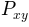by Welch’s average periodogram method. The vectors x and y are divided into NFFT length segments. Each segment is detrended by function detrend and windowed by function window. noverlap gives the length of the overlap between segments. The product of the direct FFTs of x and y are averaged over each segment to compute, with a scaling to correct for power loss due to windowing.

If len(x) < NFFT or len(y) < NFFT, they will be zero padded to NFFT.

x, y: 1-D arrays or sequences
Arrays or sequences containing the data

Keyword arguments:

Fs: scalar
The sampling frequency (samples per time unit). It is used to calculate the Fourier frequencies, freqs, in cycles per time unit. The default value is 2.
window: callable or ndarray
A function or a vector of length NFFT. To create window vectors see `window_hanning()`, `window_none()`, `numpy.blackman()`, `numpy.hamming()`, `numpy.bartlett()`, `scipy.signal()`, `scipy.signal.get_window()`, etc. The default is `window_hanning()`. If a function is passed as the argument, it must take a data segment as an argument and return the windowed version of the segment.
sides: [ ‘default’ | ‘onesided’ | ‘twosided’ ]
Specifies which sides of the spectrum to return. Default gives the default behavior, which returns one-sided for real data and both for complex data. ‘onesided’ forces the return of a one-sided spectrum, while ‘twosided’ forces two-sided.
The number of points to which the data segment is padded when performing the FFT. This can be different from NFFT, which specifies the number of data points used. While not increasing the actual resolution of the spectrum (the minimum distance between resolvable peaks), this can give more points in the plot, allowing for more detail. This corresponds to the n parameter in the call to fft(). The default is None, which sets pad_to equal to NFFT
NFFT: integer
The number of data points used in each block for the FFT. A power 2 is most efficient. The default value is 256. This should NOT be used to get zero padding, or the scaling of the result will be incorrect. Use pad_to for this instead.
detrend: [ ‘default’ | ‘constant’ | ‘mean’ | ‘linear’ | ‘none’] or
callable

The function applied to each segment before fft-ing, designed to remove the mean or linear trend. Unlike in MATLAB, where the detrend parameter is a vector, in matplotlib is it a function. The `pylab` module defines `detrend_none()`, `detrend_mean()`, and `detrend_linear()`, but you can use a custom function as well. You can also use a string to choose one of the functions. ‘default’, ‘constant’, and ‘mean’ call `detrend_mean()`. ‘linear’ calls `detrend_linear()`. ‘none’ calls `detrend_none()`.

scale_by_freq: boolean
Specifies whether the resulting density values should be scaled by the scaling frequency, which gives density in units of Hz^-1. This allows for integration over the returned frequency values. The default is True for MATLAB compatibility.
noverlap: integer
The number of points of overlap between segments. The default value is 0 (no overlap).
Fc: integer
The center frequency of x (defaults to 0), which offsets the x extents of the plot to reflect the frequency range used when a signal is acquired and then filtered and downsampled to baseband.
return_line: bool
Whether to include the line object plotted in the returned values. Default is False.

If return_line is False, returns the tuple (Pxy, freqs). If return_line is True, returns the tuple (Pxy, freqs. line):

Pxy: 1-D array
The values for the cross spectrum `P_{xy}` before scaling (complex valued)
freqs: 1-D array
The frequencies corresponding to the elements in Pxy
line: a `Line2D` instance
The line created by this function. Only returend if return_line is True.

For plotting, the power is plotted as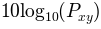for decibels, though `P_{xy}` itself is returned.

References:
Bendat & Piersol – Random Data: Analysis and Measurement Procedures, John Wiley & Sons (1986)

kwargs control the Line2D properties:

Property Description
`agg_filter` unknown
`alpha` float (0.0 transparent through 1.0 opaque)
`animated` [True | False]
`antialiased` or aa [True | False]
`axes` an `Axes` instance
`clip_box` a `matplotlib.transforms.Bbox` instance
`clip_on` [True | False]
`clip_path` [ (`Path`, `Transform`) | `Patch` | None ]
`color` or c any matplotlib color
`contains` a callable function
`dash_capstyle` [‘butt’ | ‘round’ | ‘projecting’]
`dash_joinstyle` [‘miter’ | ‘round’ | ‘bevel’]
`dashes` sequence of on/off ink in points
`drawstyle` [‘default’ | ‘steps’ | ‘steps-pre’ | ‘steps-mid’ | ‘steps-post’]
`figure` a `matplotlib.figure.Figure` instance
`fillstyle` [‘full’ | ‘left’ | ‘right’ | ‘bottom’ | ‘top’ | ‘none’]
`gid` an id string
`label` string or anything printable with ‘%s’ conversion.
`linestyle` or ls [`'-'` | `'--'` | `'-.'` | `':'` | `'None'` | `' '` | `''`]
`linewidth` or lw float value in points
`lod` [True | False]
`marker` unknown
`markeredgecolor` or mec any matplotlib color
`markeredgewidth` or mew float value in points
`markerfacecolor` or mfc any matplotlib color
`markerfacecoloralt` or mfcalt any matplotlib color
`markersize` or ms float
`markevery` unknown
`path_effects` unknown
`picker` float distance in points or callable pick function `fn(artist, event)`
`pickradius` float distance in points
`rasterized` [True | False | None]
`sketch_params` unknown
`snap` unknown
`solid_capstyle` [‘butt’ | ‘round’ | ‘projecting’]
`solid_joinstyle` [‘miter’ | ‘round’ | ‘bevel’]
`transform` a `matplotlib.transforms.Transform` instance
`url` a url string
`visible` [True | False]
`xdata` 1D array
`ydata` 1D array
`zorder` any number

Example: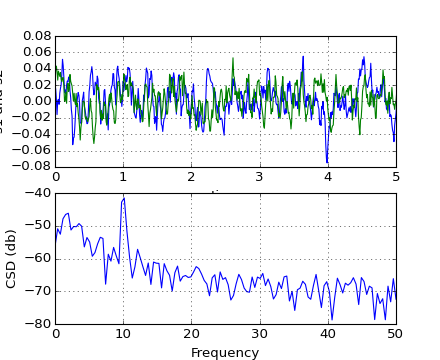`psd()`
`psd()` is the equivalent to setting y=x.
`drag_pan`(button, key, x, y)

Called when the mouse moves during a pan operation.

button is the mouse button number:

• 1: LEFT
• 2: MIDDLE
• 3: RIGHT

key is a “shift” key

x, y are the mouse coordinates in display coords.

Note

Intended to be overridden by new projection types.

`draw`(artist, renderer, *args, **kwargs)

Draw everything (plot lines, axes, labels)

`draw_artist`(a)

This method can only be used after an initial draw which caches the renderer. It is used to efficiently update Axes data (axis ticks, labels, etc are not updated)

`end_pan`()

Called when a pan operation completes (when the mouse button is up.)

Note

Intended to be overridden by new projection types.

`errorbar`(x, y, yerr=None, xerr=None, fmt=u'', ecolor=None, elinewidth=None, capsize=3, barsabove=False, lolims=False, uplims=False, xlolims=False, xuplims=False, errorevery=1, capthick=None, **kwargs)

Plot an errorbar graph.

Call signature:

```errorbar(x, y, yerr=None, xerr=None,
fmt='', ecolor=None, elinewidth=None, capsize=3,
barsabove=False, lolims=False, uplims=False,
xlolims=False, xuplims=False, errorevery=1,
capthick=None)
```

Plot x versus y with error deltas in yerr and xerr. Vertical errorbars are plotted if yerr is not None. Horizontal errorbars are plotted if xerr is not None.

x, y, xerr, and yerr can all be scalars, which plots a single error bar at x, y.

Optional keyword arguments:

xerr/yerr: [ scalar | N, Nx1, or 2xN array-like ]

If a scalar number, len(N) array-like object, or an Nx1 array-like object, errorbars are drawn at +/-value relative to the data.

If a sequence of shape 2xN, errorbars are drawn at -row1 and +row2 relative to the data.

fmt: [ ‘’ | ‘none’ | plot format string ]
The plot format symbol. If fmt is ‘none’ (case-insensitive), only the errorbars are plotted. This is used for adding errorbars to a bar plot, for example. Default is ‘’, an empty plot format string; properties are then identical to the defaults for `plot()`.
ecolor: [ None | mpl color ]
A matplotlib color arg which gives the color the errorbar lines; if None, use the color of the line connecting the markers.
elinewidth: scalar
The linewidth of the errorbar lines. If None, use the linewidth.
capsize: scalar
The length of the error bar caps in points
capthick: scalar
An alias kwarg to markeredgewidth (a.k.a. - mew). This setting is a more sensible name for the property that controls the thickness of the error bar cap in points. For backwards compatibility, if mew or markeredgewidth are given, then they will over-ride capthick. This may change in future releases.
barsabove: [ True | False ]
if True, will plot the errorbars above the plot symbols. Default is below.
lolims / uplims / xlolims / xuplims: [ False | True ]
These arguments can be used to indicate that a value gives only upper/lower limits. In that case a caret symbol is used to indicate this. lims-arguments may be of the same type as xerr and yerr. To use limits with inverted axes, `set_xlim()` or `set_ylim()` must be called before `errorbar()`.
errorevery: positive integer
subsamples the errorbars. e.g., if everyerror=5, errorbars for every 5-th datapoint will be plotted. The data plot itself still shows all data points.

All other keyword arguments are passed on to the plot command for the markers. For example, this code makes big red squares with thick green edges:

```x,y,yerr = rand(3,10)
errorbar(x, y, yerr, marker='s',
mfc='red', mec='green', ms=20, mew=4)
```

where mfc, mec, ms and mew are aliases for the longer property names, markerfacecolor, markeredgecolor, markersize and markeredgewith.

valid kwargs for the marker properties are

Property Description
`agg_filter` unknown
`alpha` float (0.0 transparent through 1.0 opaque)
`animated` [True | False]
`antialiased` or aa [True | False]
`axes` an `Axes` instance
`clip_box` a `matplotlib.transforms.Bbox` instance
`clip_on` [True | False]
`clip_path` [ (`Path`, `Transform`) | `Patch` | None ]
`color` or c any matplotlib color
`contains` a callable function
`dash_capstyle` [‘butt’ | ‘round’ | ‘projecting’]
`dash_joinstyle` [‘miter’ | ‘round’ | ‘bevel’]
`dashes` sequence of on/off ink in points
`drawstyle` [‘default’ | ‘steps’ | ‘steps-pre’ | ‘steps-mid’ | ‘steps-post’]
`figure` a `matplotlib.figure.Figure` instance
`fillstyle` [‘full’ | ‘left’ | ‘right’ | ‘bottom’ | ‘top’ | ‘none’]
`gid` an id string
`label` string or anything printable with ‘%s’ conversion.
`linestyle` or ls [`'-'` | `'--'` | `'-.'` | `':'` | `'None'` | `' '` | `''`]
`linewidth` or lw float value in points
`lod` [True | False]
`marker` unknown
`markeredgecolor` or mec any matplotlib color
`markeredgewidth` or mew float value in points
`markerfacecolor` or mfc any matplotlib color
`markerfacecoloralt` or mfcalt any matplotlib color
`markersize` or ms float
`markevery` unknown
`path_effects` unknown
`picker` float distance in points or callable pick function `fn(artist, event)`
`pickradius` float distance in points
`rasterized` [True | False | None]
`sketch_params` unknown
`snap` unknown
`solid_capstyle` [‘butt’ | ‘round’ | ‘projecting’]
`solid_joinstyle` [‘miter’ | ‘round’ | ‘bevel’]
`transform` a `matplotlib.transforms.Transform` instance
`url` a url string
`visible` [True | False]
`xdata` 1D array
`ydata` 1D array
`zorder` any number

Returns (plotline, caplines, barlinecols):

plotline: `Line2D` instance
x, y plot markers and/or line
caplines: list of error bar cap
`Line2D` instances
barlinecols: list of
`LineCollection` instances for the horizontal and vertical error ranges.

Example: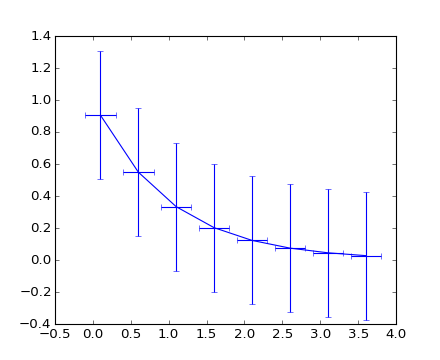`eventplot`(positions, orientation=u'horizontal', lineoffsets=1, linelengths=1, linewidths=None, colors=None, linestyles=u'solid', **kwargs)

Plot identical parallel lines at specific positions.

Call signature:

```eventplot(positions, orientation='horizontal', lineoffsets=0,
linelengths=1, linewidths=None, color =None,
linestyles='solid'
```

Plot parallel lines at the given positions. positions should be a 1D or 2D array-like object, with each row corresponding to a row or column of lines.

This type of plot is commonly used in neuroscience for representing neural events, where it is commonly called a spike raster, dot raster, or raster plot.

However, it is useful in any situation where you wish to show the timing or position of multiple sets of discrete events, such as the arrival times of people to a business on each day of the month or the date of hurricanes each year of the last century.

orientation : [ ‘horizonal’ | ‘vertical’ ]
‘horizonal’ : the lines will be vertical and arranged in rows “vertical’ : lines will be horizontal and arranged in columns
lineoffsets :
A float or array-like containing floats.
linelengths :
A float or array-like containing floats.
linewidths :
A float or array-like containing floats.
colors
must be a sequence of RGBA tuples (eg arbitrary color strings, etc, not allowed) or a list of such sequences
linestyles :
[ ‘solid’ | ‘dashed’ | ‘dashdot’ | ‘dotted’ ] or an array of these values

For linelengths, linewidths, colors, and linestyles, if only a single value is given, that value is applied to all lines. If an array-like is given, it must have the same length as positions, and each value will be applied to the corresponding row or column in positions.

Returns a list of `matplotlib.collections.EventCollection` objects that were added.

kwargs are `LineCollection` properties:

Property Description
`agg_filter` unknown
`alpha` float or None
`animated` [True | False]
`antialiased` or antialiaseds Boolean or sequence of booleans
`array` unknown
`axes` an `Axes` instance
`clim` a length 2 sequence of floats
`clip_box` a `matplotlib.transforms.Bbox` instance
`clip_on` [True | False]
`clip_path` [ (`Path`, `Transform`) | `Patch` | None ]
`cmap` a colormap or registered colormap name
`color` matplotlib color arg or sequence of rgba tuples
`contains` a callable function
`edgecolor` or edgecolors matplotlib color arg or sequence of rgba tuples
`facecolor` or facecolors matplotlib color arg or sequence of rgba tuples
`figure` a `matplotlib.figure.Figure` instance
`gid` an id string
`hatch` [ ‘/’ | ‘\’ | ‘|’ | ‘-‘ | ‘+’ | ‘x’ | ‘o’ | ‘O’ | ‘.’ | ‘*’ ]
`label` string or anything printable with ‘%s’ conversion.
`linestyle` or linestyles or dashes [‘solid’ | ‘dashed’, ‘dashdot’, ‘dotted’ | (offset, on-off-dash-seq) ]
`linewidth` or lw or linewidths float or sequence of floats
`lod` [True | False]
`norm` unknown
`offset_position` unknown
`offsets` float or sequence of floats
`path_effects` unknown
`paths` unknown
`picker` [None|float|boolean|callable]
`pickradius` unknown
`rasterized` [True | False | None]
`segments` unknown
`sketch_params` unknown
`snap` unknown
`transform` `Transform` instance
`url` a url string
`urls` unknown
`verts` unknown
`visible` [True | False]
`zorder` any number

Example: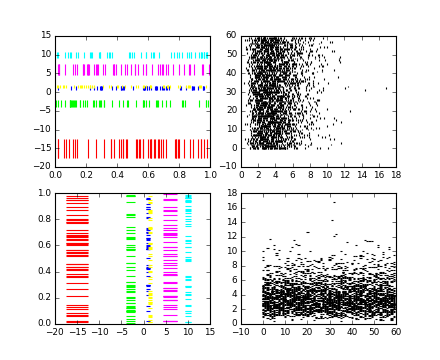`fill`(*args, **kwargs)

Plot filled polygons.

Call signature:

```fill(*args, **kwargs)
```

args is a variable length argument, allowing for multiple x, y pairs with an optional color format string; see `plot()` for details on the argument parsing. For example, to plot a polygon with vertices at x, y in blue.:

```ax.fill(x,y, 'b' )
```

An arbitrary number of x, y, color groups can be specified:

```ax.fill(x1, y1, 'g', x2, y2, 'r')
```

Return value is a list of `Patch` instances that were added.

The same color strings that `plot()` supports are supported by the fill format string.

If you would like to fill below a curve, e.g., shade a region between 0 and y along x, use `fill_between()`

The closed kwarg will close the polygon when True (default).

kwargs control the `Polygon` properties:

Property Description
`agg_filter` unknown
`alpha` float or None
`animated` [True | False]
`antialiased` or aa [True | False] or None for default
`axes` an `Axes` instance
`capstyle` [‘butt’ | ‘round’ | ‘projecting’]
`clip_box` a `matplotlib.transforms.Bbox` instance
`clip_on` [True | False]
`clip_path` [ (`Path`, `Transform`) | `Patch` | None ]
`color` matplotlib color spec
`contains` a callable function
`edgecolor` or ec mpl color spec, or None for default, or ‘none’ for no color
`facecolor` or fc mpl color spec, or None for default, or ‘none’ for no color
`figure` a `matplotlib.figure.Figure` instance
`fill` [True | False]
`gid` an id string
`hatch` [‘/’ | ‘\’ | ‘|’ | ‘-‘ | ‘+’ | ‘x’ | ‘o’ | ‘O’ | ‘.’ | ‘*’]
`joinstyle` [‘miter’ | ‘round’ | ‘bevel’]
`label` string or anything printable with ‘%s’ conversion.
`linestyle` or ls [‘solid’ | ‘dashed’ | ‘dashdot’ | ‘dotted’]
`linewidth` or lw float or None for default
`lod` [True | False]
`path_effects` unknown
`picker` [None|float|boolean|callable]
`rasterized` [True | False | None]
`sketch_params` unknown
`snap` unknown
`transform` `Transform` instance
`url` a url string
`visible` [True | False]
`zorder` any number

Example: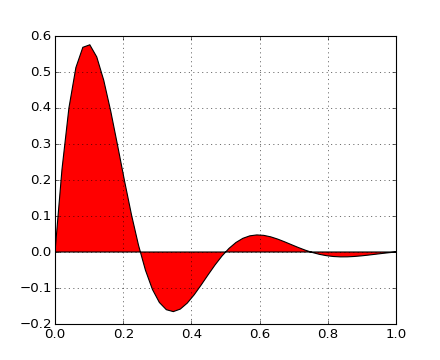`fill_between`(x, y1, y2=0, where=None, interpolate=False, **kwargs)

Make filled polygons between two curves.

Call signature:

```fill_between(x, y1, y2=0, where=None, **kwargs)
```

Create a `PolyCollection` filling the regions between y1 and y2 where `where==True`

x :
An N-length array of the x data
y1 :
An N-length array (or scalar) of the y data
y2 :
An N-length array (or scalar) of the y data
where :
If None, default to fill between everywhere. If not None, it is an N-length numpy boolean array and the fill will only happen over the regions where `where==True`.
interpolate :
If True, interpolate between the two lines to find the precise point of intersection. Otherwise, the start and end points of the filled region will only occur on explicit values in the x array.
kwargs :
Keyword args passed on to the `PolyCollection`.

kwargs control the `Polygon` properties:

Property Description
`agg_filter` unknown
`alpha` float or None
`animated` [True | False]
`antialiased` or antialiaseds Boolean or sequence of booleans
`array` unknown
`axes` an `Axes` instance
`clim` a length 2 sequence of floats
`clip_box` a `matplotlib.transforms.Bbox` instance
`clip_on` [True | False]
`clip_path` [ (`Path`, `Transform`) | `Patch` | None ]
`cmap` a colormap or registered colormap name
`color` matplotlib color arg or sequence of rgba tuples
`contains` a callable function
`edgecolor` or edgecolors matplotlib color arg or sequence of rgba tuples
`facecolor` or facecolors matplotlib color arg or sequence of rgba tuples
`figure` a `matplotlib.figure.Figure` instance
`gid` an id string
`hatch` [ ‘/’ | ‘\’ | ‘|’ | ‘-‘ | ‘+’ | ‘x’ | ‘o’ | ‘O’ | ‘.’ | ‘*’ ]
`label` string or anything printable with ‘%s’ conversion.
`linestyle` or linestyles or dashes [‘solid’ | ‘dashed’, ‘dashdot’, ‘dotted’ | (offset, on-off-dash-seq) ]
`linewidth` or lw or linewidths float or sequence of floats
`lod` [True | False]
`norm` unknown
`offset_position` unknown
`offsets` float or sequence of floats
`path_effects` unknown
`picker` [None|float|boolean|callable]
`pickradius` unknown
`rasterized` [True | False | None]
`sketch_params` unknown
`snap` unknown
`transform` `Transform` instance
`url` a url string
`urls` unknown
`visible` [True | False]
`zorder` any number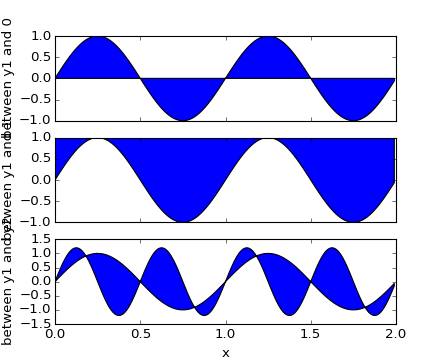(png, hires.png, pdf)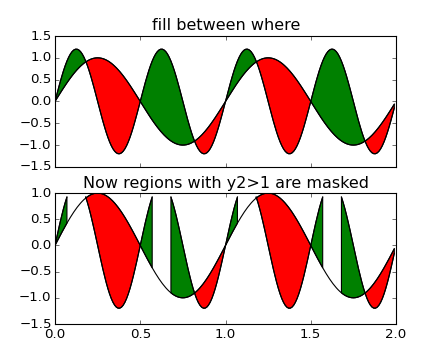(png, hires.png, pdf)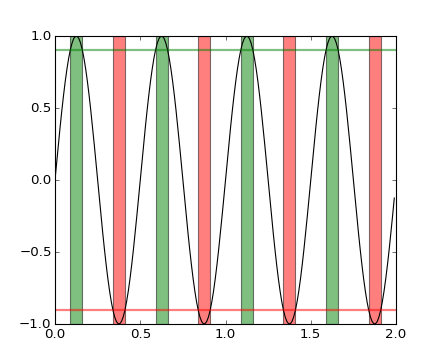(png, hires.png, pdf)

`fill_betweenx()`
for filling between two sets of x-values
`fill_betweenx`(y, x1, x2=0, where=None, **kwargs)

Make filled polygons between two horizontal curves.

Call signature:

```fill_betweenx(y, x1, x2=0, where=None, **kwargs)
```

Create a `PolyCollection` filling the regions between x1 and x2 where `where==True`

y :
An N-length array of the y data
x1 :
An N-length array (or scalar) of the x data
x2 :
An N-length array (or scalar) of the x data
where :
If None, default to fill between everywhere. If not None, it is a N length numpy boolean array and the fill will only happen over the regions where `where==True`
kwargs :
keyword args passed on to the `PolyCollection`

kwargs control the `Polygon` properties:

Property Description
`agg_filter` unknown
`alpha` float or None
`animated` [True | False]
`antialiased` or antialiaseds Boolean or sequence of booleans
`array` unknown
`axes` an `Axes` instance
`clim` a length 2 sequence of floats
`clip_box` a `matplotlib.transforms.Bbox` instance
`clip_on` [True | False]
`clip_path` [ (`Path`, `Transform`) | `Patch` | None ]
`cmap` a colormap or registered colormap name
`color` matplotlib color arg or sequence of rgba tuples
`contains` a callable function
`edgecolor` or edgecolors matplotlib color arg or sequence of rgba tuples
`facecolor` or facecolors matplotlib color arg or sequence of rgba tuples
`figure` a `matplotlib.figure.Figure` instance
`gid` an id string
`hatch` [ ‘/’ | ‘\’ | ‘|’ | ‘-‘ | ‘+’ | ‘x’ | ‘o’ | ‘O’ | ‘.’ | ‘*’ ]
`label` string or anything printable with ‘%s’ conversion.
`linestyle` or linestyles or dashes [‘solid’ | ‘dashed’, ‘dashdot’, ‘dotted’ | (offset, on-off-dash-seq) ]
`linewidth` or lw or linewidths float or sequence of floats
`lod` [True | False]
`norm` unknown
`offset_position` unknown
`offsets` float or sequence of floats
`path_effects` unknown
`picker` [None|float|boolean|callable]
`pickradius` unknown
`rasterized` [True | False | None]
`sketch_params` unknown
`snap` unknown
`transform` `Transform` instance
`url` a url string
`urls` unknown
`visible` [True | False]
`zorder` any number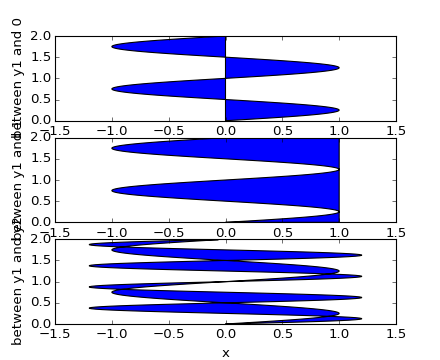(png, hires.png, pdf)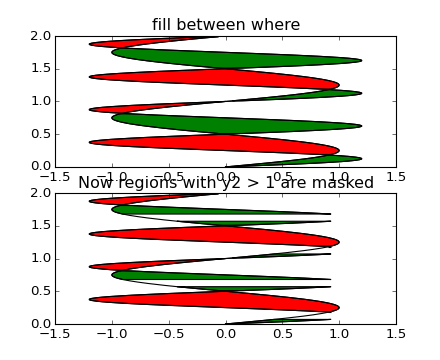(png, hires.png, pdf)

`fill_between()`
for filling between two sets of y-values
`findobj`(match=None, include_self=True)

Find artist objects.

Recursively find all `Artist` instances contained in self.

match can be

• None: return all objects contained in artist.
• function with signature `boolean = match(artist)` used to filter matches
• class instance: e.g., Line2D. Only return artists of class type.

If include_self is True (default), include self in the list to be checked for a match.

`format_coord`(x, y)

Return a format string formatting the x, y coord

`format_xdata`(x)

Return x string formatted. This function will use the attribute self.fmt_xdata if it is callable, else will fall back on the xaxis major formatter

`format_ydata`(y)

Return y string formatted. This function will use the `fmt_ydata` attribute if it is callable, else will fall back on the yaxis major formatter

`get_adjustable`()
`get_agg_filter`()

return filter function to be used for agg filter

`get_alpha`()

Return the alpha value used for blending - not supported on all backends

`get_anchor`()
`get_animated`()

Return the artist’s animated state

`get_aspect`()
`get_autoscale_on`()

Get whether autoscaling is applied for both axes on plot commands

`get_autoscalex_on`()

Get whether autoscaling for the x-axis is applied on plot commands

`get_autoscaley_on`()

Get whether autoscaling for the y-axis is applied on plot commands

`get_axes`()

Return the `Axes` instance the artist resides in, or None

`get_axes_locator`()

return axes_locator

`get_axis_bgcolor`()

Return the axis background color

`get_axisbelow`()

Get whether axis below is true or not

`get_children`()

return a list of child artists

`get_clip_box`()

Return artist clipbox

`get_clip_on`()

Return whether artist uses clipping

`get_clip_path`()

Return artist clip path

`get_contains`()

Return the _contains test used by the artist, or None for default.

`get_cursor_props`()

Return the cursor propertiess as a (linewidth, color) tuple, where linewidth is a float and color is an RGBA tuple

`get_data_ratio`()

Returns the aspect ratio of the raw data.

This method is intended to be overridden by new projection types.

`get_data_ratio_log`()

Returns the aspect ratio of the raw data in log scale. Will be used when both axis scales are in log.

`get_default_bbox_extra_artists`()
`get_figure`()

Return the `Figure` instance the artist belongs to.

`get_frame_on`()

Get whether the axes rectangle patch is drawn

`get_gid`()

Returns the group id

`get_images`()

return a list of Axes images contained by the Axes

`get_label`()

Get the label used for this artist in the legend.

`get_legend`()

Return the legend.Legend instance, or None if no legend is defined

`get_legend_handles_labels`(legend_handler_map=None)

Return handles and labels for legend

`ax.legend()` is equivalent to

```h, l = ax.get_legend_handles_labels()
ax.legend(h, l)
```
`get_lines`()

Return a list of lines contained by the Axes

`get_navigate`()

Get whether the axes responds to navigation commands

`get_navigate_mode`()

Get the navigation toolbar button status: ‘PAN’, ‘ZOOM’, or None

`get_path_effects`()
`get_picker`()

Return the picker object used by this artist

`get_position`(original=False)

Return the a copy of the axes rectangle as a Bbox

`get_rasterization_zorder`()

Get zorder value below which artists will be rasterized

`get_rasterized`()

return True if the artist is to be rasterized

`get_renderer_cache`()
`get_shared_x_axes`()

Return a copy of the shared axes Grouper object for x axes

`get_shared_y_axes`()

Return a copy of the shared axes Grouper object for y axes

`get_sketch_params`()

Returns the sketch parameters for the artist.

Returns: sketch_params : tuple or `None` A 3-tuple with the following elements: : `scale`: The amplitude of the wiggle perpendicular to the source line. `length`: The length of the wiggle along the line. `randomness`: The scale factor by which the length is shrunken or expanded. May return `None` if no sketch parameters were set. :
`get_snap`()

Returns the snap setting which may be:

• True: snap vertices to the nearest pixel center
• False: leave vertices as-is
• None: (auto) If the path contains only rectilinear line segments, round to the nearest pixel center

Only supported by the Agg and MacOSX backends.

`get_tightbbox`(renderer, call_axes_locator=True)

Return the tight bounding box of the axes. The dimension of the Bbox in canvas coordinate.

If call_axes_locator is False, it does not call the _axes_locator attribute, which is necessary to get the correct bounding box. `call_axes_locator==False` can be used if the caller is only intereted in the relative size of the tightbbox compared to the axes bbox.

`get_title`(loc=u'center')

Get an axes title.

Get one of the three available axes titles. The available titles are positioned above the axes in the center, flush with the left edge, and flush with the right edge.

Parameters: loc : {‘center’, ‘left’, ‘right’}, str, optional Which title to get, defaults to ‘center’ title: str : The title text string.
`get_transform`()

Return the `Transform` instance used by this artist.

`get_transformed_clip_path_and_affine`()

Return the clip path with the non-affine part of its transformation applied, and the remaining affine part of its transformation.

`get_url`()

Returns the url

`get_visible`()

Return the artist’s visiblity

`get_window_extent`(*args, **kwargs)

get the axes bounding box in display space; args and kwargs are empty

`get_xaxis`()

Return the XAxis instance

`get_xaxis_text1_transform`(pad_points)

Get the transformation used for drawing x-axis labels, which will add the given amount of padding (in points) between the axes and the label. The x-direction is in data coordinates and the y-direction is in axis coordinates. Returns a 3-tuple of the form:

```(transform, valign, halign)
```

where valign and halign are requested alignments for the text.

Note

This transformation is primarily used by the `Axis` class, and is meant to be overridden by new kinds of projections that may need to place axis elements in different locations.

`get_xaxis_text2_transform`(pad_points)

Get the transformation used for drawing the secondary x-axis labels, which will add the given amount of padding (in points) between the axes and the label. The x-direction is in data coordinates and the y-direction is in axis coordinates. Returns a 3-tuple of the form:

```(transform, valign, halign)
```

where valign and halign are requested alignments for the text.

Note

This transformation is primarily used by the `Axis` class, and is meant to be overridden by new kinds of projections that may need to place axis elements in different locations.

`get_xaxis_transform`(which=u'grid')

Get the transformation used for drawing x-axis labels, ticks and gridlines. The x-direction is in data coordinates and the y-direction is in axis coordinates.

Note

This transformation is primarily used by the `Axis` class, and is meant to be overridden by new kinds of projections that may need to place axis elements in different locations.

`get_xbound`()

Returns the x-axis numerical bounds where:

```lowerBound < upperBound
```
`get_xgridlines`()

Get the x grid lines as a list of Line2D instances

`get_xlabel`()

Get the xlabel text string.

`get_xlim`()

Get the x-axis range [left, right]

`get_xmajorticklabels`()

Get the xtick labels as a list of `Text` instances.

`get_xminorticklabels`()

Get the x minor tick labels as a list of `matplotlib.text.Text` instances.

`get_xscale`()

Return the xaxis scale string: linear, log, symlog

`get_xticklabels`(minor=False, which=None)

Get the x tick labels as a list of `Text` instances.

Parameters: minor : bool If True return the minor ticklabels, else return the major ticklabels which : None, (‘minor’, ‘major’, ‘both’) Overrides `minor`. Selects which ticklabels to return ret : list List of `Text` instances.
`get_xticklines`()

Get the xtick lines as a list of Line2D instances

`get_xticks`(minor=False)

Return the x ticks as a list of locations

`get_yaxis`()

Return the YAxis instance

`get_yaxis_text1_transform`(pad_points)

Get the transformation used for drawing y-axis labels, which will add the given amount of padding (in points) between the axes and the label. The x-direction is in axis coordinates and the y-direction is in data coordinates. Returns a 3-tuple of the form:

```(transform, valign, halign)
```

where valign and halign are requested alignments for the text.

Note

This transformation is primarily used by the `Axis` class, and is meant to be overridden by new kinds of projections that may need to place axis elements in different locations.

`get_yaxis_text2_transform`(pad_points)

Get the transformation used for drawing the secondary y-axis labels, which will add the given amount of padding (in points) between the axes and the label. The x-direction is in axis coordinates and the y-direction is in data coordinates. Returns a 3-tuple of the form:

```(transform, valign, halign)
```

where valign and halign are requested alignments for the text.

Note

This transformation is primarily used by the `Axis` class, and is meant to be overridden by new kinds of projections that may need to place axis elements in different locations.

`get_yaxis_transform`(which=u'grid')

Get the transformation used for drawing y-axis labels, ticks and gridlines. The x-direction is in axis coordinates and the y-direction is in data coordinates.

Note

This transformation is primarily used by the `Axis` class, and is meant to be overridden by new kinds of projections that may need to place axis elements in different locations.

`get_ybound`()

Return y-axis numerical bounds in the form of `lowerBound < upperBound`

`get_ygridlines`()

Get the y grid lines as a list of Line2D instances

`get_ylabel`()

Get the ylabel text string.

`get_ylim`()

Get the y-axis range [bottom, top]

`get_ymajorticklabels`()

Get the major y tick labels as a list of `Text` instances.

`get_yminorticklabels`()

Get the minor y tick labels as a list of `Text` instances.

`get_yscale`()

Return the yaxis scale string: linear, log, symlog

`get_yticklabels`(minor=False, which=None)

Get the x tick labels as a list of `Text` instances.

Parameters: minor : bool If True return the minor ticklabels, else return the major ticklabels which : None, (‘minor’, ‘major’, ‘both’) Overrides `minor`. Selects which ticklabels to return ret : list List of `Text` instances.
`get_yticklines`()

Get the ytick lines as a list of Line2D instances

`get_yticks`(minor=False)

Return the y ticks as a list of locations

`get_zorder`()

Return the `Artist`‘s zorder.

`grid`(b=None, which=u'major', axis=u'both', **kwargs)

Turn the axes grids on or off.

Call signature:

```grid(self, b=None, which='major', axis='both', **kwargs)
```

Set the axes grids on or off; b is a boolean. (For MATLAB compatibility, b may also be a string, ‘on’ or ‘off’.)

If b is None and `len(kwargs)==0`, toggle the grid state. If kwargs are supplied, it is assumed that you want a grid and b is thus set to True.

which can be ‘major’ (default), ‘minor’, or ‘both’ to control whether major tick grids, minor tick grids, or both are affected.

axis can be ‘both’ (default), ‘x’, or ‘y’ to control which set of gridlines are drawn.

kwargs are used to set the grid line properties, eg:

```ax.grid(color='r', linestyle='-', linewidth=2)
```

Valid `Line2D` kwargs are

Property Description
`agg_filter` unknown
`alpha` float (0.0 transparent through 1.0 opaque)
`animated` [True | False]
`antialiased` or aa [True | False]
`axes` an `Axes` instance
`clip_box` a `matplotlib.transforms.Bbox` instance
`clip_on` [True | False]
`clip_path` [ (`Path`, `Transform`) | `Patch` | None ]
`color` or c any matplotlib color
`contains` a callable function
`dash_capstyle` [‘butt’ | ‘round’ | ‘projecting’]
`dash_joinstyle` [‘miter’ | ‘round’ | ‘bevel’]
`dashes` sequence of on/off ink in points
`drawstyle` [‘default’ | ‘steps’ | ‘steps-pre’ | ‘steps-mid’ | ‘steps-post’]
`figure` a `matplotlib.figure.Figure` instance
`fillstyle` [‘full’ | ‘left’ | ‘right’ | ‘bottom’ | ‘top’ | ‘none’]
`gid` an id string
`label` string or anything printable with ‘%s’ conversion.
`linestyle` or ls [`'-'` | `'--'` | `'-.'` | `':'` | `'None'` | `' '` | `''`]
`linewidth` or lw float value in points
`lod` [True | False]
`marker` unknown
`markeredgecolor` or mec any matplotlib color
`markeredgewidth` or mew float value in points
`markerfacecolor` or mfc any matplotlib color
`markerfacecoloralt` or mfcalt any matplotlib color
`markersize` or ms float
`markevery` unknown
`path_effects` unknown
`picker` float distance in points or callable pick function `fn(artist, event)`
`pickradius` float distance in points
`rasterized` [True | False | None]
`sketch_params` unknown
`snap` unknown
`solid_capstyle` [‘butt’ | ‘round’ | ‘projecting’]
`solid_joinstyle` [‘miter’ | ‘round’ | ‘bevel’]
`transform` a `matplotlib.transforms.Transform` instance
`url` a url string
`visible` [True | False]
`xdata` 1D array
`ydata` 1D array
`zorder` any number
`has_data`()

Return True if any artists have been added to axes.

This should not be used to determine whether the dataLim need to be updated, and may not actually be useful for anything.

`have_units`()

Return True if units are set on the x or y axes

`hexbin`(x, y, C=None, gridsize=100, bins=None, xscale=u'linear', yscale=u'linear', extent=None, cmap=None, norm=None, vmin=None, vmax=None, alpha=None, linewidths=None, edgecolors=u'none', reduce_C_function=<function mean at 0x7fd5471666e0>, mincnt=None, marginals=False, **kwargs)

Make a hexagonal binning plot.

Call signature:

```hexbin(x, y, C = None, gridsize = 100, bins = None,
xscale = 'linear', yscale = 'linear',
cmap=None, norm=None, vmin=None, vmax=None,
alpha=None, linewidths=None, edgecolors='none'
reduce_C_function = np.mean, mincnt=None, marginals=True
**kwargs)
```

Make a hexagonal binning plot of x versus y, where x, y are 1-D sequences of the same length, N. If C is None (the default), this is a histogram of the number of occurences of the observations at (x[i],y[i]).

If C is specified, it specifies values at the coordinate (x[i],y[i]). These values are accumulated for each hexagonal bin and then reduced according to reduce_C_function, which defaults to numpy’s mean function (np.mean). (If C is specified, it must also be a 1-D sequence of the same length as x and y.)

x, y and/or C may be masked arrays, in which case only unmasked points will be plotted.

Optional keyword arguments:

gridsize: [ 100 | integer ]
The number of hexagons in the x-direction, default is 100. The corresponding number of hexagons in the y-direction is chosen such that the hexagons are approximately regular. Alternatively, gridsize can be a tuple with two elements specifying the number of hexagons in the x-direction and the y-direction.
bins: [ None | ‘log’ | integer | sequence ]

If None, no binning is applied; the color of each hexagon directly corresponds to its count value.

If ‘log’, use a logarithmic scale for the color map. Internally,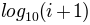is used to determine the hexagon color.

If an integer, divide the counts in the specified number of bins, and color the hexagons accordingly.

If a sequence of values, the values of the lower bound of the bins to be used.

xscale: [ ‘linear’ | ‘log’ ]
Use a linear or log10 scale on the horizontal axis.
scale: [ ‘linear’ | ‘log’ ]
Use a linear or log10 scale on the vertical axis.
mincnt: [ None | a positive integer ]
If not None, only display cells with more than mincnt number of points in the cell
marginals: [ True | False ]
if marginals is True, plot the marginal density as colormapped rectagles along the bottom of the x-axis and left of the y-axis
extent: [ None | scalars (left, right, bottom, top) ]
The limits of the bins. The default assigns the limits based on gridsize, x, y, xscale and yscale.

Other keyword arguments controlling color mapping and normalization arguments:

cmap: [ None | Colormap ]
a `matplotlib.colors.Colormap` instance. If None, defaults to rc `image.cmap`.
norm: [ None | Normalize ]
`matplotlib.colors.Normalize` instance is used to scale luminance data to 0,1.
vmin / vmax: scalar
vmin and vmax are used in conjunction with norm to normalize luminance data. If either are None, the min and max of the color array C is used. Note if you pass a norm instance, your settings for vmin and vmax will be ignored.
alpha: scalar between 0 and 1, or None
the alpha value for the patches
linewidths: [ None | scalar ]
If None, defaults to rc lines.linewidth. Note that this is a tuple, and if you set the linewidths argument you must set it as a sequence of floats, as required by `RegularPolyCollection`.

Other keyword arguments controlling the Collection properties:

edgecolors: [ None | `'none'` | mpl color | color sequence ]

If `'none'`, draws the edges in the same color as the fill color. This is the default, as it avoids unsightly unpainted pixels between the hexagons.

If None, draws the outlines in the default color.

If a matplotlib color arg or sequence of rgba tuples, draws the outlines in the specified color.

Here are the standard descriptions of all the `Collection` kwargs:

Property Description
`agg_filter` unknown
`alpha` float or None
`animated` [True | False]
`antialiased` or antialiaseds Boolean or sequence of booleans
`array` unknown
`axes` an `Axes` instance
`clim` a length 2 sequence of floats
`clip_box` a `matplotlib.transforms.Bbox` instance
`clip_on` [True | False]
`clip_path` [ (`Path`, `Transform`) | `Patch` | None ]
`cmap` a colormap or registered colormap name
`color` matplotlib color arg or sequence of rgba tuples
`contains` a callable function
`edgecolor` or edgecolors matplotlib color arg or sequence of rgba tuples
`facecolor` or facecolors matplotlib color arg or sequence of rgba tuples
`figure` a `matplotlib.figure.Figure` instance
`gid` an id string
`hatch` [ ‘/’ | ‘\’ | ‘|’ | ‘-‘ | ‘+’ | ‘x’ | ‘o’ | ‘O’ | ‘.’ | ‘*’ ]
`label` string or anything printable with ‘%s’ conversion.
`linestyle` or linestyles or dashes [‘solid’ | ‘dashed’, ‘dashdot’, ‘dotted’ | (offset, on-off-dash-seq) ]
`linewidth` or lw or linewidths float or sequence of floats
`lod` [True | False]
`norm` unknown
`offset_position` unknown
`offsets` float or sequence of floats
`path_effects` unknown
`picker` [None|float|boolean|callable]
`pickradius` unknown
`rasterized` [True | False | None]
`sketch_params` unknown
`snap` unknown
`transform` `Transform` instance
`url` a url string
`urls` unknown
`visible` [True | False]
`zorder` any number

The return value is a `PolyCollection` instance; use `get_array()` on this `PolyCollection` to get the counts in each hexagon. If marginals is True, horizontal bar and vertical bar (both PolyCollections) will be attached to the return collection as attributes hbar and vbar.

Example: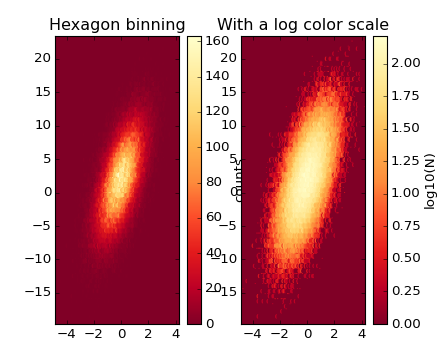`hist`(x, bins=10, range=None, normed=False, weights=None, cumulative=False, bottom=None, histtype=u'bar', align=u'mid', orientation=u'vertical', rwidth=None, log=False, color=None, label=None, stacked=False, **kwargs)

Plot a histogram.

Compute and draw the histogram of x. The return value is a tuple (n, bins, patches) or ([n0, n1, ...], bins, [patches0, patches1,...]) if the input contains multiple data.

Multiple data can be provided via x as a list of datasets of potentially different length ([x0, x1, ...]), or as a 2-D ndarray in which each column is a dataset. Note that the ndarray form is transposed relative to the list form.

Masked arrays are not supported at present.

Parameters: Returns: x : (n,) array or sequence of (n,) arrays Input values, this takes either a single array or a sequency of arrays which are not required to be of the same length bins : integer or array_like, optional, default: 10 If an integer is given, `bins + 1` bin edges are returned, consistently with `numpy.histogram()` for numpy version >= 1.3. Unequally spaced bins are supported if `bins` is a sequence. range : tuple, optional, default: None The lower and upper range of the bins. Lower and upper outliers are ignored. If not provided, `range` is (x.min(), x.max()). Range has no effect if `bins` is a sequence. If `bins` is a sequence or `range` is specified, autoscaling is based on the specified bin range instead of the range of x. normed : boolean, optional, default: False If `True`, the first element of the return tuple will be the counts normalized to form a probability density, i.e., `n/(len(x)`dbin)`, ie the integral of the histogram will sum to 1. If stacked is also True, the sum of the histograms is normalized to 1. weights : array_like, shape (n, ), optional, default: None An array of weights, of the same shape as `x`. Each value in `x` only contributes its associated weight towards the bin count (instead of 1). If `normed` is True, the weights are normalized, so that the integral of the density over the range remains 1. cumulative : boolean, optional, default If `True`, then a histogram is computed where each bin gives the counts in that bin plus all bins for smaller values. The last bin gives the total number of datapoints. If `normed` is also `True` then the histogram is normalized such that the last bin equals 1. If `cumulative` evaluates to less than 0 (e.g., -1), the direction of accumulation is reversed. In this case, if `normed` is also `True`, then the histogram is normalized such that the first bin equals 1. bottom : array_like, scalar, or None, default: None Location of the bottom baseline of each bin. If a scalar, the base line for each bin is shifted by the same amount. If an array, each bin is shifted independently and the length of bottom must match the number of bins. If None, defaults to 0. histtype : [‘bar’ | ‘barstacked’ | ‘step’ | ‘stepfilled’], optional The type of histogram to draw. ‘bar’ is a traditional bar-type histogram. If multiple data are given the bars are aranged side by side. ‘barstacked’ is a bar-type histogram where multiple data are stacked on top of each other. ‘step’ generates a lineplot that is by default unfilled. ‘stepfilled’ generates a lineplot that is by default filled. align : [‘left’ | ‘mid’ | ‘right’], optional, default: ‘mid’ Controls how the histogram is plotted. ‘left’: bars are centered on the left bin edges. ‘mid’: bars are centered between the bin edges. ‘right’: bars are centered on the right bin edges. orientation : [‘horizontal’ | ‘vertical’], optional If ‘horizontal’, `barh` will be used for bar-type histograms and the bottom kwarg will be the left edges. rwidth : scalar, optional, default: None The relative width of the bars as a fraction of the bin width. If `None`, automatically compute the width. Ignored if `histtype` = ‘step’ or ‘stepfilled’. log : boolean, optional, default If `True`, the histogram axis will be set to a log scale. If `log` is `True` and `x` is a 1D array, empty bins will be filtered out and only the non-empty (`n`, `bins`, `patches`) will be returned. color : color or array_like of colors, optional, default: None Color spec or sequence of color specs, one per dataset. Default (`None`) uses the standard line color sequence. label : string, optional, default: ‘’ String, or sequence of strings to match multiple datasets. Bar charts yield multiple patches per dataset, but only the first gets the label, so that the legend command will work as expected. stacked : boolean, optional, default If `True`, multiple data are stacked on top of each other If `False` multiple data are aranged side by side if histtype is ‘bar’ or on top of each other if histtype is ‘step’ n : array or list of arrays The values of the histogram bins. See normed and weights for a description of the possible semantics. If input x is an array, then this is an array of length nbins. If input is a sequence arrays `[data1, data2,..]`, then this is a list of arrays with the values of the histograms for each of the arrays in the same order. bins : array The edges of the bins. Length nbins + 1 (nbins left edges and right edge of last bin). Always a single array even when multiple data sets are passed in. patches : list or list of lists Silent list of individual patches used to create the histogram or list of such list if multiple input datasets. kwargs : `Patch` properties

`hist2d`
2D histograms

Notes

Until numpy release 1.5, the underlying numpy histogram function was incorrect with `normed`=`True` if bin sizes were unequal. MPL inherited that error. It is now corrected within MPL when using earlier numpy versions.

Examples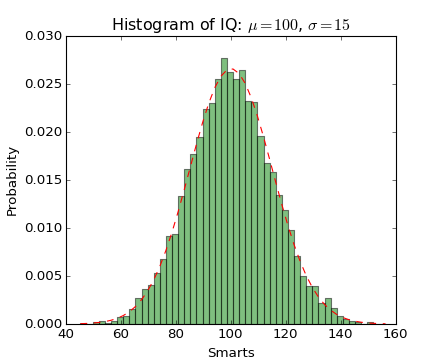`hist2d`(x, y, bins=10, range=None, normed=False, weights=None, cmin=None, cmax=None, **kwargs)

Make a 2D histogram plot.

Parameters: Returns: x, y: array_like, shape (n, ) : Input values bins: [None | int | [int, int] | array_like | [array, array]] : The bin specification: If int, the number of bins for the two dimensions (nx=ny=bins). If [int, int], the number of bins in each dimension (nx, ny = bins). If array_like, the bin edges for the two dimensions (x_edges=y_edges=bins). If [array, array], the bin edges in each dimension (x_edges, y_edges = bins). The default value is 10. range : array_like shape(2, 2), optional, default: None The leftmost and rightmost edges of the bins along each dimension (if not specified explicitly in the bins parameters): [[xmin, xmax], [ymin, ymax]]. All values outside of this range will be considered outliers and not tallied in the histogram. normed : boolean, optional, default: False Normalize histogram. weights : array_like, shape (n, ), optional, default: None An array of values w_i weighing each sample (x_i, y_i). cmin : scalar, optional, default: None All bins that has count less than cmin will not be displayed and these count values in the return value count histogram will also be set to nan upon return cmax : scalar, optional, default: None All bins that has count more than cmax will not be displayed (set to none before passing to imshow) and these count values in the return value count histogram will also be set to nan upon return The return value is ``(counts, xedges, yedges, Image)``. : kwargs : `pcolorfast()` properties.

`hist`
1D histogram

Notes

Rendering the histogram with a logarithmic color scale is accomplished by passing a `colors.LogNorm` instance to the norm keyword argument. Likewise, power-law normalization (similar in effect to gamma correction) can be accomplished with `colors.PowerNorm`.

Examples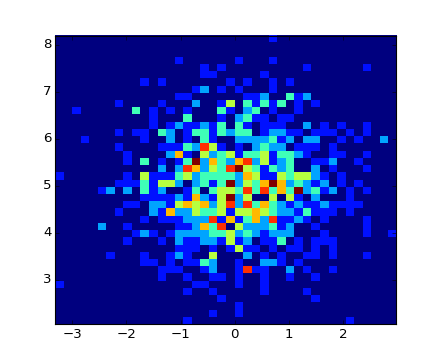`hitlist`(event)

List the children of the artist which contain the mouse event event.

`hlines`(y, xmin, xmax, colors=u'k', linestyles=u'solid', label=u'', **kwargs)

Plot horizontal lines at each `y` from `xmin` to `xmax`.

Parameters: Returns: y : scalar or sequence of scalar y-indexes where to plot the lines. xmin, xmax : scalar or 1D array_like Respective beginning and end of each line. If scalars are provided, all lines will have same length. colors : array_like of colors, optional, default: ‘k’ linestyles : [‘solid’ | ‘dashed’ | ‘dashdot’ | ‘dotted’], optional label : string, optional, default: ‘’ lines : `LineCollection` kwargs : `LineCollection` properties.

`vlines`
vertical lines

Examples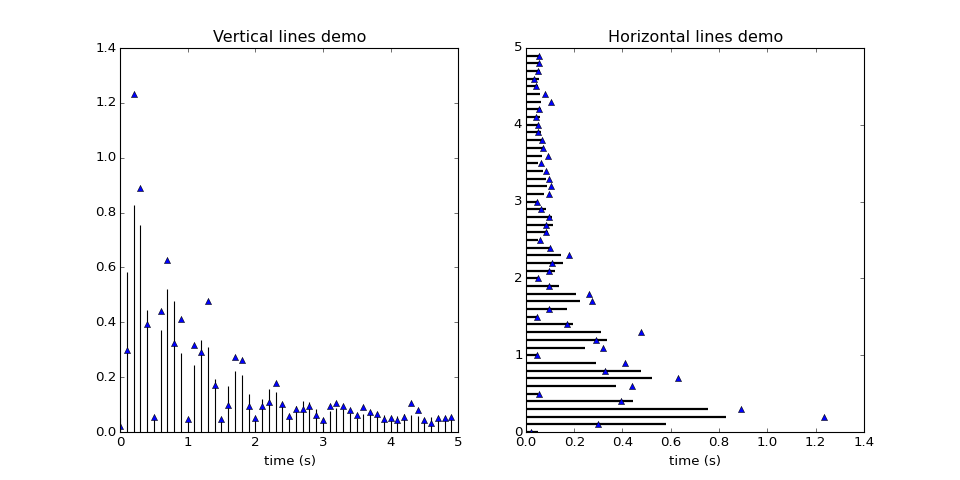`hold`(b=None)

Call signature:

```hold(b=None)
```

Set the hold state. If hold is None (default), toggle the hold state. Else set the hold state to boolean value b.

Examples:

```# toggle hold
hold()

# turn hold on
hold(True)

# turn hold off
hold(False)
```

When hold is True, subsequent plot commands will be added to the current axes. When hold is False, the current axes and figure will be cleared on the next plot command

`imshow`(X, cmap=None, norm=None, aspect=None, interpolation=None, alpha=None, vmin=None, vmax=None, origin=None, extent=None, shape=None, filternorm=1, filterrad=4.0, imlim=None, resample=None, url=None, **kwargs)

Display an image on the axes.

Parameters: Returns: X : array_like, shape (n, m) or (n, m, 3) or (n, m, 4) Display the image in `X` to current axes. `X` may be a float array, a uint8 array or a PIL image. If `X` is an array, it can have the following shapes: MxN – luminance (grayscale, float array only) MxNx3 – RGB (float or uint8 array) MxNx4 – RGBA (float or uint8 array) The value for each component of MxNx3 and MxNx4 float arrays should be in the range 0.0 to 1.0; MxN float arrays may be normalised. cmap : `Colormap`, optional, default: None If None, default to rc `image.cmap` value. `cmap` is ignored when `X` has RGB(A) information aspect : [‘auto’ | ‘equal’ | scalar], optional, default: None If ‘auto’, changes the image aspect ratio to match that of the axes. If ‘equal’, and `extent` is None, changes the axes aspect ratio to match that of the image. If `extent` is not `None`, the axes aspect ratio is changed to match that of the extent. If None, default to rc `image.aspect` value. interpolation : string, optional, default: None Acceptable values are ‘none’, ‘nearest’, ‘bilinear’, ‘bicubic’, ‘spline16’, ‘spline36’, ‘hanning’, ‘hamming’, ‘hermite’, ‘kaiser’, ‘quadric’, ‘catrom’, ‘gaussian’, ‘bessel’, ‘mitchell’, ‘sinc’, ‘lanczos’ If `interpolation` is None, default to rc `image.interpolation`. See also the `filternorm` and `filterrad` parameters. If `interpolation` is ‘none’, then no interpolation is performed on the Agg, ps and pdf backends. Other backends will fall back to ‘nearest’. norm : `Normalize`, optional, default: None A `Normalize` instance is used to scale luminance data to 0, 1. If `None`, use the default func:`normalize`. `norm` is only used if `X` is an array of floats. vmin, vmax : scalar, optional, default: None `vmin` and `vmax` are used in conjunction with norm to normalize luminance data. Note if you pass a `norm` instance, your settings for `vmin` and `vmax` will be ignored. alpha : scalar, optional, default: None The alpha blending value, between 0 (transparent) and 1 (opaque) origin : [‘upper’ | ‘lower’], optional, default: None Place the [0,0] index of the array in the upper left or lower left corner of the axes. If None, default to rc `image.origin`. extent : scalars (left, right, bottom, top), optional, default: None The location, in data-coordinates, of the lower-left and upper-right corners. If `None`, the image is positioned such that the pixel centers fall on zero-based (row, column) indices. shape : scalars (columns, rows), optional, default: None For raw buffer images filternorm : scalar, optional, default: 1 A parameter for the antigrain image resize filter. From the antigrain documentation, if `filternorm` = 1, the filter normalizes integer values and corrects the rounding errors. It doesn’t do anything with the source floating point values, it corrects only integers according to the rule of 1.0 which means that any sum of pixel weights must be equal to 1.0. So, the filter function must produce a graph of the proper shape. filterrad : scalar, optional, default: 4.0 The filter radius for filters that have a radius parameter, i.e. when interpolation is one of: ‘sinc’, ‘lanczos’ or ‘blackman’ image : `AxesImage` kwargs : `Artist` properties.

`matshow`
Plot a matrix or an array as an image.

Examples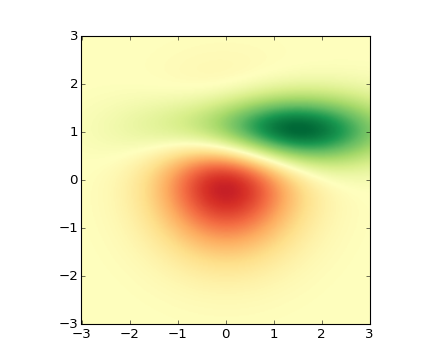`in_axes`(mouseevent)

Return True if the given mouseevent (in display coords) is in the Axes

`invert_xaxis`()

Invert the x-axis.

`invert_yaxis`()

Invert the y-axis.

`is_figure_set`()

Returns True if the artist is assigned to a `Figure`.

`is_transform_set`()

Returns True if `Artist` has a transform explicitly set.

`ishold`()

return the HOLD status of the axes

`legend`(*args, **kwargs)

Places a legend on the axes.

To make a legend for lines which already exist on the axes (via plot for instance), simply call this function with an iterable of strings, one for each legend item. For example:

```ax.plot([1, 2, 3])
ax.legend(['A simple line'])
```

However, in order to keep the “label” and the legend element instance together, it is preferable to specify the label either at artist creation, or by calling the `set_label()` method on the artist:

```line, = ax.plot([1, 2, 3], label='Inline label')
# Overwrite the label by calling the method.
line.set_label('Label via method')
ax.legend()
```

Specific lines can be excluded from the automatic legend element selection by defining a label starting with an underscore. This is default for all artists, so calling `legend()` without any arguments and without setting the labels manually will result in no legend being drawn.

For full control of which artists have a legend entry, it is possible to pass an iterable of legend artists followed by an iterable of legend labels respectively:

```legend((line1, line2, line3), ('label1', 'label2', 'label3'))
```
Parameters:

loc : int or string or pair of floats, default: 0

The location of the legend. Possible codes are:

Location String Location Code
‘best’ 0
‘upper right’ 1
‘upper left’ 2
‘lower left’ 3
‘lower right’ 4
‘right’ 5
‘center left’ 6
‘center right’ 7
‘lower center’ 8
‘upper center’ 9
‘center’ 10

Alternatively can be a 2-tuple giving `x, y` of the lower-left corner of the legend in axes coordinates (in which case `bbox_to_anchor` will be ignored).

bbox_to_anchor : `matplotlib.transforms.BboxBase` instance or tuple of floats

Specify any arbitrary location for the legend in `bbox_transform` coordinates (default Axes coordinates).

For example, to put the legend’s upper right hand corner in the center of the axes the following keywords can be used:

```loc='upper right', bbox_to_anchor=(0.5, 0.5)
```

ncol : integer

The number of columns that the legend has. Default is 1.

prop : None or `matplotlib.font_manager.FontProperties` or dict

The font properties of the legend. If None (default), the current `matplotlib.rcParams` will be used.

fontsize : int or float or {‘xx-small’, ‘x-small’, ‘small’, ‘medium’, ‘large’, ‘x-large’, ‘xx-large’}

Controls the font size of the legend. If the value is numeric the size will be the absolute font size in points. String values are relative to the current default font size. This argument is only used if `prop` is not specified.

numpoints : None or int

The number of marker points in the legend when creating a legend entry for a line/`matplotlib.lines.Line2D`. Default is `None` which will take the value from the `legend.numpoints` `rcParam`.

scatterpoints : None or int

The number of marker points in the legend when creating a legend entry for a scatter plot/ `matplotlib.collections.PathCollection`. Default is `None` which will take the value from the `legend.scatterpoints` `rcParam`.

scatteryoffsets : iterable of floats

The vertical offset (relative to the font size) for the markers created for a scatter plot legend entry. 0.0 is at the base the legend text, and 1.0 is at the top. To draw all markers at the same height, set to `[0.5]`. Default `[0.375, 0.5, 0.3125]`.

markerscale : None or int or float

The relative size of legend markers compared with the originally drawn ones. Default is `None` which will take the value from the `legend.markerscale` `rcParam`.

frameon : None or bool

Control whether a frame should be drawn around the legend. Default is `None` which will take the value from the `legend.frameon` `rcParam`.

fancybox : None or bool

Control whether round edges should be enabled around the `FancyBboxPatch` which makes up the legend’s background. Default is `None` which will take the value from the `legend.fancybox` `rcParam`.

Control whether to draw a shadow behind the legend. Default is `None` which will take the value from the `legend.shadow` `rcParam`.

framealpha : None or float

Control the alpha transparency of the legend’s frame. Default is `None` which will take the value from the `legend.framealpha` `rcParam`.

mode : {“expand”, None}

If `mode` is set to `"expand"` the legend will be horizontally expanded to fill the axes area (or `bbox_to_anchor` if defines the legend’s size).

bbox_transform : None or `matplotlib.transforms.Transform`

The transform for the bounding box (`bbox_to_anchor`). For a value of `None` (default) the Axes’ `transAxes` transform will be used.

title : str or None

The legend’s title. Default is no title (`None`).

The fractional whitespace inside the legend border. Measured in font-size units. Default is `None` which will take the value from the `legend.borderpad` `rcParam`.

labelspacing : float or None

The vertical space between the legend entries. Measured in font-size units. Default is `None` which will take the value from the `legend.labelspacing` `rcParam`.

handlelength : float or None

The length of the legend handles. Measured in font-size units. Default is `None` which will take the value from the `legend.handlelength` `rcParam`.

The pad between the legend handle and text. Measured in font-size units. Default is `None` which will take the value from the `legend.handletextpad` `rcParam`.

The pad between the axes and legend border. Measured in font-size units. Default is `None` which will take the value from the `legend.borderaxespad` `rcParam`.

columnspacing : float or None

The spacing between columns. Measured in font-size units. Default is `None` which will take the value from the `legend.columnspacing` `rcParam`.

handler_map : dict or None

The custom dictionary mapping instances or types to a legend handler. This `handler_map` updates the default handler map found at `matplotlib.legend.Legend.get_legend_handler_map()`.

Notes

Not all kinds of artist are supported by the legend command. See Legend guide for details.

Examples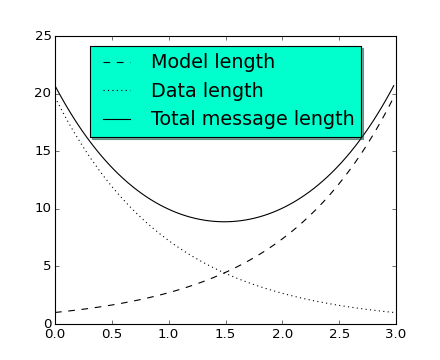`locator_params`(axis=u'both', tight=None, **kwargs)

Control behavior of tick locators.

Keyword arguments:

axis
[‘x’ | ‘y’ | ‘both’] Axis on which to operate; default is ‘both’.
tight
[True | False | None] Parameter passed to `autoscale_view()`. Default is None, for no change.

Remaining keyword arguments are passed to directly to the `set_params()` method.

Typically one might want to reduce the maximum number of ticks and use tight bounds when plotting small subplots, for example:

```ax.locator_params(tight=True, nbins=4)
```

Because the locator is involved in autoscaling, `autoscale_view()` is called automatically after the parameters are changed.

This presently works only for the `MaxNLocator` used by default on linear axes, but it may be generalized.

`loglog`(*args, **kwargs)

Make a plot with log scaling on both the x and y axis.

Call signature:

```loglog(*args, **kwargs)
```

`loglog()` supports all the keyword arguments of `plot()` and `matplotlib.axes.Axes.set_xscale()` / `matplotlib.axes.Axes.set_yscale()`.

Notable keyword arguments:

basex/basey: scalar > 1
Base of the x/y logarithm
subsx/subsy: [ None | sequence ]
The location of the minor x/y ticks; None defaults to autosubs, which depend on the number of decades in the plot; see `matplotlib.axes.Axes.set_xscale()` / `matplotlib.axes.Axes.set_yscale()` for details
Non-positive values in x or y can be masked as invalid, or clipped to a very small positive number

The remaining valid kwargs are `Line2D` properties:

Property Description
`agg_filter` unknown
`alpha` float (0.0 transparent through 1.0 opaque)
`animated` [True | False]
`antialiased` or aa [True | False]
`axes` an `Axes` instance
`clip_box` a `matplotlib.transforms.Bbox` instance
`clip_on` [True | False]
`clip_path` [ (`Path`, `Transform`) | `Patch` | None ]
`color` or c any matplotlib color
`contains` a callable function
`dash_capstyle` [‘butt’ | ‘round’ | ‘projecting’]
`dash_joinstyle` [‘miter’ | ‘round’ | ‘bevel’]
`dashes` sequence of on/off ink in points
`drawstyle` [‘default’ | ‘steps’ | ‘steps-pre’ | ‘steps-mid’ | ‘steps-post’]
`figure` a `matplotlib.figure.Figure` instance
`fillstyle` [‘full’ | ‘left’ | ‘right’ | ‘bottom’ | ‘top’ | ‘none’]
`gid` an id string
`label` string or anything printable with ‘%s’ conversion.
`linestyle` or ls [`'-'` | `'--'` | `'-.'` | `':'` | `'None'` | `' '` | `''`]
`linewidth` or lw float value in points
`lod` [True | False]
`marker` unknown
`markeredgecolor` or mec any matplotlib color
`markeredgewidth` or mew float value in points
`markerfacecolor` or mfc any matplotlib color
`markerfacecoloralt` or mfcalt any matplotlib color
`markersize` or ms float
`markevery` unknown
`path_effects` unknown
`picker` float distance in points or callable pick function `fn(artist, event)`
`pickradius` float distance in points
`rasterized` [True | False | None]
`sketch_params` unknown
`snap` unknown
`solid_capstyle` [‘butt’ | ‘round’ | ‘projecting’]
`solid_joinstyle` [‘miter’ | ‘round’ | ‘bevel’]
`transform` a `matplotlib.transforms.Transform` instance
`url` a url string
`visible` [True | False]
`xdata` 1D array
`ydata` 1D array
`zorder` any number

Example: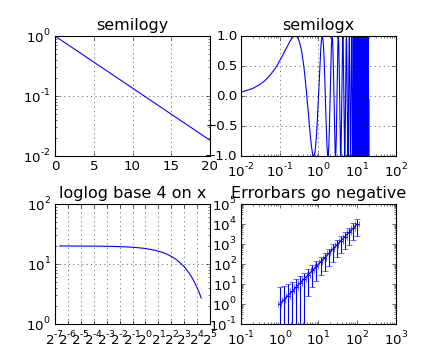`magnitude_spectrum`(x, Fs=None, Fc=None, window=None, pad_to=None, sides=None, scale=None, **kwargs)

Plot the magnitude spectrum.

Call signature:

```magnitude_spectrum(x, Fs=2, Fc=0,  window=mlab.window_hanning,
```

Compute the magnitude spectrum of x. Data is padded to a length of pad_to and the windowing function window is applied to the signal.

x: 1-D array or sequence
Array or sequence containing the data

Keyword arguments:

Fs: scalar
The sampling frequency (samples per time unit). It is used to calculate the Fourier frequencies, freqs, in cycles per time unit. The default value is 2.
window: callable or ndarray
A function or a vector of length NFFT. To create window vectors see `window_hanning()`, `window_none()`, `numpy.blackman()`, `numpy.hamming()`, `numpy.bartlett()`, `scipy.signal()`, `scipy.signal.get_window()`, etc. The default is `window_hanning()`. If a function is passed as the argument, it must take a data segment as an argument and return the windowed version of the segment.
sides: [ ‘default’ | ‘onesided’ | ‘twosided’ ]
Specifies which sides of the spectrum to return. Default gives the default behavior, which returns one-sided for real data and both for complex data. ‘onesided’ forces the return of a one-sided spectrum, while ‘twosided’ forces two-sided.
The number of points to which the data segment is padded when performing the FFT. While not increasing the actual resolution of the spectrum (the minimum distance between resolvable peaks), this can give more points in the plot, allowing for more detail. This corresponds to the n parameter in the call to fft(). The default is None, which sets pad_to equal to the length of the input signal (i.e. no padding).
scale: [ ‘default’ | ‘linear’ | ‘dB’ ]
The scaling of the values in the spec. ‘linear’ is no scaling. ‘dB’ returns the values in dB scale. When mode is ‘density’, this is dB power (10 * log10). Otherwise this is dB amplitude (20 * log10). ‘default’ is ‘linear’.
Fc: integer
The center frequency of x (defaults to 0), which offsets the x extents of the plot to reflect the frequency range used when a signal is acquired and then filtered and downsampled to baseband.

Returns the tuple (spectrum, freqs, line):

spectrum: 1-D array
The values for the magnitude spectrum before scaling (real valued)
freqs: 1-D array
The frequencies corresponding to the elements in spectrum
line: a `Line2D` instance
The line created by this function

kwargs control the `Line2D` properties:

Property Description
`agg_filter` unknown
`alpha` float (0.0 transparent through 1.0 opaque)
`animated` [True | False]
`antialiased` or aa [True | False]
`axes` an `Axes` instance
`clip_box` a `matplotlib.transforms.Bbox` instance
`clip_on` [True | False]
`clip_path` [ (`Path`, `Transform`) | `Patch` | None ]
`color` or c any matplotlib color
`contains` a callable function
`dash_capstyle` [‘butt’ | ‘round’ | ‘projecting’]
`dash_joinstyle` [‘miter’ | ‘round’ | ‘bevel’]
`dashes` sequence of on/off ink in points
`drawstyle` [‘default’ | ‘steps’ | ‘steps-pre’ | ‘steps-mid’ | ‘steps-post’]
`figure` a `matplotlib.figure.Figure` instance
`fillstyle` [‘full’ | ‘left’ | ‘right’ | ‘bottom’ | ‘top’ | ‘none’]
`gid` an id string
`label` string or anything printable with ‘%s’ conversion.
`linestyle` or ls [`'-'` | `'--'` | `'-.'` | `':'` | `'None'` | `' '` | `''`]
`linewidth` or lw float value in points
`lod` [True | False]
`marker` unknown
`markeredgecolor` or mec any matplotlib color
`markeredgewidth` or mew float value in points
`markerfacecolor` or mfc any matplotlib color
`markerfacecoloralt` or mfcalt any matplotlib color
`markersize` or ms float
`markevery` unknown
`path_effects` unknown
`picker` float distance in points or callable pick function `fn(artist, event)`
`pickradius` float distance in points
`rasterized` [True | False | None]
`sketch_params` unknown
`snap` unknown
`solid_capstyle` [‘butt’ | ‘round’ | ‘projecting’]
`solid_joinstyle` [‘miter’ | ‘round’ | ‘bevel’]
`transform` a `matplotlib.transforms.Transform` instance
`url` a url string
`visible` [True | False]
`xdata` 1D array
`ydata` 1D array
`zorder` any number

Example:`psd()`
`psd()` plots the power spectral density.`.
`angle_spectrum()`
`angle_spectrum()` plots the angles of the corresponding frequencies.
`phase_spectrum()`
`phase_spectrum()` plots the phase (unwrapped angle) of the corresponding frequencies.
`specgram()`
`specgram()` can plot the magnitude spectrum of segments within the signal in a colormap.
`margins`(*args, **kw)

Set or retrieve autoscaling margins.

signatures:

```margins()
```

returns xmargin, ymargin

```margins(margin)

margins(xmargin, ymargin)

margins(x=xmargin, y=ymargin)

margins(..., tight=False)
```

All three forms above set the xmargin and ymargin parameters. All keyword parameters are optional. A single argument specifies both xmargin and ymargin. The tight parameter is passed to `autoscale_view()`, which is executed after a margin is changed; the default here is True, on the assumption that when margins are specified, no additional padding to match tick marks is usually desired. Setting tight to None will preserve the previous setting.

Specifying any margin changes only the autoscaling; for example, if xmargin is not None, then xmargin times the X data interval will be added to each end of that interval before it is used in autoscaling.

`matshow`(Z, **kwargs)

Plot a matrix or array as an image.

The matrix will be shown the way it would be printed, with the first row at the top. Row and column numbering is zero-based.

Parameters: Returns: Z : array_like shape (n, m) The matrix to be displayed. image : `AxesImage` kwargs : `imshow` arguments Sets `origin` to ‘upper’, ‘interpolation’ to ‘nearest’ and ‘aspect’ to equal.

`imshow`
plot an image

Examples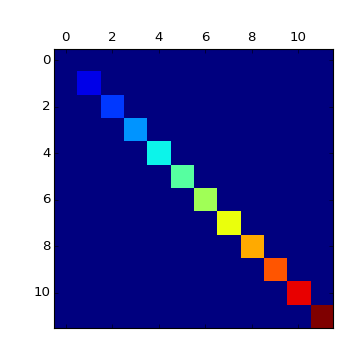(png, hires.png, pdf)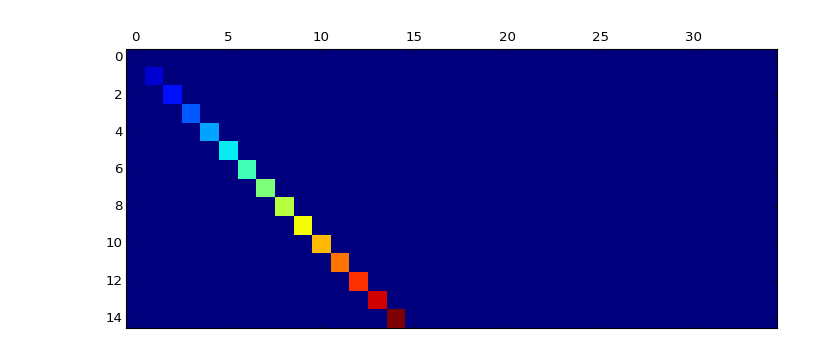(png, hires.png, pdf)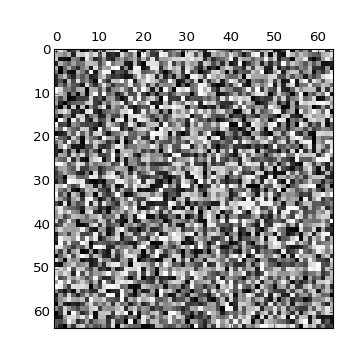(png, hires.png, pdf)

`minorticks_off`()

Remove minor ticks from the axes.

`minorticks_on`()

Add autoscaling minor ticks to the axes.

`name` = u'rectilinear'
`pchanged`()

Fire an event when property changed, calling all of the registered callbacks.

`pcolor`(*args, **kwargs)

Create a pseudocolor plot of a 2-D array.

Note

pcolor can be very slow for large arrays; consider using the similar but much faster `pcolormesh()` instead.

Call signatures:

```pcolor(C, **kwargs)
pcolor(X, Y, C, **kwargs)
```

C is the array of color values.

X and Y, if given, specify the (x, y) coordinates of the colored quadrilaterals; the quadrilateral for C[i,j] has corners at:

```(X[i,   j],   Y[i,   j]),
(X[i,   j+1], Y[i,   j+1]),
(X[i+1, j],   Y[i+1, j]),
(X[i+1, j+1], Y[i+1, j+1]).
```

Ideally the dimensions of X and Y should be one greater than those of C; if the dimensions are the same, then the last row and column of C will be ignored.

Note that the the column index corresponds to the x-coordinate, and the row index corresponds to y; for details, see the Grid Orientation section below.

If either or both of X and Y are 1-D arrays or column vectors, they will be expanded as needed into the appropriate 2-D arrays, making a rectangular grid.

X, Y and C may be masked arrays. If either C[i, j], or one of the vertices surrounding C[i,j] (X or Y at [i, j], [i+1, j], [i, j+1],[i+1, j+1]) is masked, nothing is plotted.

Keyword arguments:

cmap: [ None | Colormap ]
A `matplotlib.colors.Colormap` instance. If None, use rc settings.
norm: [ None | Normalize ]
An `matplotlib.colors.Normalize` instance is used to scale luminance data to 0,1. If None, defaults to `normalize()`.
vmin/vmax: [ None | scalar ]
vmin and vmax are used in conjunction with norm to normalize luminance data. If either is None, it is autoscaled to the respective min or max of the color array C. If not None, vmin or vmax passed in here override any pre-existing values supplied in the norm instance.
shading: [ ‘flat’ | ‘faceted’ ]

If ‘faceted’, a black grid is drawn around each rectangle; if ‘flat’, edges are not drawn. Default is ‘flat’, contrary to MATLAB.

edgecolors: [ None | `'none'` | color | color sequence]

If None, the rc setting is used by default.

If `'none'`, edges will not be visible.

An mpl color or sequence of colors will set the edge color

alpha: `0 <= scalar <= 1` or None
the alpha blending value
snap: bool
Whether to snap the mesh to pixel boundaries.

Return value is a `matplotlib.collections.Collection` instance.

The grid orientation follows the MATLAB convention: an array C with shape (nrows, ncolumns) is plotted with the column number as X and the row number as Y, increasing up; hence it is plotted the way the array would be printed, except that the Y axis is reversed. That is, C is taken as C*(*y, x).

Similarly for `meshgrid()`:

```x = np.arange(5)
y = np.arange(3)
X, Y = np.meshgrid(x, y)
```

is equivalent to:

```X = array([[0, 1, 2, 3, 4],
[0, 1, 2, 3, 4],
[0, 1, 2, 3, 4]])

Y = array([[0, 0, 0, 0, 0],
[1, 1, 1, 1, 1],
[2, 2, 2, 2, 2]])
```

so if you have:

```C = rand(len(x), len(y))
```

then you need to transpose C:

```pcolor(X, Y, C.T)
```

or:

```pcolor(C.T)
```

MATLAB `pcolor()` always discards the last row and column of C, but matplotlib displays the last row and column if X and Y are not specified, or if X and Y have one more row and column than C.

kwargs can be used to control the `PolyCollection` properties:

Property Description
`agg_filter` unknown
`alpha` float or None
`animated` [True | False]
`antialiased` or antialiaseds Boolean or sequence of booleans
`array` unknown
`axes` an `Axes` instance
`clim` a length 2 sequence of floats
`clip_box` a `matplotlib.transforms.Bbox` instance
`clip_on` [True | False]
`clip_path` [ (`Path`, `Transform`) | `Patch` | None ]
`cmap` a colormap or registered colormap name
`color` matplotlib color arg or sequence of rgba tuples
`contains` a callable function
`edgecolor` or edgecolors matplotlib color arg or sequence of rgba tuples
`facecolor` or facecolors matplotlib color arg or sequence of rgba tuples
`figure` a `matplotlib.figure.Figure` instance
`gid` an id string
`hatch` [ ‘/’ | ‘\’ | ‘|’ | ‘-‘ | ‘+’ | ‘x’ | ‘o’ | ‘O’ | ‘.’ | ‘*’ ]
`label` string or anything printable with ‘%s’ conversion.
`linestyle` or linestyles or dashes [‘solid’ | ‘dashed’, ‘dashdot’, ‘dotted’ | (offset, on-off-dash-seq) ]
`linewidth` or lw or linewidths float or sequence of floats
`lod` [True | False]
`norm` unknown
`offset_position` unknown
`offsets` float or sequence of floats
`path_effects` unknown
`picker` [None|float|boolean|callable]
`pickradius` unknown
`rasterized` [True | False | None]
`sketch_params` unknown
`snap` unknown
`transform` `Transform` instance
`url` a url string
`urls` unknown
`visible` [True | False]
`zorder` any number

Note

The default antialiaseds is False if the default edgecolors*=”none” is used. This eliminates artificial lines at patch boundaries, and works regardless of the value of alpha. If *edgecolors is not “none”, then the default antialiaseds is taken from rcParams[‘patch.antialiased’], which defaults to True. Stroking the edges may be preferred if alpha is 1, but will cause artifacts otherwise.

`pcolormesh()`
For an explanation of the differences between pcolor and pcolormesh.
`pcolorfast`(*args, **kwargs)

pseudocolor plot of a 2-D array

Experimental; this is a pcolor-type method that provides the fastest possible rendering with the Agg backend, and that can handle any quadrilateral grid. It supports only flat shading (no outlines), it lacks support for log scaling of the axes, and it does not have a pyplot wrapper.

Call signatures:

```ax.pcolorfast(C, **kwargs)
ax.pcolorfast(xr, yr, C, **kwargs)
ax.pcolorfast(x, y, C, **kwargs)
ax.pcolorfast(X, Y, C, **kwargs)
```

C is the 2D array of color values corresponding to quadrilateral cells. Let (nr, nc) be its shape. C may be a masked array.

`ax.pcolorfast(C, **kwargs)` is equivalent to `ax.pcolorfast([0,nc], [0,nr], C, **kwargs)`

xr, yr specify the ranges of x and y corresponding to the rectangular region bounding C. If:

```xr = [x0, x1]
```

and:

```yr = [y0,y1]
```

then x goes from x0 to x1 as the second index of C goes from 0 to nc, etc. (x0, y0) is the outermost corner of cell (0,0), and (x1, y1) is the outermost corner of cell (nr-1, nc-1). All cells are rectangles of the same size. This is the fastest version.

x, y are 1D arrays of length nc +1 and nr +1, respectively, giving the x and y boundaries of the cells. Hence the cells are rectangular but the grid may be nonuniform. The speed is intermediate. (The grid is checked, and if found to be uniform the fast version is used.)

X and Y are 2D arrays with shape (nr +1, nc +1) that specify the (x,y) coordinates of the corners of the colored quadrilaterals; the quadrilateral for C[i,j] has corners at (X[i,j],Y[i,j]), (X[i,j+1],Y[i,j+1]), (X[i+1,j],Y[i+1,j]), (X[i+1,j+1],Y[i+1,j+1]). The cells need not be rectangular. This is the most general, but the slowest to render. It may produce faster and more compact output using ps, pdf, and svg backends, however.

Note that the the column index corresponds to the x-coordinate, and the row index corresponds to y; for details, see the “Grid Orientation” section below.

Optional keyword arguments:

cmap: [ None | Colormap ]
A `matplotlib.colors.Colormap` instance from cm. If None, use rc settings.
norm: [ None | Normalize ]
A `matplotlib.colors.Normalize` instance is used to scale luminance data to 0,1. If None, defaults to normalize()
vmin/vmax: [ None | scalar ]
vmin and vmax are used in conjunction with norm to normalize luminance data. If either are None, the min and max of the color array C is used. If you pass a norm instance, vmin and vmax will be None.
alpha: `0 <= scalar <= 1` or None
the alpha blending value

Return value is an image if a regular or rectangular grid is specified, and a `QuadMesh` collection in the general quadrilateral case.

`pcolormesh`(*args, **kwargs)

Call signatures:

```pcolormesh(C)
pcolormesh(X, Y, C)
pcolormesh(C, **kwargs)
```

Create a pseudocolor plot of a 2-D array.

pcolormesh is similar to `pcolor()`, but uses a different mechanism and returns a different object; pcolor returns a `PolyCollection` but pcolormesh returns a `QuadMesh`. It is much faster, so it is almost always preferred for large arrays.

C may be a masked array, but X and Y may not. Masked array support is implemented via cmap and norm; in contrast, `pcolor()` simply does not draw quadrilaterals with masked colors or vertices.

Keyword arguments:

cmap: [ None | Colormap ]
A `matplotlib.colors.Colormap` instance. If None, use rc settings.
norm: [ None | Normalize ]
A `matplotlib.colors.Normalize` instance is used to scale luminance data to 0,1. If None, defaults to `normalize()`.
vmin/vmax: [ None | scalar ]
vmin and vmax are used in conjunction with norm to normalize luminance data. If either is None, it is autoscaled to the respective min or max of the color array C. If not None, vmin or vmax passed in here override any pre-existing values supplied in the norm instance.
shading: [ ‘flat’ | ‘gouraud’ ]
edgecolors: [None | `'None'` | `'face'` | color |
color sequence]

If None, the rc setting is used by default.

If `'None'`, edges will not be visible.

If `'face'`, edges will have the same color as the faces.

An mpl color or sequence of colors will set the edge color

alpha: `0 <= scalar <= 1` or None
the alpha blending value

Return value is a `matplotlib.collections.QuadMesh` object.

kwargs can be used to control the `matplotlib.collections.QuadMesh` properties:

Property Description
`agg_filter` unknown
`alpha` float or None
`animated` [True | False]
`antialiased` or antialiaseds Boolean or sequence of booleans
`array` unknown
`axes` an `Axes` instance
`clim` a length 2 sequence of floats
`clip_box` a `matplotlib.transforms.Bbox` instance
`clip_on` [True | False]
`clip_path` [ (`Path`, `Transform`) | `Patch` | None ]
`cmap` a colormap or registered colormap name
`color` matplotlib color arg or sequence of rgba tuples
`contains` a callable function
`edgecolor` or edgecolors matplotlib color arg or sequence of rgba tuples
`facecolor` or facecolors matplotlib color arg or sequence of rgba tuples
`figure` a `matplotlib.figure.Figure` instance
`gid` an id string
`hatch` [ ‘/’ | ‘\’ | ‘|’ | ‘-‘ | ‘+’ | ‘x’ | ‘o’ | ‘O’ | ‘.’ | ‘*’ ]
`label` string or anything printable with ‘%s’ conversion.
`linestyle` or linestyles or dashes [‘solid’ | ‘dashed’, ‘dashdot’, ‘dotted’ | (offset, on-off-dash-seq) ]
`linewidth` or lw or linewidths float or sequence of floats
`lod` [True | False]
`norm` unknown
`offset_position` unknown
`offsets` float or sequence of floats
`path_effects` unknown
`picker` [None|float|boolean|callable]
`pickradius` unknown
`rasterized` [True | False | None]
`sketch_params` unknown
`snap` unknown
`transform` `Transform` instance
`url` a url string
`urls` unknown
`visible` [True | False]
`zorder` any number

`pcolor()`
For an explanation of the grid orientation and the expansion of 1-D X and/or Y to 2-D arrays.
`phase_spectrum`(x, Fs=None, Fc=None, window=None, pad_to=None, sides=None, **kwargs)

Plot the phase spectrum.

Call signature:

```phase_spectrum(x, Fs=2, Fc=0,  window=mlab.window_hanning,
```

Compute the phase spectrum (unwrapped angle spectrum) of x. Data is padded to a length of pad_to and the windowing function window is applied to the signal.

x: 1-D array or sequence
Array or sequence containing the data

Keyword arguments:

Fs: scalar
The sampling frequency (samples per time unit). It is used to calculate the Fourier frequencies, freqs, in cycles per time unit. The default value is 2.
window: callable or ndarray
A function or a vector of length NFFT. To create window vectors see `window_hanning()`, `window_none()`, `numpy.blackman()`, `numpy.hamming()`, `numpy.bartlett()`, `scipy.signal()`, `scipy.signal.get_window()`, etc. The default is `window_hanning()`. If a function is passed as the argument, it must take a data segment as an argument and return the windowed version of the segment.
sides: [ ‘default’ | ‘onesided’ | ‘twosided’ ]
Specifies which sides of the spectrum to return. Default gives the default behavior, which returns one-sided for real data and both for complex data. ‘onesided’ forces the return of a one-sided spectrum, while ‘twosided’ forces two-sided.
The number of points to which the data segment is padded when performing the FFT. While not increasing the actual resolution of the spectrum (the minimum distance between resolvable peaks), this can give more points in the plot, allowing for more detail. This corresponds to the n parameter in the call to fft(). The default is None, which sets pad_to equal to the length of the input signal (i.e. no padding).
Fc: integer
The center frequency of x (defaults to 0), which offsets the x extents of the plot to reflect the frequency range used when a signal is acquired and then filtered and downsampled to baseband.

Returns the tuple (spectrum, freqs, line):

spectrum: 1-D array
The values for the phase spectrum in radians (real valued)
freqs: 1-D array
The frequencies corresponding to the elements in spectrum
line: a `Line2D` instance
The line created by this function

kwargs control the `Line2D` properties:

Property Description
`agg_filter` unknown
`alpha` float (0.0 transparent through 1.0 opaque)
`animated` [True | False]
`antialiased` or aa [True | False]
`axes` an `Axes` instance
`clip_box` a `matplotlib.transforms.Bbox` instance
`clip_on` [True | False]
`clip_path` [ (`Path`, `Transform`) | `Patch` | None ]
`color` or c any matplotlib color
`contains` a callable function
`dash_capstyle` [‘butt’ | ‘round’ | ‘projecting’]
`dash_joinstyle` [‘miter’ | ‘round’ | ‘bevel’]
`dashes` sequence of on/off ink in points
`drawstyle` [‘default’ | ‘steps’ | ‘steps-pre’ | ‘steps-mid’ | ‘steps-post’]
`figure` a `matplotlib.figure.Figure` instance
`fillstyle` [‘full’ | ‘left’ | ‘right’ | ‘bottom’ | ‘top’ | ‘none’]
`gid` an id string
`label` string or anything printable with ‘%s’ conversion.
`linestyle` or ls [`'-'` | `'--'` | `'-.'` | `':'` | `'None'` | `' '` | `''`]
`linewidth` or lw float value in points
`lod` [True | False]
`marker` unknown
`markeredgecolor` or mec any matplotlib color
`markeredgewidth` or mew float value in points
`markerfacecolor` or mfc any matplotlib color
`markerfacecoloralt` or mfcalt any matplotlib color
`markersize` or ms float
`markevery` unknown
`path_effects` unknown
`picker` float distance in points or callable pick function `fn(artist, event)`
`pickradius` float distance in points
`rasterized` [True | False | None]
`sketch_params` unknown
`snap` unknown
`solid_capstyle` [‘butt’ | ‘round’ | ‘projecting’]
`solid_joinstyle` [‘miter’ | ‘round’ | ‘bevel’]
`transform` a `matplotlib.transforms.Transform` instance
`url` a url string
`visible` [True | False]
`xdata` 1D array
`ydata` 1D array
`zorder` any number

Example:`magnitude_spectrum()`
`magnitude_spectrum()` plots the magnitudes of the corresponding frequencies.
`angle_spectrum()`
`angle_spectrum()` plots the wrapped version of this function.
`specgram()`
`specgram()` can plot the phase spectrum of segments within the signal in a colormap.
`pick`(*args)

Call signature:

```pick(mouseevent)
```

each child artist will fire a pick event if mouseevent is over the artist and the artist has picker set

`pickable`()

Return True if `Artist` is pickable.

`pie`(x, explode=None, labels=None, colors=None, autopct=None, pctdistance=0.6, shadow=False, labeldistance=1.1, startangle=None, radius=None, counterclock=True, wedgeprops=None, textprops=None)

Plot a pie chart.

Call signature:

```pie(x, explode=None, labels=None,
colors=('b', 'g', 'r', 'c', 'm', 'y', 'k', 'w'),
counterclock=True, wedgeprops=None, textprops=None)
```

Make a pie chart of array x. The fractional area of each wedge is given by x/sum(x). If sum(x) <= 1, then the values of x give the fractional area directly and the array will not be normalized. The wedges are plotted counterclockwise, by default starting from the x-axis.

Keyword arguments:

explode: [ None | len(x) sequence ]
If not None, is a `len(x)` array which specifies the fraction of the radius with which to offset each wedge.
colors: [ None | color sequence ]
A sequence of matplotlib color args through which the pie chart will cycle.
labels: [ None | len(x) sequence of strings ]
A sequence of strings providing the labels for each wedge
autopct: [ None | format string | format function ]
If not None, is a string or function used to label the wedges with their numeric value. The label will be placed inside the wedge. If it is a format string, the label will be `fmt%pct`. If it is a function, it will be called.
pctdistance: scalar
The ratio between the center of each pie slice and the start of the text generated by autopct. Ignored if autopct is None; default is 0.6.
labeldistance: scalar
The radial distance at which the pie labels are drawn
shadow: [ False | True ]
Draw a shadow beneath the pie.
startangle: [ None | Offset angle ]
If not None, rotates the start of the pie chart by angle degrees counterclockwise from the x-axis.

radius: [ None | scalar ] The radius of the pie, if radius is None it will be set to 1.

counterclock: [ False | True ]
Specify fractions direction, clockwise or counterclockwise.
wedgeprops: [ None | dict of key value pairs ]
Dict of arguments passed to the wedge objects making the pie. For example, you can pass in wedgeprops = { ‘linewidth’ : 3 } to set the width of the wedge border lines equal to 3. For more details, look at the doc/arguments of the wedge object. By default `clip_on=False`.
textprops: [ None | dict of key value pairs ]
Dict of arguments to pass to the text objects.

The pie chart will probably look best if the figure and axes are square, or the Axes aspect is equal. e.g.:

```figure(figsize=(8,8))
ax = axes([0.1, 0.1, 0.8, 0.8])
```

or:

```axes(aspect=1)
```
Return value:

If autopct is None, return the tuple (patches, texts):

If autopct is not None, return the tuple (patches, texts, autotexts), where patches and texts are as above, and autotexts is a list of `Text` instances for the numeric labels.

`plot`(*args, **kwargs)

Plot lines and/or markers to the `Axes`. args is a variable length argument, allowing for multiple x, y pairs with an optional format string. For example, each of the following is legal:

```plot(x, y)        # plot x and y using default line style and color
plot(x, y, 'bo')  # plot x and y using blue circle markers
plot(y)           # plot y using x as index array 0..N-1
plot(y, 'r+')     # ditto, but with red plusses
```

If x and/or y is 2-dimensional, then the corresponding columns will be plotted.

An arbitrary number of x, y, fmt groups can be specified, as in:

```a.plot(x1, y1, 'g^', x2, y2, 'g-')
```

Return value is a list of lines that were added.

By default, each line is assigned a different color specified by a ‘color cycle’. To change this behavior, you can edit the axes.color_cycle rcParam.

The following format string characters are accepted to control the line style or marker:

character description
`'-'` solid line style
`'--'` dashed line style
`'-.'` dash-dot line style
`':'` dotted line style
`'.'` point marker
`','` pixel marker
`'o'` circle marker
`'v'` triangle_down marker
`'^'` triangle_up marker
`'<'` triangle_left marker
`'>'` triangle_right marker
`'1'` tri_down marker
`'2'` tri_up marker
`'3'` tri_left marker
`'4'` tri_right marker
`'s'` square marker
`'p'` pentagon marker
`'*'` star marker
`'h'` hexagon1 marker
`'H'` hexagon2 marker
`'+'` plus marker
`'x'` x marker
`'D'` diamond marker
`'d'` thin_diamond marker
`'|'` vline marker
`'_'` hline marker

The following color abbreviations are supported:

character color
‘b’ blue
‘g’ green
‘r’ red
‘c’ cyan
‘m’ magenta
‘y’ yellow
‘k’ black
‘w’ white

In addition, you can specify colors in many weird and wonderful ways, including full names (`'green'`), hex strings (`'#008000'`), RGB or RGBA tuples (`(0,1,0,1)`) or grayscale intensities as a string (`'0.8'`). Of these, the string specifications can be used in place of a `fmt` group, but the tuple forms can be used only as `kwargs`.

Line styles and colors are combined in a single format string, as in `'bo'` for blue circles.

The kwargs can be used to set line properties (any property that has a `set_*` method). You can use this to set a line label (for auto legends), linewidth, anitialising, marker face color, etc. Here is an example:

```plot([1,2,3], [1,2,3], 'go-', label='line 1', linewidth=2)
plot([1,2,3], [1,4,9], 'rs',  label='line 2')
axis([0, 4, 0, 10])
legend()
```

If you make multiple lines with one plot command, the kwargs apply to all those lines, e.g.:

```plot(x1, y1, x2, y2, antialised=False)
```

Neither line will be antialiased.

You do not need to use format strings, which are just abbreviations. All of the line properties can be controlled by keyword arguments. For example, you can set the color, marker, linestyle, and markercolor with:

```plot(x, y, color='green', linestyle='dashed', marker='o',
markerfacecolor='blue', markersize=12).
```

See `Line2D` for details.

The kwargs are `Line2D` properties:

Property Description
`agg_filter` unknown
`alpha` float (0.0 transparent through 1.0 opaque)
`animated` [True | False]
`antialiased` or aa [True | False]
`axes` an `Axes` instance
`clip_box` a `matplotlib.transforms.Bbox` instance
`clip_on` [True | False]
`clip_path` [ (`Path`, `Transform`) | `Patch` | None ]
`color` or c any matplotlib color
`contains` a callable function
`dash_capstyle` [‘butt’ | ‘round’ | ‘projecting’]
`dash_joinstyle` [‘miter’ | ‘round’ | ‘bevel’]
`dashes` sequence of on/off ink in points
`drawstyle` [‘default’ | ‘steps’ | ‘steps-pre’ | ‘steps-mid’ | ‘steps-post’]
`figure` a `matplotlib.figure.Figure` instance
`fillstyle` [‘full’ | ‘left’ | ‘right’ | ‘bottom’ | ‘top’ | ‘none’]
`gid` an id string
`label` string or anything printable with ‘%s’ conversion.
`linestyle` or ls [`'-'` | `'--'` | `'-.'` | `':'` | `'None'` | `' '` | `''`]
`linewidth` or lw float value in points
`lod` [True | False]
`marker` unknown
`markeredgecolor` or mec any matplotlib color
`markeredgewidth` or mew float value in points
`markerfacecolor` or mfc any matplotlib color
`markerfacecoloralt` or mfcalt any matplotlib color
`markersize` or ms float
`markevery` unknown
`path_effects` unknown
`picker` float distance in points or callable pick function `fn(artist, event)`
`pickradius` float distance in points
`rasterized` [True | False | None]
`sketch_params` unknown
`snap` unknown
`solid_capstyle` [‘butt’ | ‘round’ | ‘projecting’]
`solid_joinstyle` [‘miter’ | ‘round’ | ‘bevel’]
`transform` a `matplotlib.transforms.Transform` instance
`url` a url string
`visible` [True | False]
`xdata` 1D array
`ydata` 1D array
`zorder` any number

kwargs scalex and scaley, if defined, are passed on to `autoscale_view()` to determine whether the x and y axes are autoscaled; the default is True.

`plot_date`(x, y, fmt=u'o', tz=None, xdate=True, ydate=False, **kwargs)

Plot with data with dates.

Call signature:

```plot_date(x, y, fmt='bo', tz=None, xdate=True,
ydate=False, **kwargs)
```

Similar to the `plot()` command, except the x or y (or both) data is considered to be dates, and the axis is labeled accordingly.

x and/or y can be a sequence of dates represented as float days since 0001-01-01 UTC.

Keyword arguments:

fmt: string
The plot format string.
tz: [ None | timezone string | `tzinfo` instance]
The time zone to use in labeling dates. If None, defaults to rc value.
xdate: [ True | False ]
If True, the x-axis will be labeled with dates.
ydate: [ False | True ]
If True, the y-axis will be labeled with dates.

Note if you are using custom date tickers and formatters, it may be necessary to set the formatters/locators after the call to `plot_date()` since `plot_date()` will set the default tick locator to `matplotlib.dates.AutoDateLocator` (if the tick locator is not already set to a `matplotlib.dates.DateLocator` instance) and the default tick formatter to `matplotlib.dates.AutoDateFormatter` (if the tick formatter is not already set to a `matplotlib.dates.DateFormatter` instance).

Valid kwargs are `Line2D` properties:

Property Description
`agg_filter` unknown
`alpha` float (0.0 transparent through 1.0 opaque)
`animated` [True | False]
`antialiased` or aa [True | False]
`axes` an `Axes` instance
`clip_box` a `matplotlib.transforms.Bbox` instance
`clip_on` [True | False]
`clip_path` [ (`Path`, `Transform`) | `Patch` | None ]
`color` or c any matplotlib color
`contains` a callable function
`dash_capstyle` [‘butt’ | ‘round’ | ‘projecting’]
`dash_joinstyle` [‘miter’ | ‘round’ | ‘bevel’]
`dashes` sequence of on/off ink in points
`drawstyle` [‘default’ | ‘steps’ | ‘steps-pre’ | ‘steps-mid’ | ‘steps-post’]
`figure` a `matplotlib.figure.Figure` instance
`fillstyle` [‘full’ | ‘left’ | ‘right’ | ‘bottom’ | ‘top’ | ‘none’]
`gid` an id string
`label` string or anything printable with ‘%s’ conversion.
`linestyle` or ls [`'-'` | `'--'` | `'-.'` | `':'` | `'None'` | `' '` | `''`]
`linewidth` or lw float value in points
`lod` [True | False]
`marker` unknown
`markeredgecolor` or mec any matplotlib color
`markeredgewidth` or mew float value in points
`markerfacecolor` or mfc any matplotlib color
`markerfacecoloralt` or mfcalt any matplotlib color
`markersize` or ms float
`markevery` unknown
`path_effects` unknown
`picker` float distance in points or callable pick function `fn(artist, event)`
`pickradius` float distance in points
`rasterized` [True | False | None]
`sketch_params` unknown
`snap` unknown
`solid_capstyle` [‘butt’ | ‘round’ | ‘projecting’]
`solid_joinstyle` [‘miter’ | ‘round’ | ‘bevel’]
`transform` a `matplotlib.transforms.Transform` instance
`url` a url string
`visible` [True | False]
`xdata` 1D array
`ydata` 1D array
`zorder` any number

`dates` for helper functions

`date2num()`, `num2date()` and `drange()` for help on creating the required floating point dates.

`properties`()

return a dictionary mapping property name -> value for all Artist props

`psd`(x, NFFT=None, Fs=None, Fc=None, detrend=None, window=None, noverlap=None, pad_to=None, sides=None, scale_by_freq=None, return_line=None, **kwargs)

Plot the power spectral density.

Call signature:

```psd(x, NFFT=256, Fs=2, Fc=0, detrend=mlab.detrend_none,
sides='default', scale_by_freq=None, return_line=None, **kwargs)
```

The power spectral density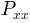by Welch’s average periodogram method. The vector x is divided into NFFT length segments. Each segment is detrended by function detrend and windowed by function window. noverlap gives the length of the overlap between segments. The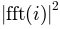of each segment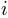are averaged to compute, with a scaling to correct for power loss due to windowing.

If len(x) < NFFT, it will be zero padded to NFFT.

x: 1-D array or sequence
Array or sequence containing the data

Keyword arguments:

Fs: scalar
The sampling frequency (samples per time unit). It is used to calculate the Fourier frequencies, freqs, in cycles per time unit. The default value is 2.
window: callable or ndarray
A function or a vector of length NFFT. To create window vectors see `window_hanning()`, `window_none()`, `numpy.blackman()`, `numpy.hamming()`, `numpy.bartlett()`, `scipy.signal()`, `scipy.signal.get_window()`, etc. The default is `window_hanning()`. If a function is passed as the argument, it must take a data segment as an argument and return the windowed version of the segment.
sides: [ ‘default’ | ‘onesided’ | ‘twosided’ ]
Specifies which sides of the spectrum to return. Default gives the default behavior, which returns one-sided for real data and both for complex data. ‘onesided’ forces the return of a one-sided spectrum, while ‘twosided’ forces two-sided.
The number of points to which the data segment is padded when performing the FFT. This can be different from NFFT, which specifies the number of data points used. While not increasing the actual resolution of the spectrum (the minimum distance between resolvable peaks), this can give more points in the plot, allowing for more detail. This corresponds to the n parameter in the call to fft(). The default is None, which sets pad_to equal to NFFT
NFFT: integer
The number of data points used in each block for the FFT. A power 2 is most efficient. The default value is 256. This should NOT be used to get zero padding, or the scaling of the result will be incorrect. Use pad_to for this instead.
detrend: [ ‘default’ | ‘constant’ | ‘mean’ | ‘linear’ | ‘none’] or
callable

The function applied to each segment before fft-ing, designed to remove the mean or linear trend. Unlike in MATLAB, where the detrend parameter is a vector, in matplotlib is it a function. The `pylab` module defines `detrend_none()`, `detrend_mean()`, and `detrend_linear()`, but you can use a custom function as well. You can also use a string to choose one of the functions. ‘default’, ‘constant’, and ‘mean’ call `detrend_mean()`. ‘linear’ calls `detrend_linear()`. ‘none’ calls `detrend_none()`.

scale_by_freq: boolean
Specifies whether the resulting density values should be scaled by the scaling frequency, which gives density in units of Hz^-1. This allows for integration over the returned frequency values. The default is True for MATLAB compatibility.
noverlap: integer
The number of points of overlap between segments. The default value is 0 (no overlap).
Fc: integer
The center frequency of x (defaults to 0), which offsets the x extents of the plot to reflect the frequency range used when a signal is acquired and then filtered and downsampled to baseband.
return_line: bool
Whether to include the line object plotted in the returned values. Default is False.

If return_line is False, returns the tuple (Pxx, freqs). If return_line is True, returns the tuple (Pxx, freqs. line):

Pxx: 1-D array
The values for the power spectrum `P_{xx}` before scaling (real valued)
freqs: 1-D array
The frequencies corresponding to the elements in Pxx
line: a `Line2D` instance
The line created by this function. Only returend if return_line is True.

For plotting, the power is plotted as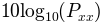for decibels, though Pxx itself is returned.

References:
Bendat & Piersol – Random Data: Analysis and Measurement Procedures, John Wiley & Sons (1986)

kwargs control the `Line2D` properties:

Property Description
`agg_filter` unknown
`alpha` float (0.0 transparent through 1.0 opaque)
`animated` [True | False]
`antialiased` or aa [True | False]
`axes` an `Axes` instance
`clip_box` a `matplotlib.transforms.Bbox` instance
`clip_on` [True | False]
`clip_path` [ (`Path`, `Transform`) | `Patch` | None ]
`color` or c any matplotlib color
`contains` a callable function
`dash_capstyle` [‘butt’ | ‘round’ | ‘projecting’]
`dash_joinstyle` [‘miter’ | ‘round’ | ‘bevel’]
`dashes` sequence of on/off ink in points
`drawstyle` [‘default’ | ‘steps’ | ‘steps-pre’ | ‘steps-mid’ | ‘steps-post’]
`figure` a `matplotlib.figure.Figure` instance
`fillstyle` [‘full’ | ‘left’ | ‘right’ | ‘bottom’ | ‘top’ | ‘none’]
`gid` an id string
`label` string or anything printable with ‘%s’ conversion.
`linestyle` or ls [`'-'` | `'--'` | `'-.'` | `':'` | `'None'` | `' '` | `''`]
`linewidth` or lw float value in points
`lod` [True | False]
`marker` unknown
`markeredgecolor` or mec any matplotlib color
`markeredgewidth` or mew float value in points
`markerfacecolor` or mfc any matplotlib color
`markerfacecoloralt` or mfcalt any matplotlib color
`markersize` or ms float
`markevery` unknown
`path_effects` unknown
`picker` float distance in points or callable pick function `fn(artist, event)`
`pickradius` float distance in points
`rasterized` [True | False | None]
`sketch_params` unknown
`snap` unknown
`solid_capstyle` [‘butt’ | ‘round’ | ‘projecting’]
`solid_joinstyle` [‘miter’ | ‘round’ | ‘bevel’]
`transform` a `matplotlib.transforms.Transform` instance
`url` a url string
`visible` [True | False]
`xdata` 1D array
`ydata` 1D array
`zorder` any number

Example: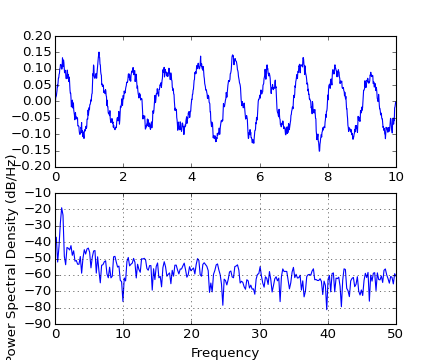`specgram()`
`specgram()` differs in the default overlap; in not returning the mean of the segment periodograms; in returning the times of the segments; and in plotting a colormap instead of a line.
`magnitude_spectrum()`
`magnitude_spectrum()` plots the magnitude spectrum.
`csd()`
`csd()` plots the spectral density between two signals.
`quiver`(*args, **kw)

Plot a 2-D field of arrows.

call signatures:

```quiver(U, V, **kw)
quiver(U, V, C, **kw)
quiver(X, Y, U, V, **kw)
quiver(X, Y, U, V, C, **kw)
```

Arguments:

X, Y:
The x and y coordinates of the arrow locations (default is tail of arrow; see pivot kwarg)
U, V:
Give the x and y components of the arrow vectors
C:
An optional array used to map colors to the arrows

All arguments may be 1-D or 2-D arrays or sequences. If X and Y are absent, they will be generated as a uniform grid. If U and V are 2-D arrays but X and Y are 1-D, and if `len(X)` and `len(Y)` match the column and row dimensions of U, then X and Y will be expanded with `numpy.meshgrid()`.

U, V, C may be masked arrays, but masked X, Y are not supported at present.

Keyword arguments:

units: [ ‘width’ | ‘height’ | ‘dots’ | ‘inches’ | ‘x’ | ‘y’ | ‘xy’ ]

Arrow units; the arrow dimensions except for length are in multiples of this unit.

• ‘width’ or ‘height’: the width or height of the axes
• ‘dots’ or ‘inches’: pixels or inches, based on the figure dpi
• ‘x’, ‘y’, or ‘xy’: X, Y, or sqrt(X^2+Y^2) data units

The arrows scale differently depending on the units. For ‘x’ or ‘y’, the arrows get larger as one zooms in; for other units, the arrow size is independent of the zoom state. For ‘width or ‘height’, the arrow size increases with the width and height of the axes, respectively, when the the window is resized; for ‘dots’ or ‘inches’, resizing does not change the arrows.

angles: [ ‘uv’ | ‘xy’ | array ]
With the default ‘uv’, the arrow aspect ratio is 1, so that if U*==*V the angle of the arrow on the plot is 45 degrees CCW from the x-axis. With ‘xy’, the arrow points from (x,y) to (x+u, y+v). Alternatively, arbitrary angles may be specified as an array of values in degrees, CCW from the x-axis.
scale: [ None | float ]
Data units per arrow length unit, e.g., m/s per plot width; a smaller scale parameter makes the arrow longer. If None, a simple autoscaling algorithm is used, based on the average vector length and the number of vectors. The arrow length unit is given by the scale_units parameter
scale_units: None, or any of the units options.

For example, if scale_units is ‘inches’, scale is 2.0, and `(u,v) = (1,0)`, then the vector will be 0.5 inches long. If scale_units is ‘width’, then the vector will be half the width of the axes.

If scale_units is ‘x’ then the vector will be 0.5 x-axis units. To plot vectors in the x-y plane, with u and v having the same units as x and y, use “angles=’xy’, scale_units=’xy’, scale=1”.

width:
Shaft width in arrow units; default depends on choice of units, above, and number of vectors; a typical starting value is about 0.005 times the width of the plot.
Head width as multiple of shaft width, default is 3
Head length as multiple of shaft width, default is 5
Head length at shaft intersection, default is 4.5
minshaft: scalar
Length below which arrow scales, in units of head length. Do not set this to less than 1, or small arrows will look terrible! Default is 1
minlength: scalar
Minimum length as a multiple of shaft width; if an arrow length is less than this, plot a dot (hexagon) of this diameter instead. Default is 1.
pivot: [ ‘tail’ | ‘middle’ | ‘tip’ ]
The part of the arrow that is at the grid point; the arrow rotates about this point, hence the name pivot.
color: [ color | color sequence ]
This is a synonym for the `PolyCollection` facecolor kwarg. If C has been set, color has no effect.

The defaults give a slightly swept-back arrow; to make the head a triangle, make headaxislength the same as headlength. To make the arrow more pointed, reduce headwidth or increase headlength and headaxislength. To make the head smaller relative to the shaft, scale down all the head parameters. You will probably do best to leave minshaft alone.

linewidths and edgecolors can be used to customize the arrow outlines. Additional `PolyCollection` keyword arguments:

Property Description
`agg_filter` unknown
`alpha` float or None
`animated` [True | False]
`antialiased` or antialiaseds Boolean or sequence of booleans
`array` unknown
`axes` an `Axes` instance
`clim` a length 2 sequence of floats
`clip_box` a `matplotlib.transforms.Bbox` instance
`clip_on` [True | False]
`clip_path` [ (`Path`, `Transform`) | `Patch` | None ]
`cmap` a colormap or registered colormap name
`color` matplotlib color arg or sequence of rgba tuples
`contains` a callable function
`edgecolor` or edgecolors matplotlib color arg or sequence of rgba tuples
`facecolor` or facecolors matplotlib color arg or sequence of rgba tuples
`figure` a `matplotlib.figure.Figure` instance
`gid` an id string
`hatch` [ ‘/’ | ‘\’ | ‘|’ | ‘-‘ | ‘+’ | ‘x’ | ‘o’ | ‘O’ | ‘.’ | ‘*’ ]
`label` string or anything printable with ‘%s’ conversion.
`linestyle` or linestyles or dashes [‘solid’ | ‘dashed’, ‘dashdot’, ‘dotted’ | (offset, on-off-dash-seq) ]
`linewidth` or lw or linewidths float or sequence of floats
`lod` [True | False]
`norm` unknown
`offset_position` unknown
`offsets` float or sequence of floats
`path_effects` unknown
`picker` [None|float|boolean|callable]
`pickradius` unknown
`rasterized` [True | False | None]
`sketch_params` unknown
`snap` unknown
`transform` `Transform` instance
`url` a url string
`urls` unknown
`visible` [True | False]
`zorder` any number
`quiverkey`(*args, **kw)

Add a key to a quiver plot.

Call signature:

```quiverkey(Q, X, Y, U, label, **kw)
```

Arguments:

Q:
The Quiver instance returned by a call to quiver.
X, Y:
The location of the key; additional explanation follows.
U:
The length of the key
label:
A string with the length and units of the key

Keyword arguments:

coordinates = [ ‘axes’ | ‘figure’ | ‘data’ | ‘inches’ ]
Coordinate system and units for X, Y: ‘axes’ and ‘figure’ are normalized coordinate systems with 0,0 in the lower left and 1,1 in the upper right; ‘data’ are the axes data coordinates (used for the locations of the vectors in the quiver plot itself); ‘inches’ is position in the figure in inches, with 0,0 at the lower left corner.
color:
overrides face and edge colors from Q.
labelpos = [ ‘N’ | ‘S’ | ‘E’ | ‘W’ ]
Position the label above, below, to the right, to the left of the arrow, respectively.
labelsep:
Distance in inches between the arrow and the label. Default is 0.1
labelcolor:
defaults to default `Text` color.
fontproperties:
A dictionary with keyword arguments accepted by the `FontProperties` initializer: family, style, variant, size, weight

Any additional keyword arguments are used to override vector properties taken from Q.

The positioning of the key depends on X, Y, coordinates, and labelpos. If labelpos is ‘N’ or ‘S’, X, Y give the position of the middle of the key arrow. If labelpos is ‘E’, X, Y positions the head, and if labelpos is ‘W’, X, Y positions the tail; in either of these two cases, X, Y is somewhere in the middle of the arrow+label key object.

`redraw_in_frame`()

This method can only be used after an initial draw which caches the renderer. It is used to efficiently update Axes data (axis ticks, labels, etc are not updated)

`relim`(visible_only=False)

Recompute the data limits based on current artists. If you want to exclude invisible artists from the calculation, set `visible_only=True`

At present, `Collection` instances are not supported.

`remove`()

Remove the artist from the figure if possible. The effect will not be visible until the figure is redrawn, e.g., with `matplotlib.axes.Axes.draw_idle()`. Call `matplotlib.axes.Axes.relim()` to update the axes limits if desired.

Note: `relim()` will not see collections even if the collection was added to axes with autolim = True.

Note: there is no support for removing the artist’s legend entry.

`remove_callback`(oid)

Remove a callback based on its id.

`add_callback()`
`reset_position`()

Make the original position the active position

`scatter`(x, y, s=20, c=u'b', marker=u'o', cmap=None, norm=None, vmin=None, vmax=None, alpha=None, linewidths=None, verts=None, **kwargs)

Make a scatter plot of x vs y, where x and y are sequence like objects of the same lengths.

Parameters: Returns: x, y : array_like, shape (n, ) Input data s : scalar or array_like, shape (n, ), optional, default: 20 size in points^2. c : color or sequence of color, optional, default `c` can be a single color format string, or a sequence of color specifications of length `N`, or a sequence of `N` numbers to be mapped to colors using the `cmap` and `norm` specified via kwargs (see below). Note that `c` should not be a single numeric RGB or RGBA sequence because that is indistinguishable from an array of values to be colormapped. `c` can be a 2-D array in which the rows are RGB or RGBA, however. marker : `MarkerStyle`, optional, default: ‘o’ See `markers` for more information on the different styles of markers scatter supports. cmap : `Colormap`, optional, default: None A `Colormap` instance or registered name. `cmap` is only used if `c` is an array of floats. If None, defaults to rc `image.cmap`. norm : `Normalize`, optional, default: None A `Normalize` instance is used to scale luminance data to 0, 1. `norm` is only used if `c` is an array of floats. If `None`, use the default `normalize()`. vmin, vmax : scalar, optional, default: None `vmin` and `vmax` are used in conjunction with `norm` to normalize luminance data. If either are `None`, the min and max of the color array is used. Note if you pass a `norm` instance, your settings for `vmin` and `vmax` will be ignored. alpha : scalar, optional, default: None The alpha blending value, between 0 (transparent) and 1 (opaque) linewidths : scalar or array_like, optional, default: None If None, defaults to (lines.linewidth,). Note that this is a tuple, and if you set the linewidths argument you must set it as a sequence of floats, as required by `RegularPolyCollection`. paths : `PathCollection` kwargs : `Collection` properties

Notes

Any or all of `x`, `y`, `s`, and `c` may be masked arrays, in which case all masks will be combined and only unmasked points will be plotted.

Examples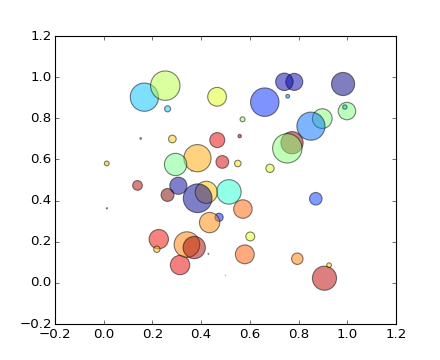`semilogx`(*args, **kwargs)

Make a plot with log scaling on the x axis.

Call signature:

```semilogx(*args, **kwargs)
```

`semilogx()` supports all the keyword arguments of `plot()` and `matplotlib.axes.Axes.set_xscale()`.

Notable keyword arguments:

basex: scalar > 1
Base of the x logarithm
subsx: [ None | sequence ]
The location of the minor xticks; None defaults to autosubs, which depend on the number of decades in the plot; see `set_xscale()` for details.
nonposx: [ ‘mask’ | ‘clip’ ]
Non-positive values in x can be masked as invalid, or clipped to a very small positive number

The remaining valid kwargs are `Line2D` properties:

Property Description
`agg_filter` unknown
`alpha` float (0.0 transparent through 1.0 opaque)
`animated` [True | False]
`antialiased` or aa [True | False]
`axes` an `Axes` instance
`clip_box` a `matplotlib.transforms.Bbox` instance
`clip_on` [True | False]
`clip_path` [ (`Path`, `Transform`) | `Patch` | None ]
`color` or c any matplotlib color
`contains` a callable function
`dash_capstyle` [‘butt’ | ‘round’ | ‘projecting’]
`dash_joinstyle` [‘miter’ | ‘round’ | ‘bevel’]
`dashes` sequence of on/off ink in points
`drawstyle` [‘default’ | ‘steps’ | ‘steps-pre’ | ‘steps-mid’ | ‘steps-post’]
`figure` a `matplotlib.figure.Figure` instance
`fillstyle` [‘full’ | ‘left’ | ‘right’ | ‘bottom’ | ‘top’ | ‘none’]
`gid` an id string
`label` string or anything printable with ‘%s’ conversion.
`linestyle` or ls [`'-'` | `'--'` | `'-.'` | `':'` | `'None'` | `' '` | `''`]
`linewidth` or lw float value in points
`lod` [True | False]
`marker` unknown
`markeredgecolor` or mec any matplotlib color
`markeredgewidth` or mew float value in points
`markerfacecolor` or mfc any matplotlib color
`markerfacecoloralt` or mfcalt any matplotlib color
`markersize` or ms float
`markevery` unknown
`path_effects` unknown
`picker` float distance in points or callable pick function `fn(artist, event)`
`pickradius` float distance in points
`rasterized` [True | False | None]
`sketch_params` unknown
`snap` unknown
`solid_capstyle` [‘butt’ | ‘round’ | ‘projecting’]
`solid_joinstyle` [‘miter’ | ‘round’ | ‘bevel’]
`transform` a `matplotlib.transforms.Transform` instance
`url` a url string
`visible` [True | False]
`xdata` 1D array
`ydata` 1D array
`zorder` any number

`loglog()`
For example code and figure
`semilogy`(*args, **kwargs)

Make a plot with log scaling on the y axis.

call signature:

```semilogy(*args, **kwargs)
```

`semilogy()` supports all the keyword arguments of `plot()` and `matplotlib.axes.Axes.set_yscale()`.

Notable keyword arguments:

basey: scalar > 1
Base of the y logarithm
subsy: [ None | sequence ]
The location of the minor yticks; None defaults to autosubs, which depend on the number of decades in the plot; see `set_yscale()` for details.
nonposy: [ ‘mask’ | ‘clip’ ]
Non-positive values in y can be masked as invalid, or clipped to a very small positive number

The remaining valid kwargs are `Line2D` properties:

Property Description
`agg_filter` unknown
`alpha` float (0.0 transparent through 1.0 opaque)
`animated` [True | False]
`antialiased` or aa [True | False]
`axes` an `Axes` instance
`clip_box` a `matplotlib.transforms.Bbox` instance
`clip_on` [True | False]
`clip_path` [ (`Path`, `Transform`) | `Patch` | None ]
`color` or c any matplotlib color
`contains` a callable function
`dash_capstyle` [‘butt’ | ‘round’ | ‘projecting’]
`dash_joinstyle` [‘miter’ | ‘round’ | ‘bevel’]
`dashes` sequence of on/off ink in points
`drawstyle` [‘default’ | ‘steps’ | ‘steps-pre’ | ‘steps-mid’ | ‘steps-post’]
`figure` a `matplotlib.figure.Figure` instance
`fillstyle` [‘full’ | ‘left’ | ‘right’ | ‘bottom’ | ‘top’ | ‘none’]
`gid` an id string
`label` string or anything printable with ‘%s’ conversion.
`linestyle` or ls [`'-'` | `'--'` | `'-.'` | `':'` | `'None'` | `' '` | `''`]
`linewidth` or lw float value in points
`lod` [True | False]
`marker` unknown
`markeredgecolor` or mec any matplotlib color
`markeredgewidth` or mew float value in points
`markerfacecolor` or mfc any matplotlib color
`markerfacecoloralt` or mfcalt any matplotlib color
`markersize` or ms float
`markevery` unknown
`path_effects` unknown
`picker` float distance in points or callable pick function `fn(artist, event)`
`pickradius` float distance in points
`rasterized` [True | False | None]
`sketch_params` unknown
`snap` unknown
`solid_capstyle` [‘butt’ | ‘round’ | ‘projecting’]
`solid_joinstyle` [‘miter’ | ‘round’ | ‘bevel’]
`transform` a `matplotlib.transforms.Transform` instance
`url` a url string
`visible` [True | False]
`xdata` 1D array
`ydata` 1D array
`zorder` any number

`loglog()`
For example code and figure
`set`(**kwargs)

A tkstyle set command, pass kwargs to set properties

`set_adjustable`(adjustable)

ACCEPTS: [ ‘box’ | ‘datalim’ | ‘box-forced’]

`set_agg_filter`(filter_func)

set agg_filter fuction.

`set_alpha`(alpha)

Set the alpha value used for blending - not supported on all backends.

ACCEPTS: float (0.0 transparent through 1.0 opaque)

`set_anchor`(anchor)

anchor

value description
‘C’ Center
‘SW’ bottom left
‘S’ bottom
‘SE’ bottom right
‘E’ right
‘NE’ top right
‘N’ top
‘NW’ top left
‘W’ left
`set_animated`(b)

Set the artist’s animation state.

ACCEPTS: [True | False]

`set_aspect`(aspect, adjustable=None, anchor=None)

aspect

value description
‘auto’ automatic; fill position rectangle with data
‘normal’ same as ‘auto’; deprecated
‘equal’ same scaling from data to plot units for x and y
num a circle will be stretched such that the height is num times the width. aspect=1 is the same as aspect=’equal’.

value description
‘box’ change physical size of axes
‘datalim’ change xlim or ylim
‘box-forced’ same as ‘box’, but axes can be shared

‘box’ does not allow axes sharing, as this can cause unintended side effect. For cases when sharing axes is fine, use ‘box-forced’.

anchor

value description
‘C’ centered
‘SW’ lower left corner
‘S’ middle of bottom edge
‘SE’ lower right corner
etc.

Deprecated since version 1.2: the option ‘normal’ for aspect is deprecated. Use ‘auto’ instead.

`set_autoscale_on`(b)

Set whether autoscaling is applied on plot commands

accepts: [ True | False ]

`set_autoscalex_on`(b)

Set whether autoscaling for the x-axis is applied on plot commands

accepts: [ True | False ]

`set_autoscaley_on`(b)

Set whether autoscaling for the y-axis is applied on plot commands

accepts: [ True | False ]

`set_axes`(axes)

Set the `Axes` instance in which the artist resides, if any.

ACCEPTS: an `Axes` instance

`set_axes_locator`(locator)

set axes_locator

ACCEPT: a callable object which takes an axes instance and renderer and
returns a bbox.
`set_axis_bgcolor`(color)

set the axes background color

ACCEPTS: any matplotlib color - see `colors()`

`set_axis_off`()

turn off the axis

`set_axis_on`()

turn on the axis

`set_axisbelow`(b)

Set whether the axis ticks and gridlines are above or below most artists

ACCEPTS: [ True | False ]

`set_clip_box`(clipbox)

Set the artist’s clip `Bbox`.

ACCEPTS: a `matplotlib.transforms.Bbox` instance

`set_clip_on`(b)

Set whether artist uses clipping.

When False artists will be visible out side of the axes which can lead to unexpected results.

ACCEPTS: [True | False]

`set_clip_path`(path, transform=None)

Set the artist’s clip path, which may be:

For efficiency, if the path happens to be an axis-aligned rectangle, this method will set the clipping box to the corresponding rectangle and set the clipping path to None.

ACCEPTS: [ (`Path`, `Transform`) | `Patch` | None ]

`set_color_cycle`(clist)

Set the color cycle for any future plot commands on this Axes.

clist is a list of mpl color specifiers.

`set_contains`(picker)

Replace the contains test used by this artist. The new picker should be a callable function which determines whether the artist is hit by the mouse event:

```hit, props = picker(artist, mouseevent)
```

If the mouse event is over the artist, return hit = True and props is a dictionary of properties you want returned with the contains test.

ACCEPTS: a callable function

`set_cursor_props`(*args)

Set the cursor property as:

```ax.set_cursor_props(linewidth, color)
```

or:

```ax.set_cursor_props((linewidth, color))
```

ACCEPTS: a (float, color) tuple

`set_figure`(fig)

Set the class:`Axes` figure

accepts a class:`Figure` instance

`set_frame_on`(b)

Set whether the axes rectangle patch is drawn

ACCEPTS: [ True | False ]

`set_gid`(gid)

Sets the (group) id for the artist

ACCEPTS: an id string

`set_label`(s)

Set the label to s for auto legend.

ACCEPTS: string or anything printable with ‘%s’ conversion.

`set_lod`(on)

Set Level of Detail on or off. If on, the artists may examine things like the pixel width of the axes and draw a subset of their contents accordingly

ACCEPTS: [True | False]

`set_navigate`(b)

Set whether the axes responds to navigation toolbar commands

ACCEPTS: [ True | False ]

`set_navigate_mode`(b)

Set the navigation toolbar button status;

Warning

this is not a user-API function.

`set_path_effects`(path_effects)

set path_effects, which should be a list of instances of matplotlib.patheffect._Base class or its derivatives.

`set_picker`(picker)

Set the epsilon for picking used by this artist

picker can be one of the following:

• None: picking is disabled for this artist (default)

• A boolean: if True then picking will be enabled and the artist will fire a pick event if the mouse event is over the artist

• A float: if picker is a number it is interpreted as an epsilon tolerance in points and the artist will fire off an event if it’s data is within epsilon of the mouse event. For some artists like lines and patch collections, the artist may provide additional data to the pick event that is generated, e.g., the indices of the data within epsilon of the pick event

• A function: if picker is callable, it is a user supplied function which determines whether the artist is hit by the mouse event:

```hit, props = picker(artist, mouseevent)
```

to determine the hit test. if the mouse event is over the artist, return hit=True and props is a dictionary of properties you want added to the PickEvent attributes.

ACCEPTS: [None|float|boolean|callable]

`set_position`(pos, which=u'both')

Set the axes position with:

```pos = [left, bottom, width, height]
```

in relative 0,1 coords, or pos can be a `Bbox`

There are two position variables: one which is ultimately used, but which may be modified by `apply_aspect()`, and a second which is the starting point for `apply_aspect()`.

Optional keyword arguments:

which

value description
‘active’ to change the first
‘original’ to change the second
‘both’ to change both
`set_rasterization_zorder`(z)

Set zorder value below which artists will be rasterized. Set to `None` to disable rasterizing of artists below a particular zorder.

`set_rasterized`(rasterized)

Force rasterized (bitmap) drawing in vector backend output.

Defaults to None, which implies the backend’s default behavior

ACCEPTS: [True | False | None]

`set_sketch_params`(scale=None, length=None, randomness=None)

Sets the the sketch parameters.

Parameters: scale : float, optional The amplitude of the wiggle perpendicular to the source line, in pixels. If scale is `None`, or not provided, no sketch filter will be provided. length : float, optional The length of the wiggle along the line, in pixels (default 128.0) randomness : float, optional The scale factor by which the length is shrunken or expanded (default 16.0)
`set_snap`(snap)

Sets the snap setting which may be:

• True: snap vertices to the nearest pixel center
• False: leave vertices as-is
• None: (auto) If the path contains only rectilinear line segments, round to the nearest pixel center

Only supported by the Agg and MacOSX backends.

`set_title`(label, fontdict=None, loc=u'center', **kwargs)

Set a title for the axes.

Set one of the three available axes titles. The available titles are positioned above the axes in the center, flush with the left edge, and flush with the right edge.

Parameters: Returns: label : str Text to use for the title fontdict : dict A dictionary controlling the appearance of the title text, the default `fontdict` is: ```{'fontsize': rcParams['axes.titlesize'], 'fontweight' : rcParams['axes.titleweight'], 'verticalalignment': 'baseline', 'horizontalalignment': loc} ``` loc : {‘center’, ‘left’, ‘right’}, str, optional Which title to set, defaults to ‘center’ text : `Text` The matplotlib text instance representing the title kwargs : text properties Other keyword arguments are text properties, see `Text` for a list of valid text properties.
`set_transform`(t)

Set the `Transform` instance used by this artist.

ACCEPTS: `Transform` instance

`set_url`(url)

Sets the url for the artist

ACCEPTS: a url string

`set_visible`(b)

Set the artist’s visiblity.

ACCEPTS: [True | False]

`set_xbound`(lower=None, upper=None)

Set the lower and upper numerical bounds of the x-axis. This method will honor axes inversion regardless of parameter order. It will not change the _autoscaleXon attribute.

`set_xlabel`(xlabel, fontdict=None, labelpad=None, **kwargs)

Set the label for the xaxis.

Parameters: Other Parameters: xlabel : string x label labelpad : scalar, optional, default: None spacing in points between the label and the x-axis kwargs : `Text` properties

`text`
for information on how override and the optional args work
`set_xlim`(left=None, right=None, emit=True, auto=False, **kw)

Call signature:

```set_xlim(self, *args, **kwargs):
```

Set the data limits for the xaxis

Examples:

```set_xlim((left, right))
set_xlim(left, right)
set_xlim(left=1) # right unchanged
set_xlim(right=1) # left unchanged
```

Keyword arguments:

left: scalar
The left xlim; xmin, the previous name, may still be used
right: scalar
The right xlim; xmax, the previous name, may still be used
emit: [ True | False ]
Notify observers of limit change
auto: [ True | False | None ]
Turn x autoscaling on (True), off (False; default), or leave unchanged (None)

Note, the left (formerly xmin) value may be greater than the right (formerly xmax). For example, suppose x is years before present. Then one might use:

```set_ylim(5000, 0)
```

so 5000 years ago is on the left of the plot and the present is on the right.

Returns the current xlimits as a length 2 tuple

ACCEPTS: length 2 sequence of floats

`set_xmargin`(m)

Set padding of X data limits prior to autoscaling.

m times the data interval will be added to each end of that interval before it is used in autoscaling.

accepts: float in range 0 to 1

`set_xscale`(value, **kwargs)

Call signature:

```set_xscale(value)
```

Set the scaling of the x-axis: u’linear’ | u’log’ | u’symlog’

ACCEPTS: [u’linear’ | u’log’ | u’symlog’]

Different kwargs are accepted, depending on the scale:

‘linear’

‘log’

basex/basey:
The base of the logarithm
non-positive values in x or y can be masked as invalid, or clipped to a very small positive number
subsx/subsy:

Where to place the subticks between each major tick. Should be a sequence of integers. For example, in a log10 scale: `[2, 3, 4, 5, 6, 7, 8, 9]`

will place 8 logarithmically spaced minor ticks between each major tick.

‘symlog’

basex/basey:
The base of the logarithm
linthreshx/linthreshy:
The range (-x, x) within which the plot is linear (to avoid having the plot go to infinity around zero).
subsx/subsy:

Where to place the subticks between each major tick. Should be a sequence of integers. For example, in a log10 scale: `[2, 3, 4, 5, 6, 7, 8, 9]`

will place 8 logarithmically spaced minor ticks between each major tick.

linscalex/linscaley:
This allows the linear range (-linthresh to linthresh) to be stretched relative to the logarithmic range. Its value is the number of decades to use for each half of the linear range. For example, when linscale == 1.0 (the default), the space used for the positive and negative halves of the linear range will be equal to one decade in the logarithmic range.
`set_xticklabels`(labels, fontdict=None, minor=False, **kwargs)

Call signature:

```set_xticklabels(labels, fontdict=None, minor=False, **kwargs)
```

Set the xtick labels with list of strings labels. Return a list of axis text instances.

kwargs set the `Text` properties. Valid properties are

Property Description
`agg_filter` unknown
`alpha` float (0.0 transparent through 1.0 opaque)
`animated` [True | False]
`axes` an `Axes` instance
`backgroundcolor` any matplotlib color
`bbox` rectangle prop dict
`clip_box` a `matplotlib.transforms.Bbox` instance
`clip_on` [True | False]
`clip_path` [ (`Path`, `Transform`) | `Patch` | None ]
`color` any matplotlib color
`contains` a callable function
`family` or fontfamily or fontname or name [FONTNAME | ‘serif’ | ‘sans-serif’ | ‘cursive’ | ‘fantasy’ | ‘monospace’ ]
`figure` a `matplotlib.figure.Figure` instance
`fontproperties` or font_properties a `matplotlib.font_manager.FontProperties` instance
`gid` an id string
`horizontalalignment` or ha [ ‘center’ | ‘right’ | ‘left’ ]
`label` string or anything printable with ‘%s’ conversion.
`linespacing` float (multiple of font size)
`lod` [True | False]
`multialignment` [‘left’ | ‘right’ | ‘center’ ]
`path_effects` unknown
`picker` [None|float|boolean|callable]
`position` (x,y)
`rasterized` [True | False | None]
`rotation` [ angle in degrees | ‘vertical’ | ‘horizontal’ ]
`rotation_mode` unknown
`size` or fontsize [size in points | ‘xx-small’ | ‘x-small’ | ‘small’ | ‘medium’ | ‘large’ | ‘x-large’ | ‘xx-large’ ]
`sketch_params` unknown
`snap` unknown
`stretch` or fontstretch [a numeric value in range 0-1000 | ‘ultra-condensed’ | ‘extra-condensed’ | ‘condensed’ | ‘semi-condensed’ | ‘normal’ | ‘semi-expanded’ | ‘expanded’ | ‘extra-expanded’ | ‘ultra-expanded’ ]
`style` or fontstyle [ ‘normal’ | ‘italic’ | ‘oblique’]
`text` string or anything printable with ‘%s’ conversion.
`transform` `Transform` instance
`url` a url string
`variant` or fontvariant [ ‘normal’ | ‘small-caps’ ]
`verticalalignment` or va or ma [ ‘center’ | ‘top’ | ‘bottom’ | ‘baseline’ ]
`visible` [True | False]
`weight` or fontweight [a numeric value in range 0-1000 | ‘ultralight’ | ‘light’ | ‘normal’ | ‘regular’ | ‘book’ | ‘medium’ | ‘roman’ | ‘semibold’ | ‘demibold’ | ‘demi’ | ‘bold’ | ‘heavy’ | ‘extra bold’ | ‘black’ ]
`x` float
`y` float
`zorder` any number

ACCEPTS: sequence of strings

`set_xticks`(ticks, minor=False)

Set the x ticks with list of ticks

ACCEPTS: sequence of floats

`set_ybound`(lower=None, upper=None)

Set the lower and upper numerical bounds of the y-axis. This method will honor axes inversion regardless of parameter order. It will not change the _autoscaleYon attribute.

`set_ylabel`(ylabel, fontdict=None, labelpad=None, **kwargs)

Set the label for the yaxis

Parameters: Other Parameters: ylabel : string y label labelpad : scalar, optional, default: None spacing in points between the label and the x-axis kwargs : `Text` properties

`text`
for information on how override and the optional args work
`set_ylim`(bottom=None, top=None, emit=True, auto=False, **kw)

Call signature:

```set_ylim(self, *args, **kwargs):
```

Set the data limits for the yaxis

Examples:

```set_ylim((bottom, top))
set_ylim(bottom, top)
set_ylim(bottom=1) # top unchanged
set_ylim(top=1) # bottom unchanged
```

Keyword arguments:

bottom: scalar
The bottom ylim; the previous name, ymin, may still be used
top: scalar
The top ylim; the previous name, ymax, may still be used
emit: [ True | False ]
Notify observers of limit change
auto: [ True | False | None ]
Turn y autoscaling on (True), off (False; default), or leave unchanged (None)

Note, the bottom (formerly ymin) value may be greater than the top (formerly ymax). For example, suppose y is depth in the ocean. Then one might use:

```set_ylim(5000, 0)
```

so 5000 m depth is at the bottom of the plot and the surface, 0 m, is at the top.

Returns the current ylimits as a length 2 tuple

ACCEPTS: length 2 sequence of floats

`set_ymargin`(m)

Set padding of Y data limits prior to autoscaling.

m times the data interval will be added to each end of that interval before it is used in autoscaling.

accepts: float in range 0 to 1

`set_yscale`(value, **kwargs)

Call signature:

```set_yscale(value)
```

Set the scaling of the y-axis: u’linear’ | u’log’ | u’symlog’

ACCEPTS: [u’linear’ | u’log’ | u’symlog’]

Different kwargs are accepted, depending on the scale:

‘linear’

‘log’

basex/basey:
The base of the logarithm
non-positive values in x or y can be masked as invalid, or clipped to a very small positive number
subsx/subsy:

Where to place the subticks between each major tick. Should be a sequence of integers. For example, in a log10 scale: `[2, 3, 4, 5, 6, 7, 8, 9]`

will place 8 logarithmically spaced minor ticks between each major tick.

‘symlog’

basex/basey:
The base of the logarithm
linthreshx/linthreshy:
The range (-x, x) within which the plot is linear (to avoid having the plot go to infinity around zero).
subsx/subsy:

Where to place the subticks between each major tick. Should be a sequence of integers. For example, in a log10 scale: `[2, 3, 4, 5, 6, 7, 8, 9]`

will place 8 logarithmically spaced minor ticks between each major tick.

linscalex/linscaley:
This allows the linear range (-linthresh to linthresh) to be stretched relative to the logarithmic range. Its value is the number of decades to use for each half of the linear range. For example, when linscale == 1.0 (the default), the space used for the positive and negative halves of the linear range will be equal to one decade in the logarithmic range.
`set_yticklabels`(labels, fontdict=None, minor=False, **kwargs)

Call signature:

```set_yticklabels(labels, fontdict=None, minor=False, **kwargs)
```

Set the y tick labels with list of strings labels. Return a list of `Text` instances.

kwargs set `Text` properties for the labels. Valid properties are

Property Description
`agg_filter` unknown
`alpha` float (0.0 transparent through 1.0 opaque)
`animated` [True | False]
`axes` an `Axes` instance
`backgroundcolor` any matplotlib color
`bbox` rectangle prop dict
`clip_box` a `matplotlib.transforms.Bbox` instance
`clip_on` [True | False]
`clip_path` [ (`Path`, `Transform`) | `Patch` | None ]
`color` any matplotlib color
`contains` a callable function
`family` or fontfamily or fontname or name [FONTNAME | ‘serif’ | ‘sans-serif’ | ‘cursive’ | ‘fantasy’ | ‘monospace’ ]
`figure` a `matplotlib.figure.Figure` instance
`fontproperties` or font_properties a `matplotlib.font_manager.FontProperties` instance
`gid` an id string
`horizontalalignment` or ha [ ‘center’ | ‘right’ | ‘left’ ]
`label` string or anything printable with ‘%s’ conversion.
`linespacing` float (multiple of font size)
`lod` [True | False]
`multialignment` [‘left’ | ‘right’ | ‘center’ ]
`path_effects` unknown
`picker` [None|float|boolean|callable]
`position` (x,y)
`rasterized` [True | False | None]
`rotation` [ angle in degrees | ‘vertical’ | ‘horizontal’ ]
`rotation_mode` unknown
`size` or fontsize [size in points | ‘xx-small’ | ‘x-small’ | ‘small’ | ‘medium’ | ‘large’ | ‘x-large’ | ‘xx-large’ ]
`sketch_params` unknown
`snap` unknown
`stretch` or fontstretch [a numeric value in range 0-1000 | ‘ultra-condensed’ | ‘extra-condensed’ | ‘condensed’ | ‘semi-condensed’ | ‘normal’ | ‘semi-expanded’ | ‘expanded’ | ‘extra-expanded’ | ‘ultra-expanded’ ]
`style` or fontstyle [ ‘normal’ | ‘italic’ | ‘oblique’]
`text` string or anything printable with ‘%s’ conversion.
`transform` `Transform` instance
`url` a url string
`variant` or fontvariant [ ‘normal’ | ‘small-caps’ ]
`verticalalignment` or va or ma [ ‘center’ | ‘top’ | ‘bottom’ | ‘baseline’ ]
`visible` [True | False]
`weight` or fontweight [a numeric value in range 0-1000 | ‘ultralight’ | ‘light’ | ‘normal’ | ‘regular’ | ‘book’ | ‘medium’ | ‘roman’ | ‘semibold’ | ‘demibold’ | ‘demi’ | ‘bold’ | ‘heavy’ | ‘extra bold’ | ‘black’ ]
`x` float
`y` float
`zorder` any number

ACCEPTS: sequence of strings

`set_yticks`(ticks, minor=False)

Set the y ticks with list of ticks

ACCEPTS: sequence of floats

Keyword arguments:

minor: [ False | True ]
Sets the minor ticks if True
`set_zorder`(level)

Set the zorder for the artist. Artists with lower zorder values are drawn first.

ACCEPTS: any number

`specgram`(x, NFFT=None, Fs=None, Fc=None, detrend=None, window=None, noverlap=None, cmap=None, xextent=None, pad_to=None, sides=None, scale_by_freq=None, mode=None, scale=None, vmin=None, vmax=None, **kwargs)

Plot a spectrogram.

Call signature:

```specgram(x, NFFT=256, Fs=2, Fc=0, detrend=mlab.detrend_none,
window=mlab.window_hanning, noverlap=128,
scale_by_freq=None, mode='default', scale='default',
**kwargs)
```

Compute and plot a spectrogram of data in x. Data are split into NFFT length segments and the spectrum of each section is computed. The windowing function window is applied to each segment, and the amount of overlap of each segment is specified with noverlap. The spectrogram is plotted as a colormap (using imshow).

x: 1-D array or sequence
Array or sequence containing the data

Keyword arguments:

Fs: scalar
The sampling frequency (samples per time unit). It is used to calculate the Fourier frequencies, freqs, in cycles per time unit. The default value is 2.
window: callable or ndarray
A function or a vector of length NFFT. To create window vectors see `window_hanning()`, `window_none()`, `numpy.blackman()`, `numpy.hamming()`, `numpy.bartlett()`, `scipy.signal()`, `scipy.signal.get_window()`, etc. The default is `window_hanning()`. If a function is passed as the argument, it must take a data segment as an argument and return the windowed version of the segment.
sides: [ ‘default’ | ‘onesided’ | ‘twosided’ ]
Specifies which sides of the spectrum to return. Default gives the default behavior, which returns one-sided for real data and both for complex data. ‘onesided’ forces the return of a one-sided spectrum, while ‘twosided’ forces two-sided.
The number of points to which the data segment is padded when performing the FFT. This can be different from NFFT, which specifies the number of data points used. While not increasing the actual resolution of the spectrum (the minimum distance between resolvable peaks), this can give more points in the plot, allowing for more detail. This corresponds to the n parameter in the call to fft(). The default is None, which sets pad_to equal to NFFT
NFFT: integer
The number of data points used in each block for the FFT. A power 2 is most efficient. The default value is 256. This should NOT be used to get zero padding, or the scaling of the result will be incorrect. Use pad_to for this instead.
detrend: [ ‘default’ | ‘constant’ | ‘mean’ | ‘linear’ | ‘none’] or
callable

The function applied to each segment before fft-ing, designed to remove the mean or linear trend. Unlike in MATLAB, where the detrend parameter is a vector, in matplotlib is it a function. The `pylab` module defines `detrend_none()`, `detrend_mean()`, and `detrend_linear()`, but you can use a custom function as well. You can also use a string to choose one of the functions. ‘default’, ‘constant’, and ‘mean’ call `detrend_mean()`. ‘linear’ calls `detrend_linear()`. ‘none’ calls `detrend_none()`.

scale_by_freq: boolean
Specifies whether the resulting density values should be scaled by the scaling frequency, which gives density in units of Hz^-1. This allows for integration over the returned frequency values. The default is True for MATLAB compatibility.
mode: [ ‘default’ | ‘psd’ | ‘magnitude’ | ‘angle’ | ‘phase’ ]
What sort of spectrum to use. Default is ‘psd’. which takes the power spectral density. ‘complex’ returns the complex-valued frequency spectrum. ‘magnitude’ returns the magnitude spectrum. ‘angle’ returns the phase spectrum without unwrapping. ‘phase’ returns the phase spectrum with unwrapping.
noverlap: integer
The number of points of overlap between blocks. The default value is 128.
scale: [ ‘default’ | ‘linear’ | ‘dB’ ]
The scaling of the values in the spec. ‘linear’ is no scaling. ‘dB’ returns the values in dB scale. When mode is ‘psd’, this is dB power (10 * log10). Otherwise this is dB amplitude (20 * log10). ‘default’ is ‘dB’ if mode is ‘psd’ or ‘magnitude’ and ‘linear’ otherwise. This must be ‘linear’ if mode is ‘angle’ or ‘phase’.
Fc: integer
The center frequency of x (defaults to 0), which offsets the x extents of the plot to reflect the frequency range used when a signal is acquired and then filtered and downsampled to baseband.
cmap:
A `matplotlib.colors.Colormap` instance; if None, use default determined by rc
xextent:
The image extent along the x-axis. xextent = (xmin,xmax) The default is (0,max(bins)), where bins is the return value from `specgram()`
kwargs:
Additional kwargs are passed on to imshow which makes the specgram image

Note

detrend and scale_by_freq only apply when mode is set to ‘psd’

Returns the tuple (spectrum, freqs, t, im):

spectrum: 2-D array
columns are the periodograms of successive segments
freqs: 1-D array
The frequencies corresponding to the rows in spectrum
t: 1-D array
The times corresponding to midpoints of segments (i.e the columns in spectrum)
im: instance of class `AxesImage`
The image created by imshow containing the spectrogram

Example: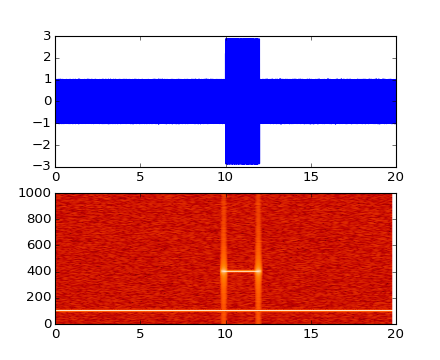`psd()`
`psd()` differs in the default overlap; in returning the mean of the segment periodograms; in not returning times; and in generating a line plot instead of colormap.
`magnitude_spectrum()`
A single spectrum, similar to having a single segment when mode is ‘magnitude’. Plots a line instead of a colormap.
`angle_spectrum()`
A single spectrum, similar to having a single segment when mode is ‘angle’. Plots a line instead of a colormap.
`phase_spectrum()`
A single spectrum, similar to having a single segment when mode is ‘phase’. Plots a line instead of a colormap.
`spy`(Z, precision=0, marker=None, markersize=None, aspect=u'equal', origin=u'upper', **kwargs)

Plot the sparsity pattern on a 2-D array.

`spy(Z)` plots the sparsity pattern of the 2-D array Z.

Parameters: Z : sparse array (n, m) The array to be plotted. precision : float, optional, default: 0 If precision is 0, any non-zero value will be plotted; else, values of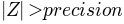will be plotted. For `scipy.sparse.spmatrix` instances, there is a special case: if precision is ‘present’, any value present in the array will be plotted, even if it is identically zero. origin : [“upper”, “lower”], optional, default: “upper” Place the [0,0] index of the array in the upper left or lower left corner of the axes. aspect : [‘auto’ | ‘equal’ | scalar], optional, default: “equal” If ‘equal’, and `extent` is None, changes the axes aspect ratio to match that of the image. If `extent` is not `None`, the axes aspect ratio is changed to match that of the extent. If ‘auto’, changes the image aspect ratio to match that of the axes. If None, default to rc `image.aspect` value. Two plotting styles are available: image or marker. Both : are available for full arrays, but only the marker style : works for :class:`scipy.sparse.spmatrix` instances. : If *marker* and *markersize* are *None*, an image will be : returned and any remaining kwargs are passed to : :func:`~matplotlib.pyplot.imshow`; else, a : :class:`~matplotlib.lines.Line2D` object will be returned with : the value of marker determining the marker type, and any : remaining kwargs passed to the : :meth:`~matplotlib.axes.Axes.plot` method. : If *marker* and *markersize* are *None*, useful kwargs include: : * *cmap* : * *alpha* :

`imshow`
for image options.
`plot`
for plotting options
`stackplot`(x, *args, **kwargs)

Draws a stacked area plot.

x : 1d array of dimension N

y : 2d array of dimension MxN, OR any number 1d arrays each of dimension

1xN. The data is assumed to be unstacked. Each of the following calls is legal:

```stackplot(x, y)               # where y is MxN
stackplot(x, y1, y2, y3, y4)  # where y1, y2, y3, y4, are all 1xNm
```

Keyword arguments:

baseline : [‘zero’, ‘sym’, ‘wiggle’, ‘weighted_wiggle’]
Method used to calculate the baseline. ‘zero’ is just a simple stacked plot. ‘sym’ is symmetric around zero and is sometimes called `ThemeRiver`. ‘wiggle’ minimizes the sum of the squared slopes. ‘weighted_wiggle’ does the same but weights to account for size of each layer. It is also called `Streamgraph`-layout. More details can be found at http://www.leebyron.com/else/streamgraph/.
colors : A list or tuple of colors. These will be cycled through and
used to colour the stacked areas. All other keyword arguments are passed to `fill_between()`

Returns r : A list of `PolyCollection`, one for each element in the stacked area plot.

Note that `Legend` does not support `PolyCollection` objects. To create a legend on a stackplot, use a proxy artist: http://matplotlib.org/users/legend_guide.html#using-proxy-artist

`start_pan`(x, y, button)

Called when a pan operation has started.

x, y are the mouse coordinates in display coords. button is the mouse button number:

• 1: LEFT
• 2: MIDDLE
• 3: RIGHT

Note

Intended to be overridden by new projection types.

`stem`(*args, **kwargs)

Create a stem plot.

Call signatures:

```stem(y, linefmt='b-', markerfmt='bo', basefmt='r-')
stem(x, y, linefmt='b-', markerfmt='bo', basefmt='r-')
```

A stem plot plots vertical lines (using linefmt) at each x location from the baseline to y, and places a marker there using markerfmt. A horizontal line at 0 is is plotted using basefmt.

If no x values are provided, the default is (0, 1, ..., len(y) - 1)

Return value is a tuple (markerline, stemlines, baseline).

This document for details.

Example: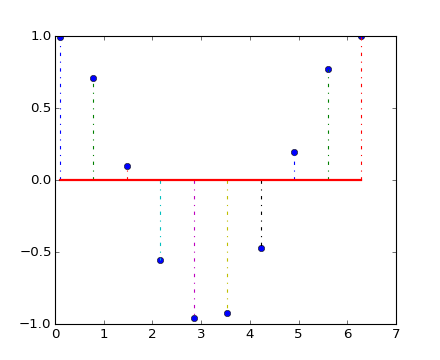`step`(x, y, *args, **kwargs)

Make a step plot.

Call signature:

```step(x, y, *args, **kwargs)
```

Additional keyword args to `step()` are the same as those for `plot()`.

x and y must be 1-D sequences, and it is assumed, but not checked, that x is uniformly increasing.

Keyword arguments:

where: [ ‘pre’ | ‘post’ | ‘mid’ ]

If ‘pre’, the interval from x[i] to x[i+1] has level y[i+1]

If ‘post’, that interval has level y[i]

If ‘mid’, the jumps in y occur half-way between the x-values.

`streamplot`(x, y, u, v, density=1, linewidth=None, color=None, cmap=None, norm=None, arrowsize=1, arrowstyle=u'-|>', minlength=0.1, transform=None, zorder=1)

Draws streamlines of a vector flow.

x, y : 1d arrays
an evenly spaced grid.
u, v : 2d arrays
x and y-velocities. Number of rows should match length of y, and the number of columns should match x.
density : float or 2-tuple
Controls the closeness of streamlines. When `density = 1`, the domain is divided into a 30x30 grid—density linearly scales this grid. Each cell in the grid can have, at most, one traversing streamline. For different densities in each direction, use [density_x, density_y].
linewidth : numeric or 2d array
vary linewidth when given a 2d array with the same shape as velocities.
color : matplotlib color code, or 2d array
Streamline color. When given an array with the same shape as velocities, color values are converted to colors using cmap.
cmap : `Colormap`
Colormap used to plot streamlines and arrows. Only necessary when using an array input for color.
norm : `Normalize`
Normalize object used to scale luminance data to 0, 1. If None, stretch (min, max) to (0, 1). Only necessary when color is an array.
arrowsize : float
Factor scale arrow size.
arrowstyle : str
Arrow style specification. See `FancyArrowPatch`.
minlength : float
Minimum length of streamline in axes coordinates.
zorder : int
any number

Returns:

stream_container : StreamplotSet

Container object with attributes

This container will probably change in the future to allow changes to the colormap, alpha, etc. for both lines and arrows, but these changes should be backward compatible.

`table`(**kwargs)

Add a table to the current axes.

Call signature:

```table(cellText=None, cellColours=None,
cellLoc='right', colWidths=None,
rowLabels=None, rowColours=None, rowLoc='left',
colLabels=None, colColours=None, colLoc='center',
loc='bottom', bbox=None):
```

Returns a `matplotlib.table.Table` instance. For finer grained control over tables, use the `Table` class and add it to the axes with `add_table()`.

Thanks to John Gill for providing the class and table.

kwargs control the `Table` properties:

Property Description
`agg_filter` unknown
`alpha` float (0.0 transparent through 1.0 opaque)
`animated` [True | False]
`axes` an `Axes` instance
`clip_box` a `matplotlib.transforms.Bbox` instance
`clip_on` [True | False]
`clip_path` [ (`Path`, `Transform`) | `Patch` | None ]
`contains` a callable function
`figure` a `matplotlib.figure.Figure` instance
`fontsize` a float in points
`gid` an id string
`label` string or anything printable with ‘%s’ conversion.
`lod` [True | False]
`path_effects` unknown
`picker` [None|float|boolean|callable]
`rasterized` [True | False | None]
`sketch_params` unknown
`snap` unknown
`transform` `Transform` instance
`url` a url string
`visible` [True | False]
`zorder` any number
`text`(x, y, s, fontdict=None, withdash=False, **kwargs)

Add text in string `s` to axis at location `x`, `y`, data coordinates.

Parameters: Other Parameters: x, y : scalars data coordinates s : string text fontdict : dictionary, optional, default: None A dictionary to override the default text properties. If fontdict is None, the defaults are determined by your rc parameters. withdash : boolean, optional, default: False Creates a `TextWithDash` instance instead of a `Text` instance. kwargs : `Text` properties. Other miscellaneous text parameters.

Examples

Individual keyword arguments can be used to override any given parameter:

```>>> text(x, y, s, fontsize=12)
```

The default transform specifies that text is in data coords, alternatively, you can specify text in axis coords (0,0 is lower-left and 1,1 is upper-right). The example below places text in the center of the axes:

```>>> text(0.5, 0.5,'matplotlib', horizontalalignment='center',
...      verticalalignment='center',
...      transform=ax.transAxes)
```

You can put a rectangular box around the text instance (e.g., to set a background color) by using the keyword `bbox`. `bbox` is a dictionary of `Rectangle` properties. For example:

```>>> text(x, y, s, bbox=dict(facecolor='red', alpha=0.5))
```
`tick_params`(axis=u'both', **kwargs)

Change the appearance of ticks and tick labels.

Keyword arguments:

axis : [‘x’ | ‘y’ | ‘both’]
Axis on which to operate; default is ‘both’.
reset : [True | False]
If True, set all parameters to defaults before processing other keyword arguments. Default is False.
which : [‘major’ | ‘minor’ | ‘both’]
Default is ‘major’; apply arguments to which ticks.
direction : [‘in’ | ‘out’ | ‘inout’]
Puts ticks inside the axes, outside the axes, or both.
length
Tick length in points.
width
Tick width in points.
color
Tick color; accepts any mpl color spec.
Distance in points between tick and label.
labelsize
Tick label font size in points or as a string (e.g., ‘large’).
labelcolor
Tick label color; mpl color spec.
colors
Changes the tick color and the label color to the same value: mpl color spec.
zorder
Tick and label zorder.
bottom, top, left, right : [bool | ‘on’ | ‘off’]
controls whether to draw the respective ticks.
labelbottom, labeltop, labelleft, labelright
Boolean or [‘on’ | ‘off’], controls whether to draw the respective tick labels.

Example:

```ax.tick_params(direction='out', length=6, width=2, colors='r')
```

This will make all major ticks be red, pointing out of the box, and with dimensions 6 points by 2 points. Tick labels will also be red.

`ticklabel_format`(**kwargs)

Change the `ScalarFormatter` used by default for linear axes.

Optional keyword arguments:

Keyword Description
style [ ‘sci’ (or ‘scientific’) | ‘plain’ ] plain turns off scientific notation
scilimits (m, n), pair of integers; if style is ‘sci’, scientific notation will be used for numbers outside the range 10`m`:sup: to 10`n`:sup:. Use (0,0) to include all numbers.
useOffset [True | False | offset]; if True, the offset will be calculated as needed; if False, no offset will be used; if a numeric offset is specified, it will be used.
axis [ ‘x’ | ‘y’ | ‘both’ ]
useLocale If True, format the number according to the current locale. This affects things such as the character used for the decimal separator. If False, use C-style (English) formatting. The default setting is controlled by the axes.formatter.use_locale rcparam.

Only the major ticks are affected. If the method is called when the `ScalarFormatter` is not the `Formatter` being used, an `AttributeError` will be raised.

`tricontour`(*args, **kwargs)

Draw contours on an unstructured triangular grid. `tricontour()` and `tricontourf()` draw contour lines and filled contours, respectively. Except as noted, function signatures and return values are the same for both versions.

The triangulation can be specified in one of two ways; either:

```tricontour(triangulation, ...)
```

where triangulation is a `matplotlib.tri.Triangulation` object, or

```tricontour(x, y, ...)
tricontour(x, y, triangles, ...)
tricontour(x, y, triangles=triangles, ...)
```

in which case a Triangulation object will be created. See `Triangulation` for a explanation of these possibilities.

The remaining arguments may be:

```tricontour(..., Z)
```

where Z is the array of values to contour, one per point in the triangulation. The level values are chosen automatically.

```tricontour(..., Z, N)
```

contour N automatically-chosen levels.

```tricontour(..., Z, V)
```

draw contour lines at the values specified in sequence V

```tricontourf(..., Z, V)
```

fill the (len(V)-1) regions between the values in V

```tricontour(Z, **kwargs)
```

Use keyword args to control colors, linewidth, origin, cmap ... see below for more details.

`C = tricontour(...)` returns a `TriContourSet` object.

Optional keyword arguments:

colors: [ None | string | (mpl_colors) ]

If None, the colormap specified by cmap will be used.

If a string, like ‘r’ or ‘red’, all levels will be plotted in this color.

If a tuple of matplotlib color args (string, float, rgb, etc), different levels will be plotted in different colors in the order specified.

alpha: float
The alpha blending value
cmap: [ None | Colormap ]
A cm `Colormap` instance or None. If cmap is None and colors is None, a default Colormap is used.
norm: [ None | Normalize ]
A `matplotlib.colors.Normalize` instance for scaling data values to colors. If norm is None and colors is None, the default linear scaling is used.
levels [level0, level1, ..., leveln]
A list of floating point numbers indicating the level curves to draw; eg to draw just the zero contour pass `levels=`
origin: [ None | ‘upper’ | ‘lower’ | ‘image’ ]

If None, the first value of Z will correspond to the lower left corner, location (0,0). If ‘image’, the rc value for `image.origin` will be used.

This keyword is not active if X and Y are specified in the call to contour.

extent: [ None | (x0,x1,y0,y1) ]

If origin is not None, then extent is interpreted as in `matplotlib.pyplot.imshow()`: it gives the outer pixel boundaries. In this case, the position of Z[0,0] is the center of the pixel, not a corner. If origin is None, then (x0, y0) is the position of Z[0,0], and (x1, y1) is the position of Z[-1,-1].

This keyword is not active if X and Y are specified in the call to contour.

locator: [ None | ticker.Locator subclass ]
If locator is None, the default `MaxNLocator` is used. The locator is used to determine the contour levels if they are not given explicitly via the V argument.
extend: [ ‘neither’ | ‘both’ | ‘min’ | ‘max’ ]
Unless this is ‘neither’, contour levels are automatically added to one or both ends of the range so that all data are included. These added ranges are then mapped to the special colormap values which default to the ends of the colormap range, but can be set via `matplotlib.colors.Colormap.set_under()` and `matplotlib.colors.Colormap.set_over()` methods.
xunits, yunits: [ None | registered units ]
Override axis units by specifying an instance of a `matplotlib.units.ConversionInterface`.

tricontour-only keyword arguments:

linewidths: [ None | number | tuple of numbers ]

If linewidths is None, the default width in `lines.linewidth` in `matplotlibrc` is used.

If a number, all levels will be plotted with this linewidth.

If a tuple, different levels will be plotted with different linewidths in the order specified

linestyles: [ None | ‘solid’ | ‘dashed’ | ‘dashdot’ | ‘dotted’ ]

If linestyles is None, the ‘solid’ is used.

linestyles can also be an iterable of the above strings specifying a set of linestyles to be used. If this iterable is shorter than the number of contour levels it will be repeated as necessary.

If contour is using a monochrome colormap and the contour level is less than 0, then the linestyle specified in `contour.negative_linestyle` in `matplotlibrc` will be used.

tricontourf-only keyword arguments:

antialiased: [ True | False ]
enable antialiasing
nchunk: [ 0 | integer ]
If 0, no subdivision of the domain. Specify a positive integer to divide the domain into subdomains of roughly nchunk by nchunk points. This may never actually be advantageous, so this option may be removed. Chunking introduces artifacts at the chunk boundaries unless antialiased is False.

Note: tricontourf fills intervals that are closed at the top; that is, for boundaries z1 and z2, the filled region is:

```z1 < z <= z2
```

There is one exception: if the lowest boundary coincides with the minimum value of the z array, then that minimum value will be included in the lowest interval.

Examples: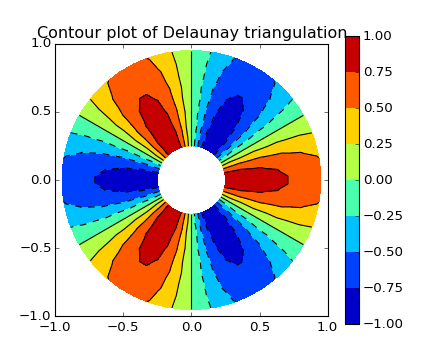(png, hires.png, pdf)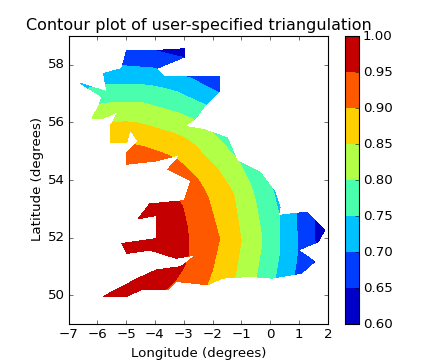(png, hires.png, pdf)

`tricontourf`(*args, **kwargs)

Draw contours on an unstructured triangular grid. `tricontour()` and `tricontourf()` draw contour lines and filled contours, respectively. Except as noted, function signatures and return values are the same for both versions.

The triangulation can be specified in one of two ways; either:

```tricontour(triangulation, ...)
```

where triangulation is a `matplotlib.tri.Triangulation` object, or

```tricontour(x, y, ...)
tricontour(x, y, triangles, ...)
tricontour(x, y, triangles=triangles, ...)
```

in which case a Triangulation object will be created. See `Triangulation` for a explanation of these possibilities.

The remaining arguments may be:

```tricontour(..., Z)
```

where Z is the array of values to contour, one per point in the triangulation. The level values are chosen automatically.

```tricontour(..., Z, N)
```

contour N automatically-chosen levels.

```tricontour(..., Z, V)
```

draw contour lines at the values specified in sequence V

```tricontourf(..., Z, V)
```

fill the (len(V)-1) regions between the values in V

```tricontour(Z, **kwargs)
```

Use keyword args to control colors, linewidth, origin, cmap ... see below for more details.

`C = tricontour(...)` returns a `TriContourSet` object.

Optional keyword arguments:

colors: [ None | string | (mpl_colors) ]

If None, the colormap specified by cmap will be used.

If a string, like ‘r’ or ‘red’, all levels will be plotted in this color.

If a tuple of matplotlib color args (string, float, rgb, etc), different levels will be plotted in different colors in the order specified.

alpha: float
The alpha blending value
cmap: [ None | Colormap ]
A cm `Colormap` instance or None. If cmap is None and colors is None, a default Colormap is used.
norm: [ None | Normalize ]
A `matplotlib.colors.Normalize` instance for scaling data values to colors. If norm is None and colors is None, the default linear scaling is used.
levels [level0, level1, ..., leveln]
A list of floating point numbers indicating the level curves to draw; eg to draw just the zero contour pass `levels=`
origin: [ None | ‘upper’ | ‘lower’ | ‘image’ ]

If None, the first value of Z will correspond to the lower left corner, location (0,0). If ‘image’, the rc value for `image.origin` will be used.

This keyword is not active if X and Y are specified in the call to contour.

extent: [ None | (x0,x1,y0,y1) ]

If origin is not None, then extent is interpreted as in `matplotlib.pyplot.imshow()`: it gives the outer pixel boundaries. In this case, the position of Z[0,0] is the center of the pixel, not a corner. If origin is None, then (x0, y0) is the position of Z[0,0], and (x1, y1) is the position of Z[-1,-1].

This keyword is not active if X and Y are specified in the call to contour.

locator: [ None | ticker.Locator subclass ]
If locator is None, the default `MaxNLocator` is used. The locator is used to determine the contour levels if they are not given explicitly via the V argument.
extend: [ ‘neither’ | ‘both’ | ‘min’ | ‘max’ ]
Unless this is ‘neither’, contour levels are automatically added to one or both ends of the range so that all data are included. These added ranges are then mapped to the special colormap values which default to the ends of the colormap range, but can be set via `matplotlib.colors.Colormap.set_under()` and `matplotlib.colors.Colormap.set_over()` methods.
xunits, yunits: [ None | registered units ]
Override axis units by specifying an instance of a `matplotlib.units.ConversionInterface`.

tricontour-only keyword arguments:

linewidths: [ None | number | tuple of numbers ]

If linewidths is None, the default width in `lines.linewidth` in `matplotlibrc` is used.

If a number, all levels will be plotted with this linewidth.

If a tuple, different levels will be plotted with different linewidths in the order specified

linestyles: [ None | ‘solid’ | ‘dashed’ | ‘dashdot’ | ‘dotted’ ]

If linestyles is None, the ‘solid’ is used.

linestyles can also be an iterable of the above strings specifying a set of linestyles to be used. If this iterable is shorter than the number of contour levels it will be repeated as necessary.

If contour is using a monochrome colormap and the contour level is less than 0, then the linestyle specified in `contour.negative_linestyle` in `matplotlibrc` will be used.

tricontourf-only keyword arguments:

antialiased: [ True | False ]
enable antialiasing
nchunk: [ 0 | integer ]
If 0, no subdivision of the domain. Specify a positive integer to divide the domain into subdomains of roughly nchunk by nchunk points. This may never actually be advantageous, so this option may be removed. Chunking introduces artifacts at the chunk boundaries unless antialiased is False.

Note: tricontourf fills intervals that are closed at the top; that is, for boundaries z1 and z2, the filled region is:

```z1 < z <= z2
```

There is one exception: if the lowest boundary coincides with the minimum value of the z array, then that minimum value will be included in the lowest interval.

Examples:(png, hires.png, pdf)(png, hires.png, pdf)

`tripcolor`(*args, **kwargs)

Create a pseudocolor plot of an unstructured triangular grid.

The triangulation can be specified in one of two ways; either:

```tripcolor(triangulation, ...)
```

where triangulation is a `matplotlib.tri.Triangulation` object, or

```tripcolor(x, y, ...)
tripcolor(x, y, triangles, ...)
tripcolor(x, y, triangles=triangles, ...)
```

in which case a Triangulation object will be created. See `Triangulation` for a explanation of these possibilities.

The next argument must be C, the array of color values, either one per point in the triangulation if color values are defined at points, or one per triangle in the triangulation if color values are defined at triangles. If there are the same number of points and triangles in the triangulation it is assumed that color values are defined at points; to force the use of color values at triangles use the kwarg facecolors*=C instead of just *C.

shading may be ‘flat’ (the default) or ‘gouraud’. If shading is ‘flat’ and C values are defined at points, the color values used for each triangle are from the mean C of the triangle’s three points. If shading is ‘gouraud’ then color values must be defined at points. shading of ‘faceted’ is deprecated; please use edgecolors instead.

The remaining kwargs are the same as for `pcolor()`.

Example: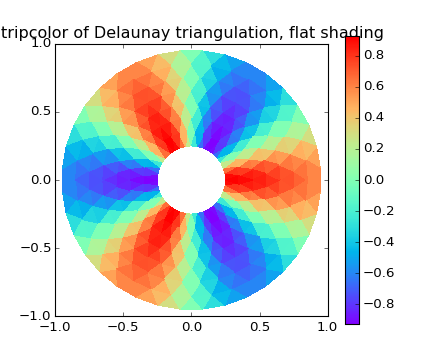(png, hires.png, pdf)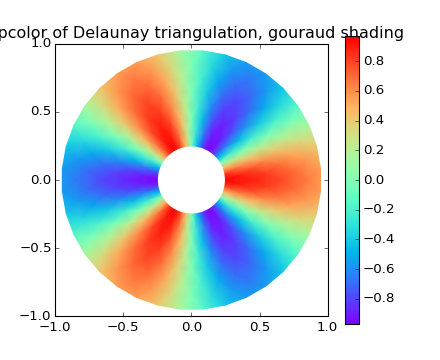(png, hires.png, pdf)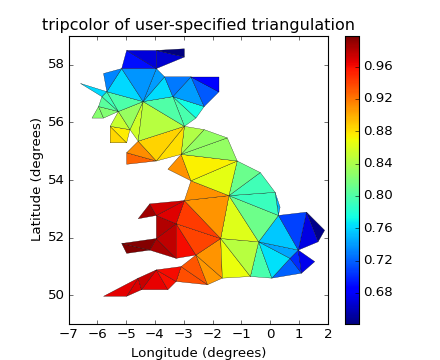(png, hires.png, pdf)

`triplot`(*args, **kwargs)

Draw a unstructured triangular grid as lines and/or markers.

The triangulation to plot can be specified in one of two ways; either:

```triplot(triangulation, ...)
```

where triangulation is a `matplotlib.tri.Triangulation` object, or

```triplot(x, y, ...)
triplot(x, y, triangles, ...)
triplot(x, y, triangles=triangles, ...)
```

in which case a Triangulation object will be created. See `Triangulation` for a explanation of these possibilities.

The remaining args and kwargs are the same as for `plot()`.

Return a list of 2 `Line2D` containing respectively:

• the lines plotted for triangles edges
• the markers plotted for triangles nodes

Example: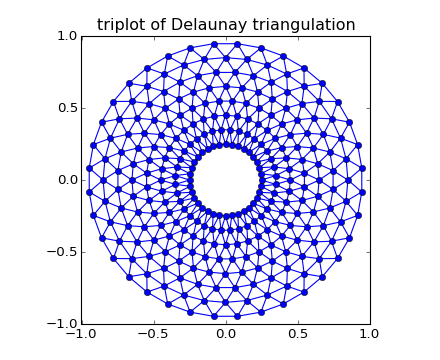(png, hires.png, pdf)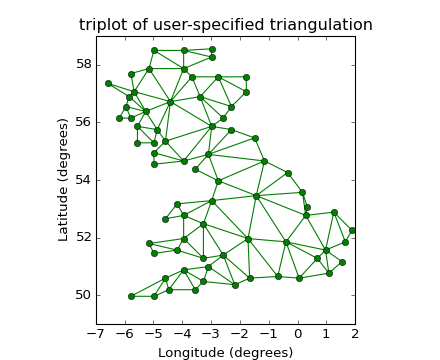(png, hires.png, pdf)

`twinx`()

Call signature:

```ax = twinx()
```

create a twin of Axes for generating a plot with a sharex x-axis but independent y axis. The y-axis of self will have ticks on left and the returned axes will have ticks on the right.

Note

For those who are ‘picking’ artists while using twinx, pick events are only called for the artists in the top-most axes.

`twiny`()

Call signature:

```ax = twiny()
```

create a twin of Axes for generating a plot with a shared y-axis but independent x axis. The x-axis of self will have ticks on bottom and the returned axes will have ticks on the top.

Note

For those who are ‘picking’ artists while using twiny, pick events are only called for the artists in the top-most axes.

`update`(props)

Update the properties of this `Artist` from the dictionary prop.

`update_datalim`(xys, updatex=True, updatey=True)

Update the data lim bbox with seq of xy tups or equiv. 2-D array

`update_datalim_bounds`(bounds)

Update the datalim to include the given `Bbox` bounds

`update_datalim_numerix`(x, y)

Update the data lim bbox with seq of xy tups

`update_from`(other)

Copy properties from other to self.

`violin`(vpstats, positions=None, vert=True, widths=0.5, showmeans=False, showextrema=True, showmedians=False)

Drawing function for violin plots.

Call signature:

```violin(vpstats, positions=None, vert=True, widths=0.5,
showmeans=False, showextrema=True, showmedians=False):
```

Draw a violin plot for each column of `vpstats`. Each filled area extends to represent the entire data range, with optional lines at the mean, the median, the minimum, and the maximum.

Parameters: vpstats : list of dicts A list of dictionaries containing stats for each violin plot. Required keys are: `coords`: A list of scalars containing the coordinates that the violin’s kernel density estimate were evaluated at. `vals`: A list of scalars containing the values of the kernel density estimate at each of the coordinates given in coords. `mean`: The mean value for this violin’s dataset. `median`: The median value for this violin’s dataset. `min`: The minimum value for this violin’s dataset. `max`: The maximum value for this violin’s dataset. positions : array-like, default = [1, 2, ..., n] Sets the positions of the violins. The ticks and limits are automatically set to match the positions. vert : bool, default = True. If true, plots the violins veritcally. Otherwise, plots the violins horizontally. widths : array-like, default = 0.5 Either a scalar or a vector that sets the maximal width of each violin. The default is 0.5, which uses about half of the available horizontal space. showmeans : bool, default = False If true, will toggle rendering of the means. showextrema : bool, default = True If true, will toggle rendering of the extrema. showmedians : bool, default = False If true, will toggle rendering of the medians. result : dict A dictionary mapping each component of the violinplot to a list of the corresponding collection instances created. The dictionary has the following keys: `bodies`: A list of the `matplotlib.collections.PolyCollection` instances containing the filled area of each violin. `means`: A `matplotlib.collections.LineCollection` instance created to identify the mean values of each of the violin’s distribution. `mins`: A `matplotlib.collections.LineCollection` instance created to identify the bottom of each violin’s distribution. `maxes`: A `matplotlib.collections.LineCollection` instance created to identify the top of each violin’s distribution. `bars`: A `matplotlib.collections.LineCollection` instance created to identify the centers of each violin’s distribution. `medians`: A `matplotlib.collections.LineCollection` instance created to identify the median values of each of the violin’s distribution.
`violinplot`(dataset, positions=None, vert=True, widths=0.5, showmeans=False, showextrema=True, showmedians=False, points=100, bw_method=None)

Make a violin plot.

Call signature:

```violinplot(dataset, positions=None, vert=True, widths=0.5,
showmeans=False, showextrema=True, showmedians=False,
points=100, bw_method=None):
```

Make a violin plot for each column of dataset or each vector in sequence dataset. Each filled area extends to represent the entire data range, with optional lines at the mean, the median, the minimum, and the maximum.

Parameters: dataset : Array or a sequence of vectors. The input data. positions : array-like, default = [1, 2, ..., n] Sets the positions of the violins. The ticks and limits are automatically set to match the positions. vert : bool, default = True. If true, creates a vertical violin plot. Otherwise, creates a horizontal violin plot. widths : array-like, default = 0.5 Either a scalar or a vector that sets the maximal width of each violin. The default is 0.5, which uses about half of the available horizontal space. showmeans : bool, default = False If `True`, will toggle rendering of the means. showextrema : bool, default = True If `True`, will toggle rendering of the extrema. showmedians : bool, default = False If `True`, will toggle rendering of the medians. points : scalar, default = 100 Defines the number of points to evaluate each of the gaussian kernel density estimations at. bw_method : str, scalar or callable, optional The method used to calculate the estimator bandwidth. This can be ‘scott’, ‘silverman’, a scalar constant or a callable. If a scalar, this will be used directly as `kde.factor`. If a callable, it should take a `GaussianKDE` instance as its only parameter and return a scalar. If None (default), ‘scott’ is used. result : dict A dictionary mapping each component of the violinplot to a list of the corresponding collection instances created. The dictionary has the following keys: `bodies`: A list of the `matplotlib.collections.PolyCollection` instances containing the filled area of each violin. `means`: A `matplotlib.collections.LineCollection` instance created to identify the mean values of each of the violin’s distribution. `mins`: A `matplotlib.collections.LineCollection` instance created to identify the bottom of each violin’s distribution. `maxes`: A `matplotlib.collections.LineCollection` instance created to identify the top of each violin’s distribution. `bars`: A `matplotlib.collections.LineCollection` instance created to identify the centers of each violin’s distribution. `medians`: A `matplotlib.collections.LineCollection` instance created to identify the median values of each of the violin’s distribution.
`vlines`(x, ymin, ymax, colors=u'k', linestyles=u'solid', label=u'', **kwargs)

Plot vertical lines.

Plot vertical lines at each `x` from `ymin` to `ymax`.

Parameters: Returns: x : scalar or 1D array_like x-indexes where to plot the lines. ymin, ymax : scalar or 1D array_like Respective beginning and end of each line. If scalars are provided, all lines will have same length. colors : array_like of colors, optional, default: ‘k’ linestyles : [‘solid’ | ‘dashed’ | ‘dashdot’ | ‘dotted’], optional label : string, optional, default: ‘’ lines : `LineCollection` kwargs : `LineCollection` properties.

`hlines`
horizontal lines

Examples`xaxis_date`(tz=None)

Sets up x-axis ticks and labels that treat the x data as dates.

tz is a timezone string or `tzinfo` instance. Defaults to rc value.

`xaxis_inverted`()

Returns True if the x-axis is inverted.

`xcorr`(x, y, normed=True, detrend=<function detrend_none at 0x7fd543b15c08>, usevlines=True, maxlags=10, **kwargs)

Plot the cross correlation between x and y.

Parameters: Returns: x : sequence of scalars of length n y : sequence of scalars of length n hold : boolean, optional, default: True detrend : callable, optional, default: `mlab.detrend_none` x is detrended by the `detrend` callable. Default is no normalization. normed : boolean, optional, default: True if True, normalize the data by the autocorrelation at the 0-th lag. usevlines : boolean, optional, default: True if True, Axes.vlines is used to plot the vertical lines from the origin to the acorr. Otherwise, Axes.plot is used. maxlags : integer, optional, default: 10 number of lags to show. If None, will return all 2 * len(x) - 1 lags. (lags, c, line, b) : where: `lags` are a length 2`maxlags+1 lag vector. `c` is the 2`maxlags+1 auto correlation vectorI `line` is a `Line2D` instance returned by `plot`. `b` is the x-axis (none, if plot is used). linestyle : `Line2D` prop, optional, default: None Only used if usevlines is False. marker : string, optional, default: ‘o’

Notes

The cross correlation is performed with `numpy.correlate()` with `mode` = 2.

`yaxis_date`(tz=None)

Sets up y-axis ticks and labels that treat the y data as dates.

tz is a timezone string or `tzinfo` instance. Defaults to rc value.

`yaxis_inverted`()

Returns True if the y-axis is inverted.

`zorder` = 0